# R S Aggarwal Solutions for Class 11 Maths Chapter 6- Linear Inequations (In One Variable)

R S Aggarwal Solutions are useful for students as it helps them in scoring high marks in the examination. The R S Aggarwal Solutions given in this page contains detailed step-by-step explanations to all the problems that come under Chapter 6, Linear Inequations (In One Variable), of the Class 11 R S Aggarwal Textbook. This chapter deals with the concepts and topics related to Linear Inequations In One Variable.

These solutions are prepared by subject matter experts at BYJU’S, describing the complete method of solving problems. By understanding the concepts used in R S Aggarwal Solutions for Class 11 Maths, students will be able to clear all their doubts related to “Linear Inequations (In One Variable)”.

### Download PDF of R S Aggarwal Solutions for Class 11 Maths Chapter 6- Linear Inequations (In One Variable)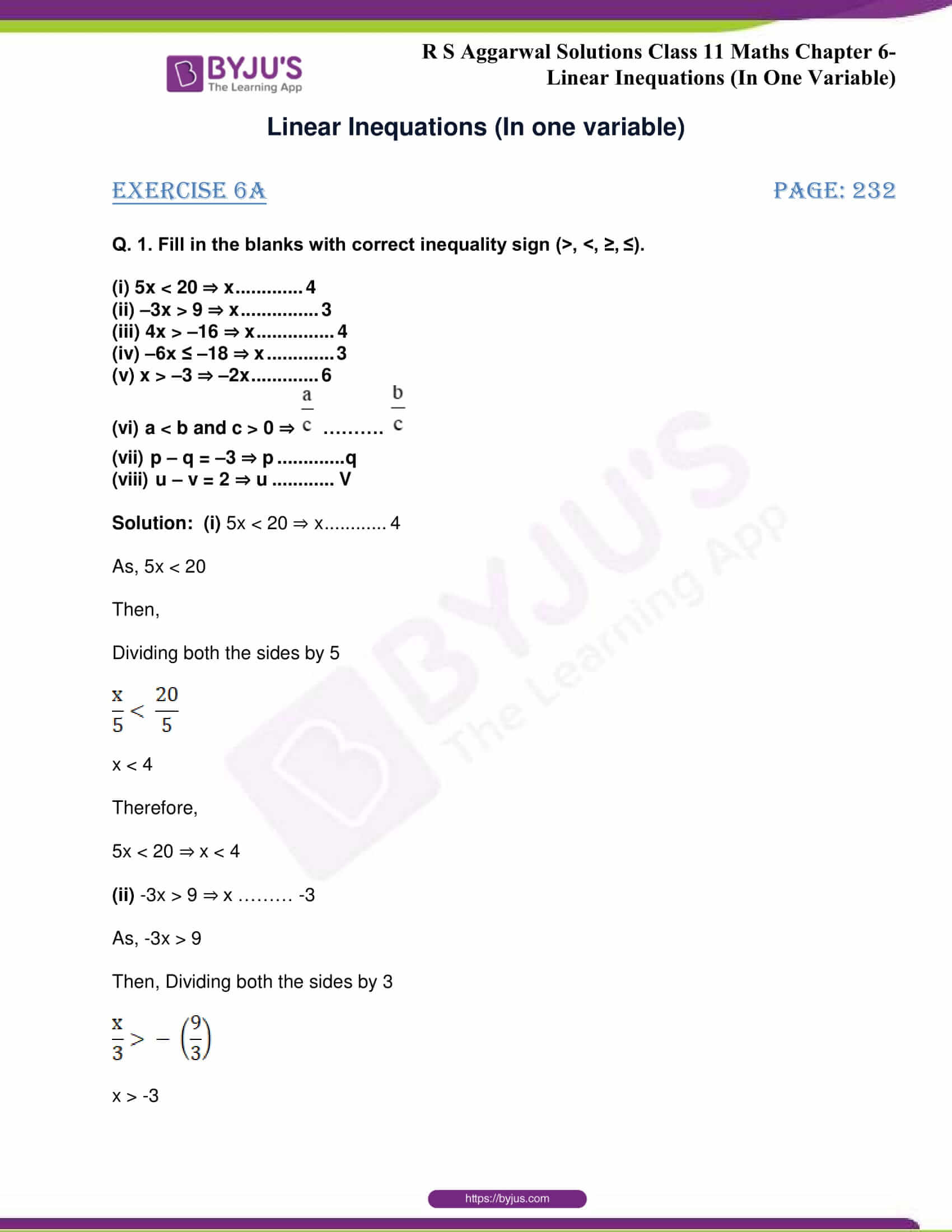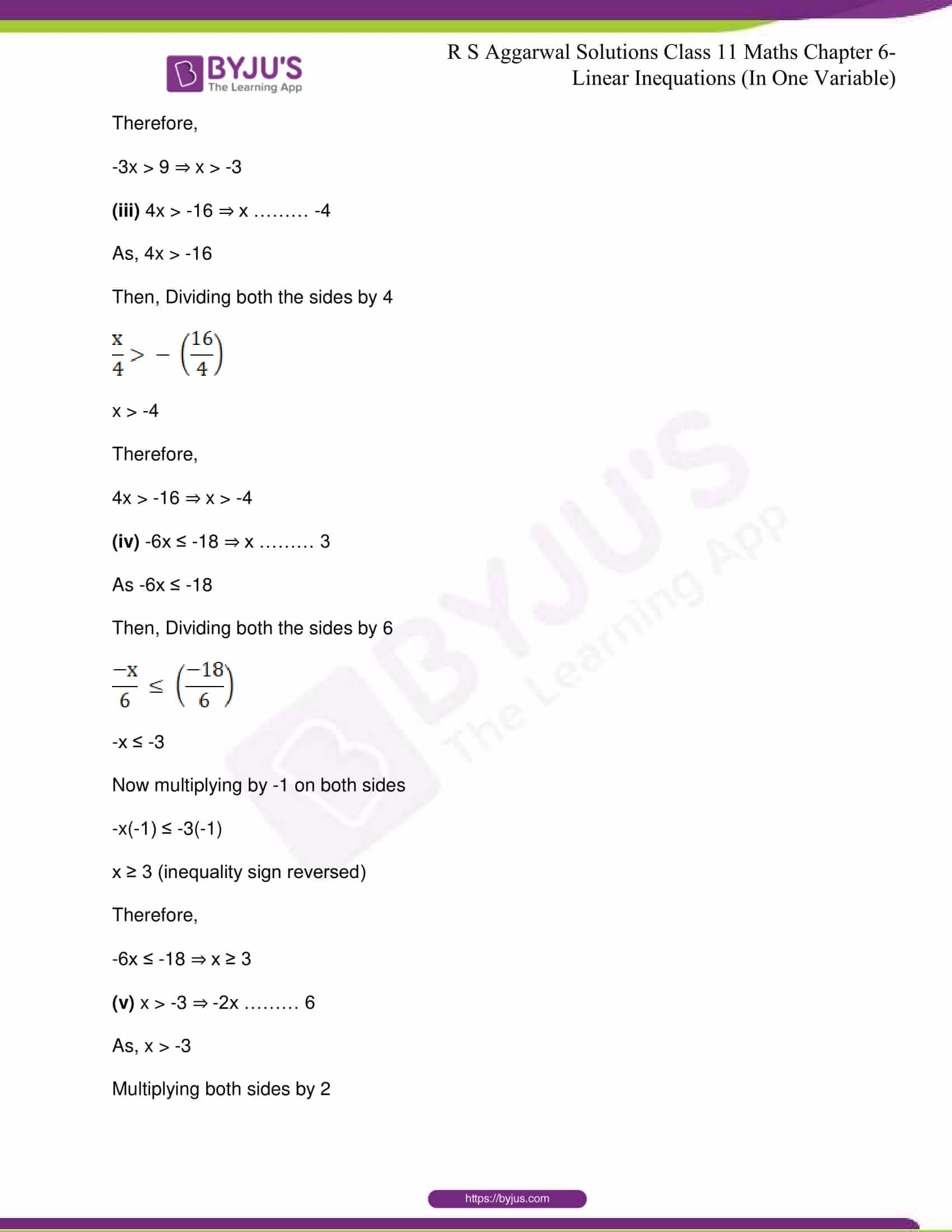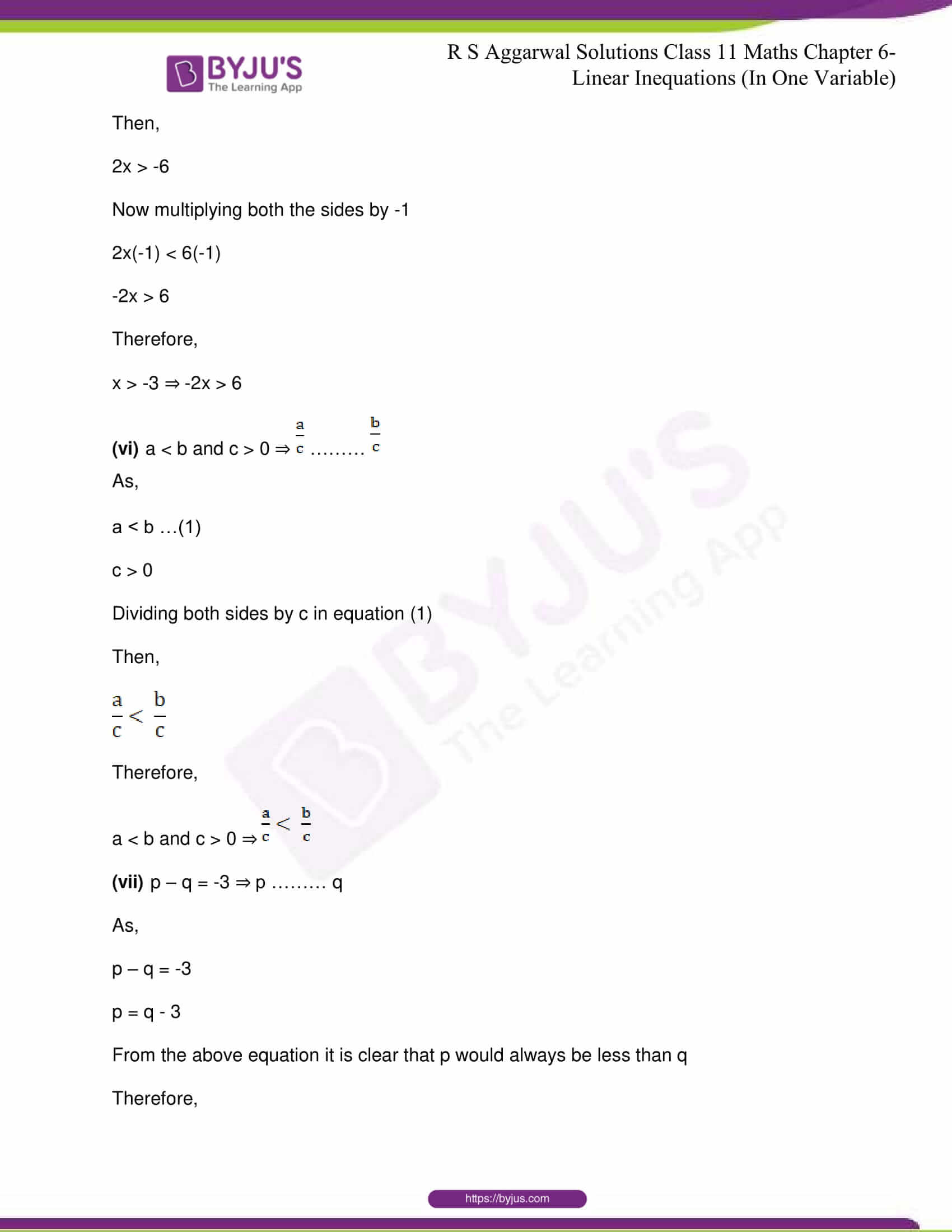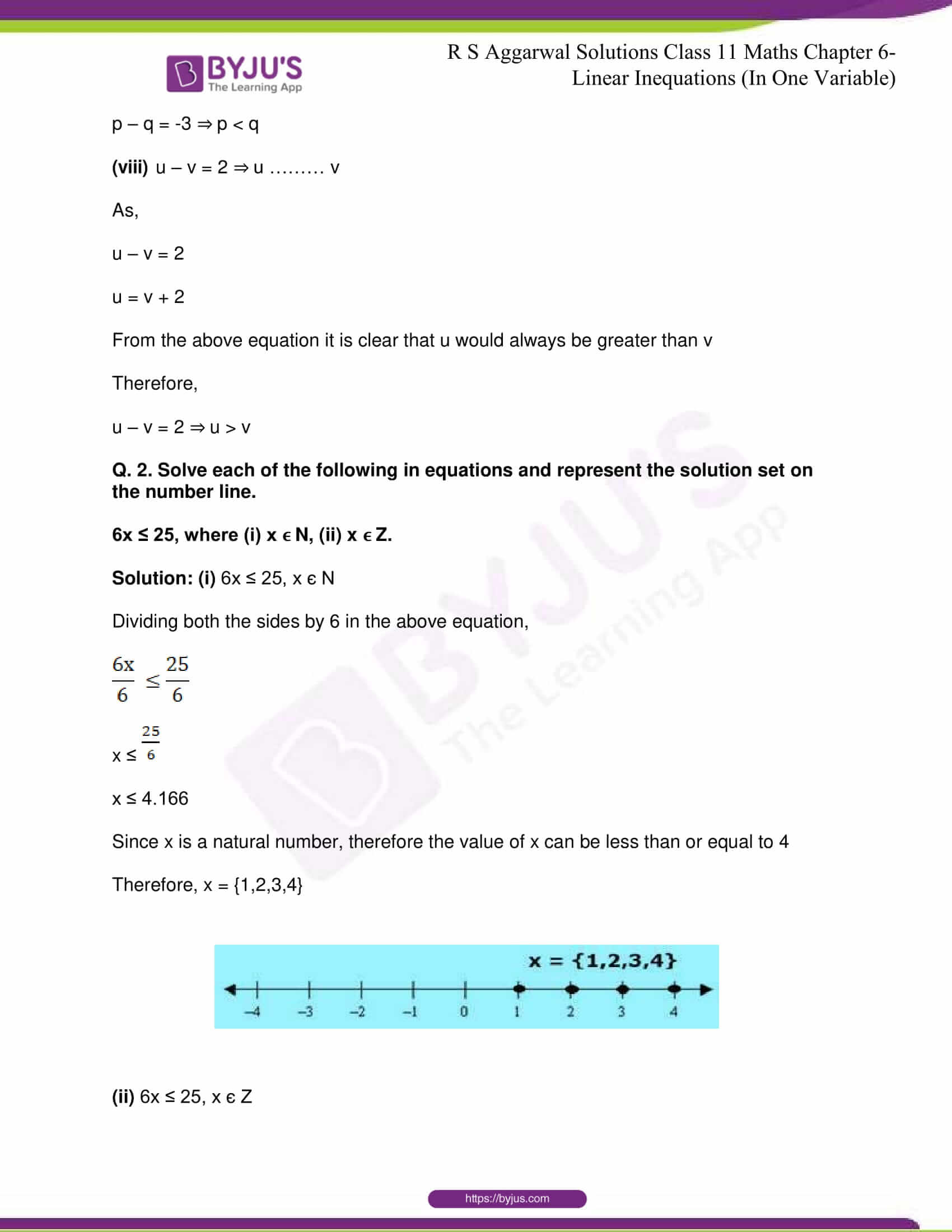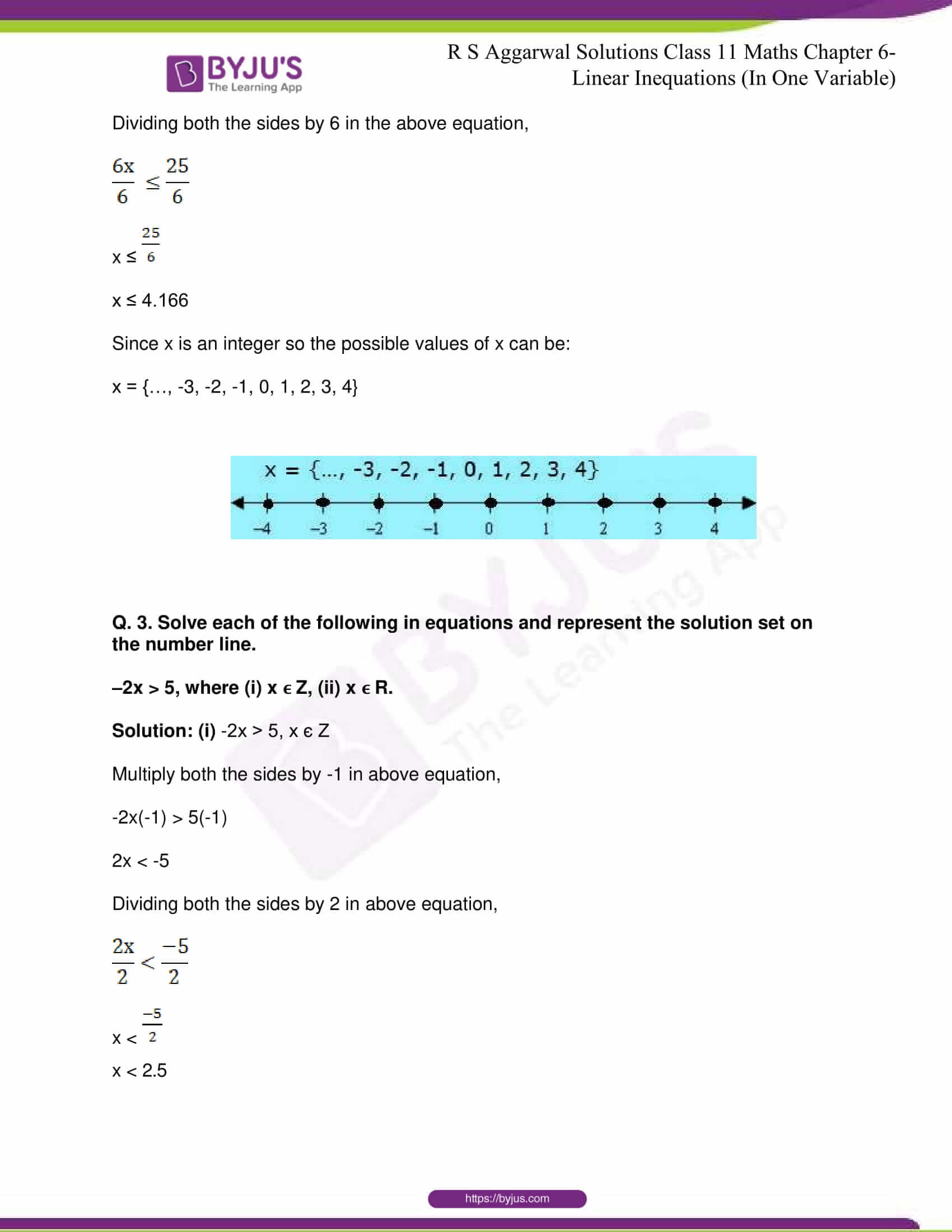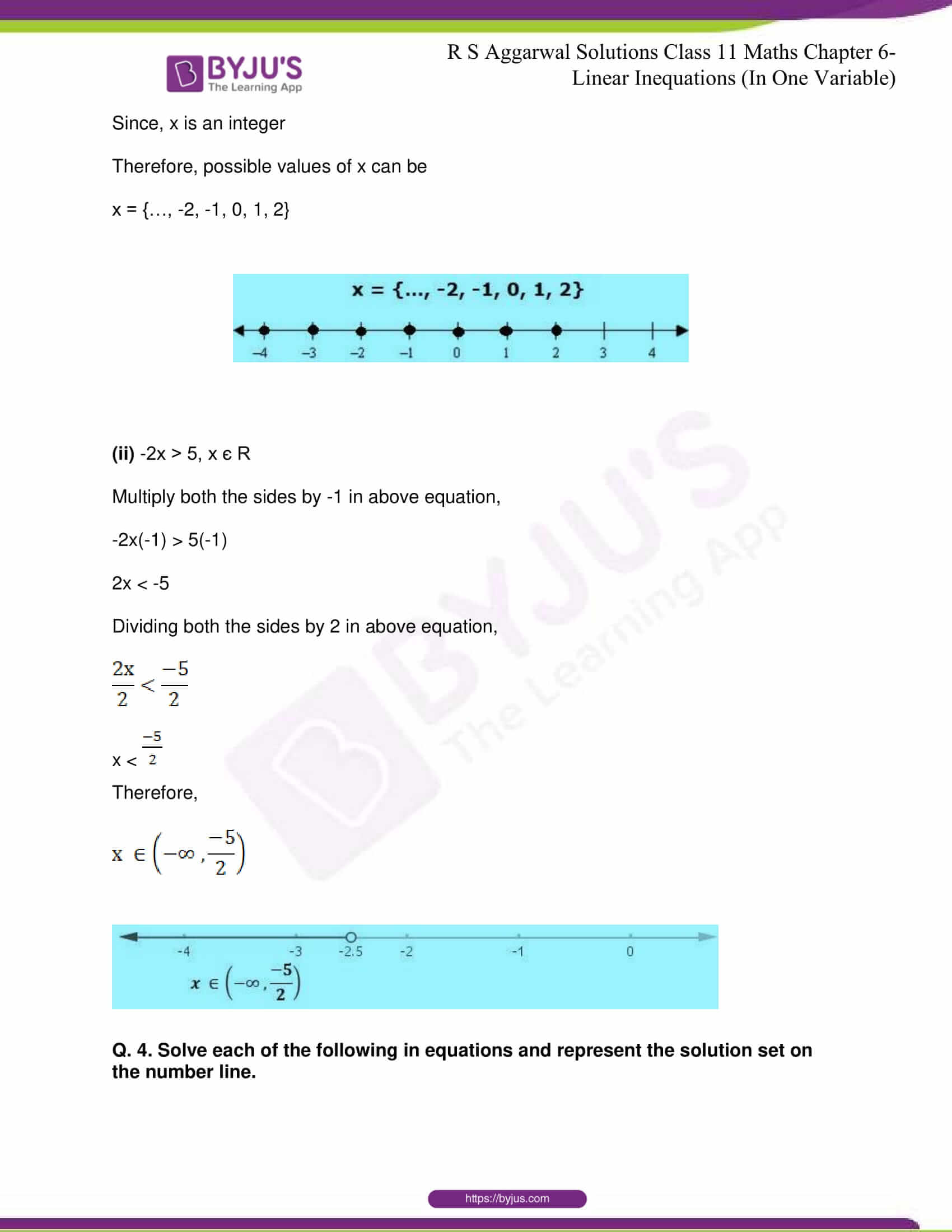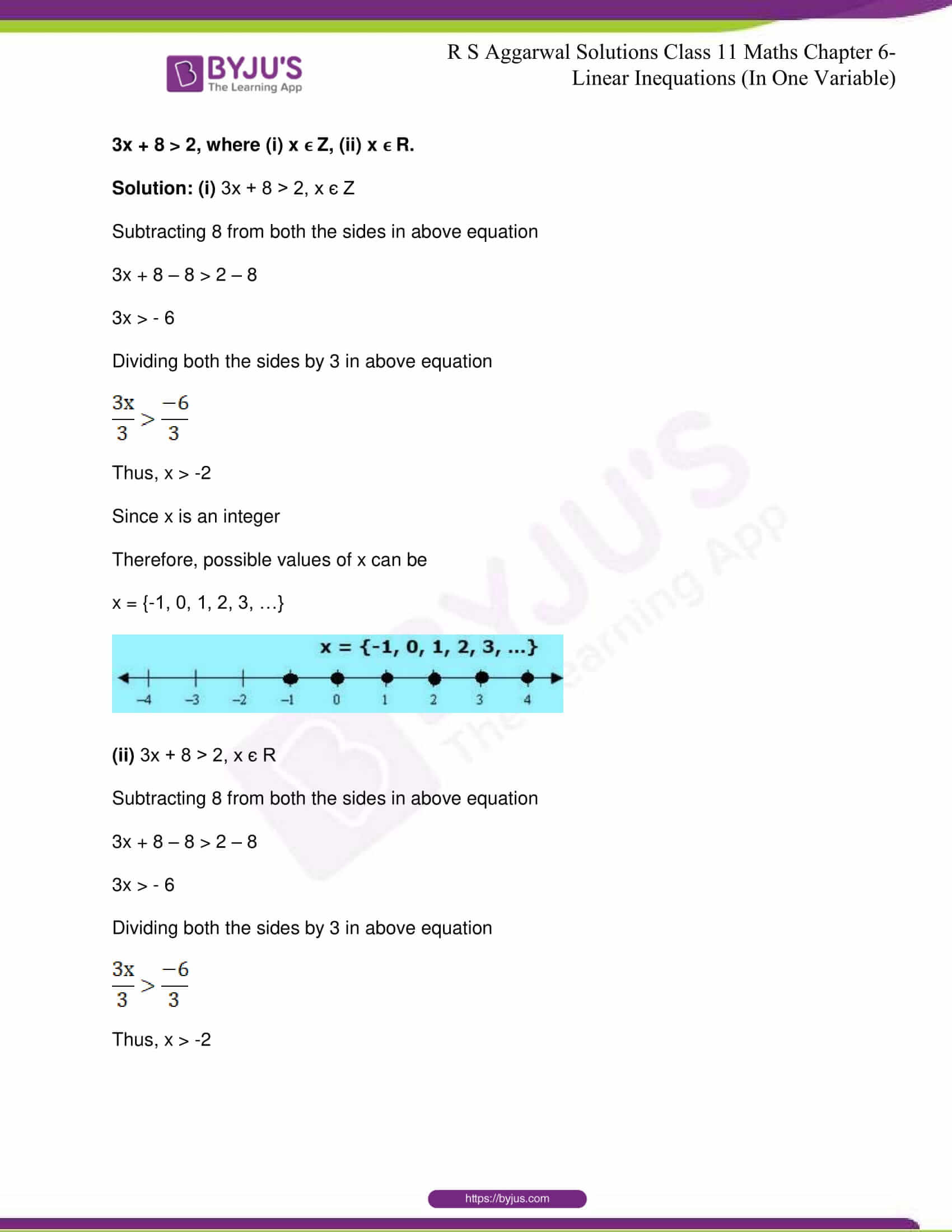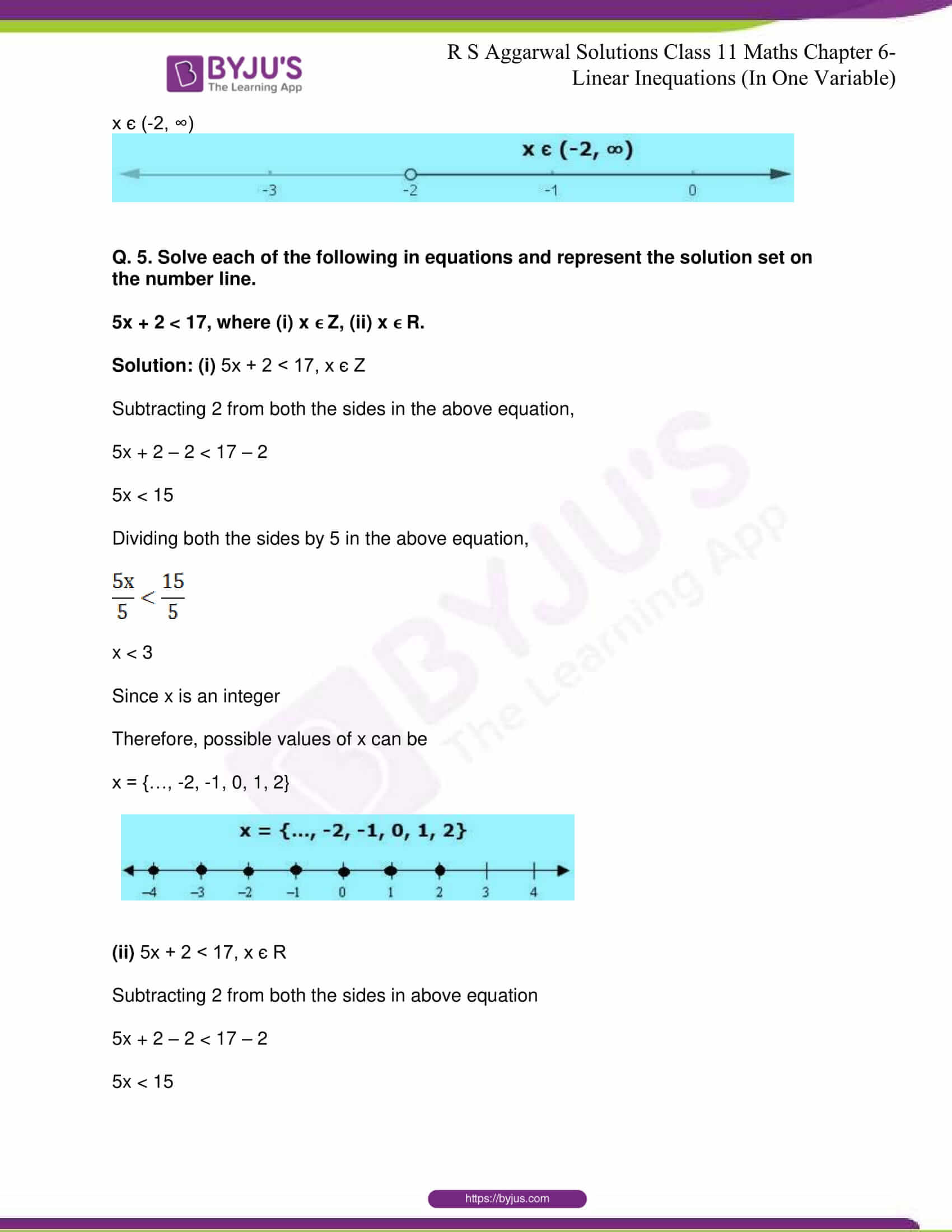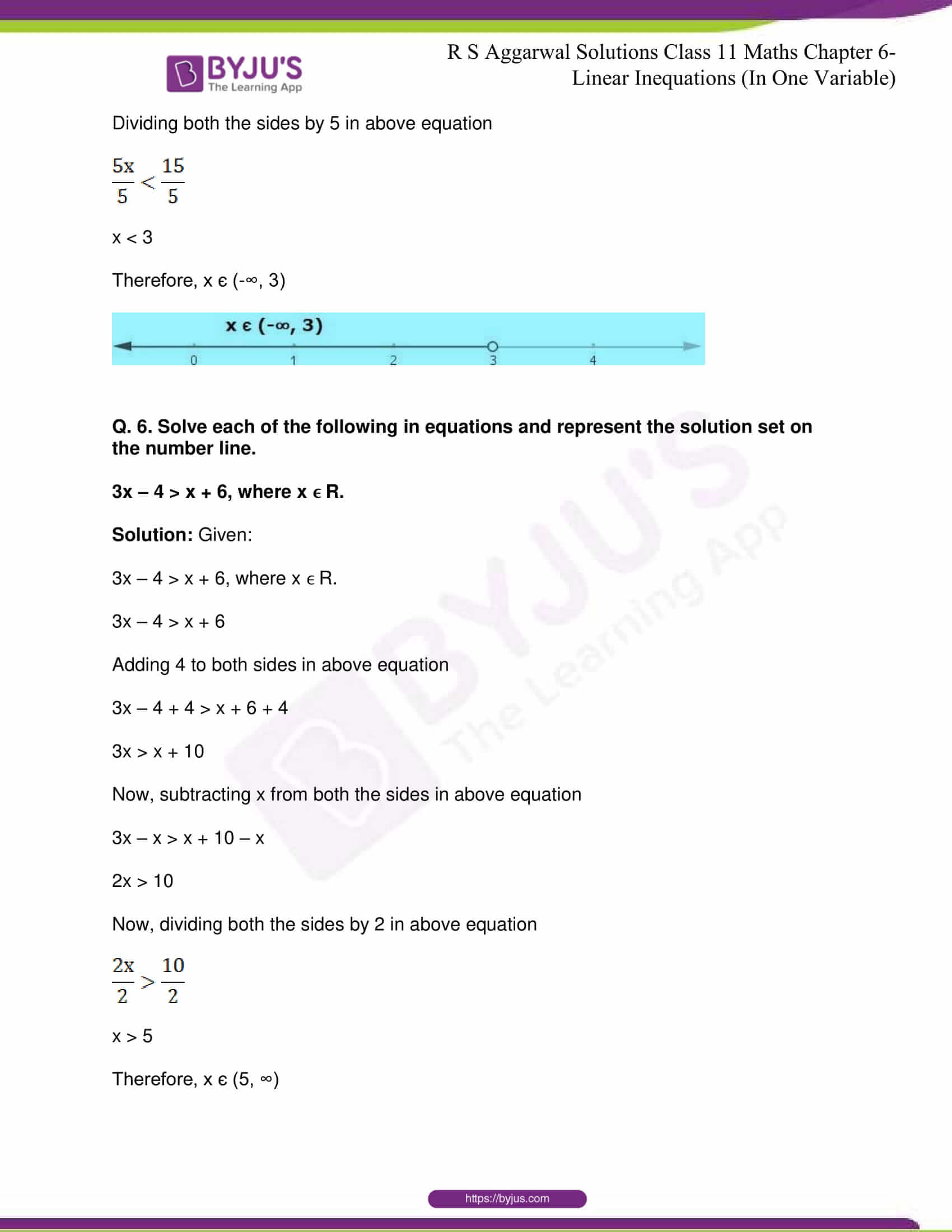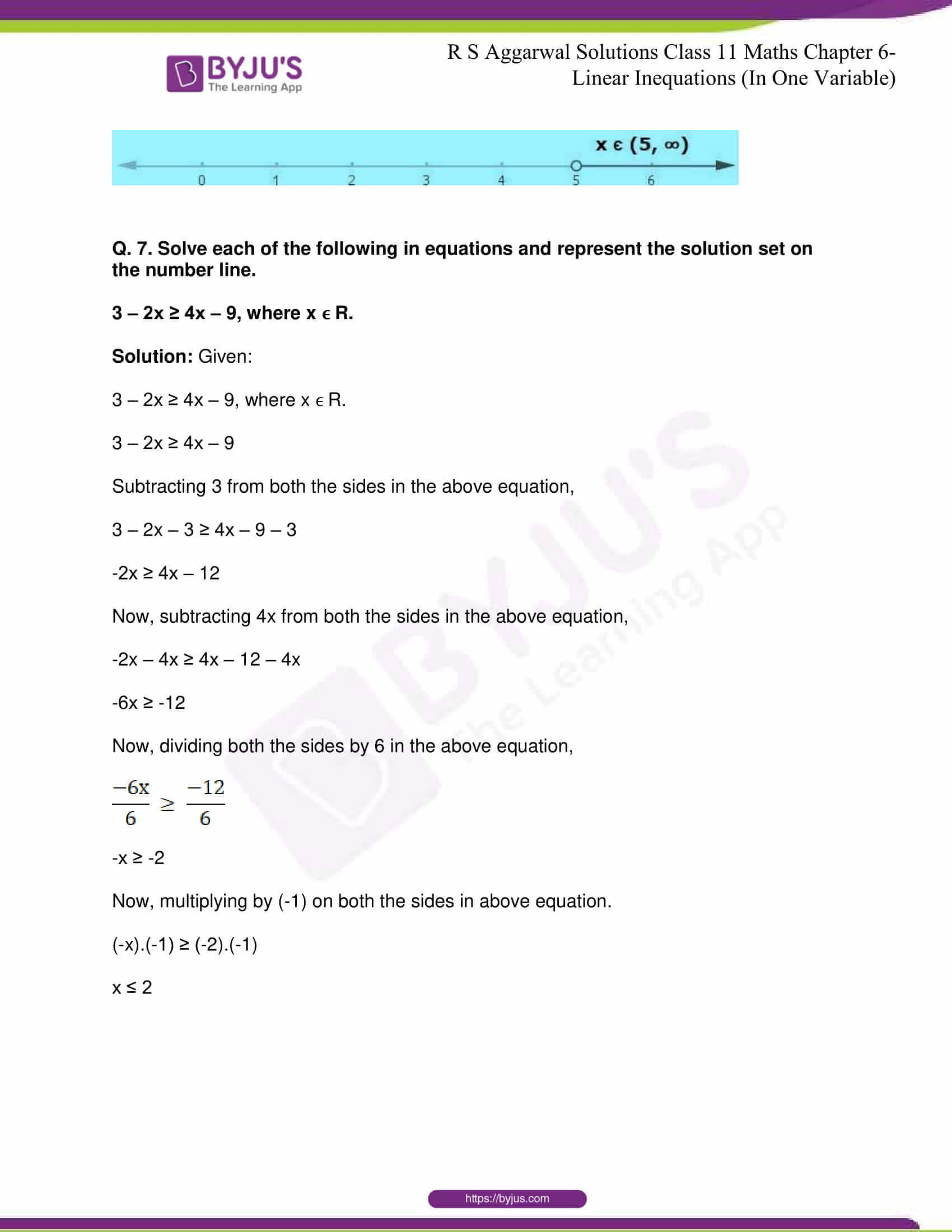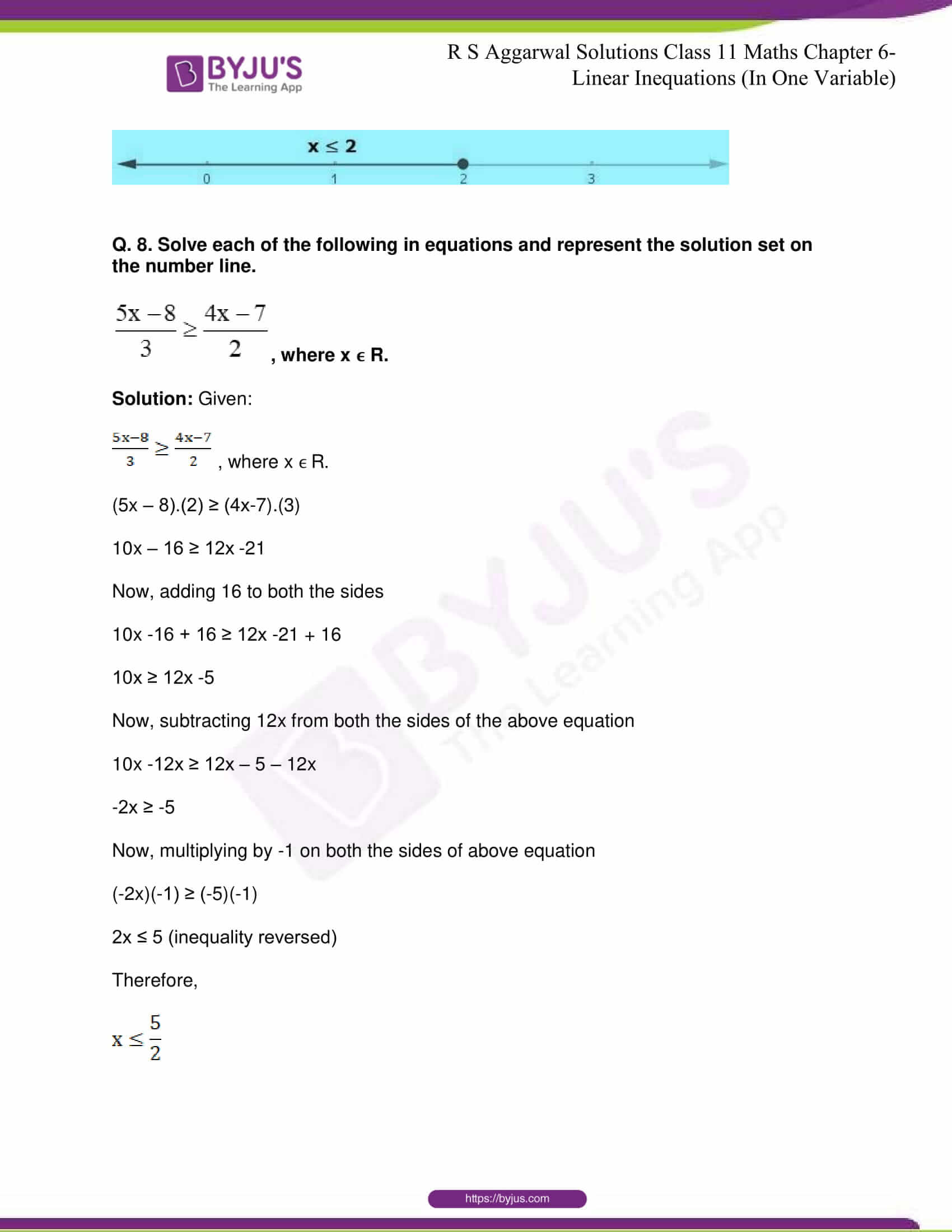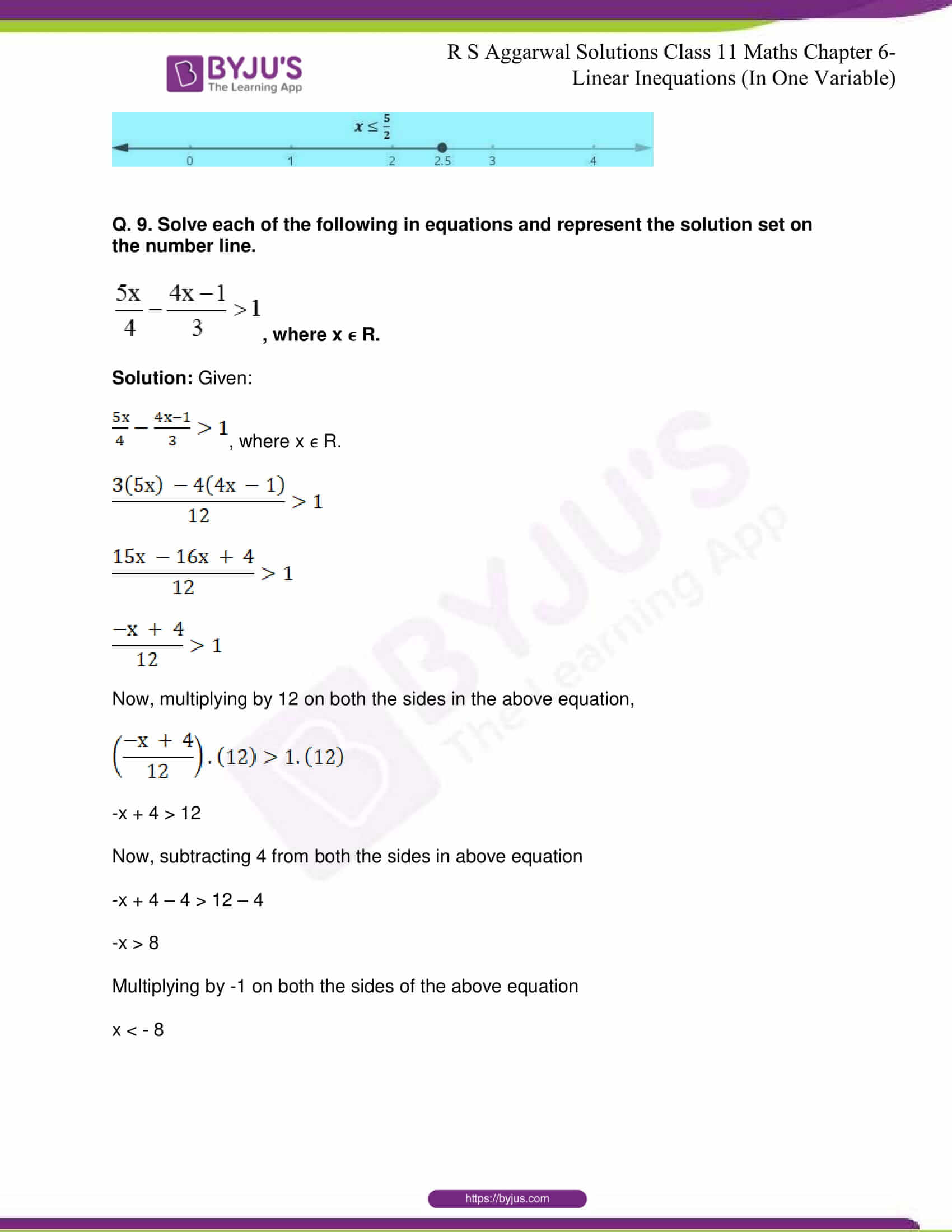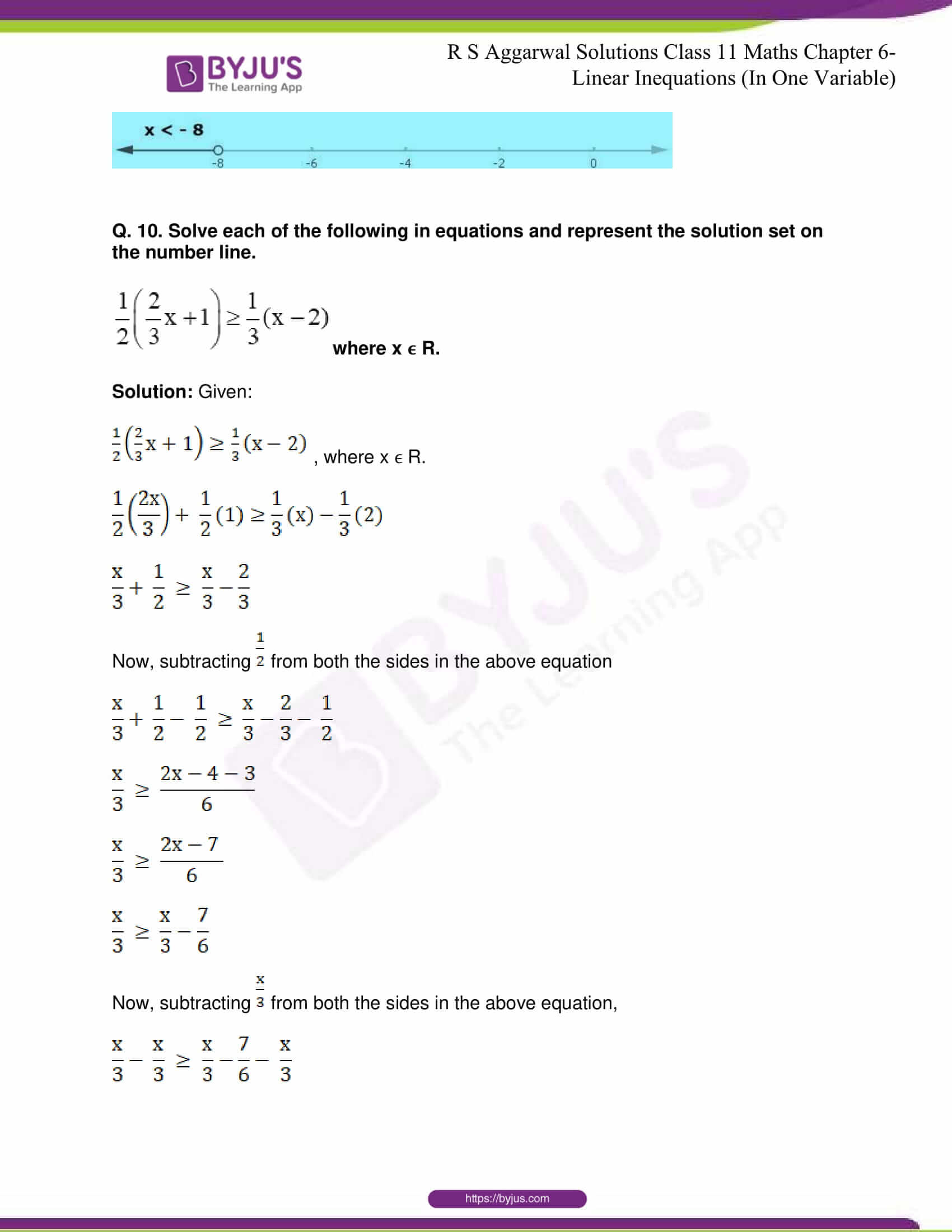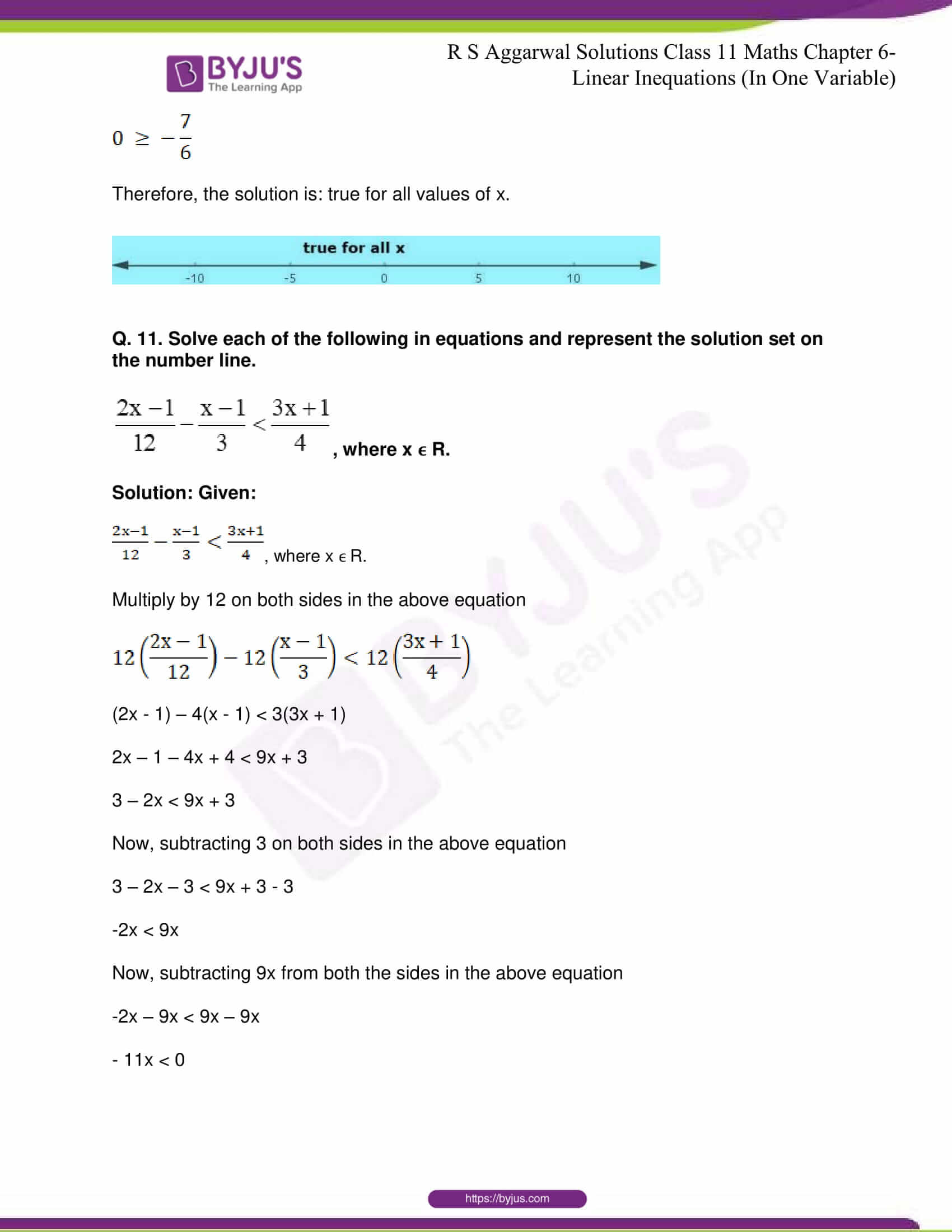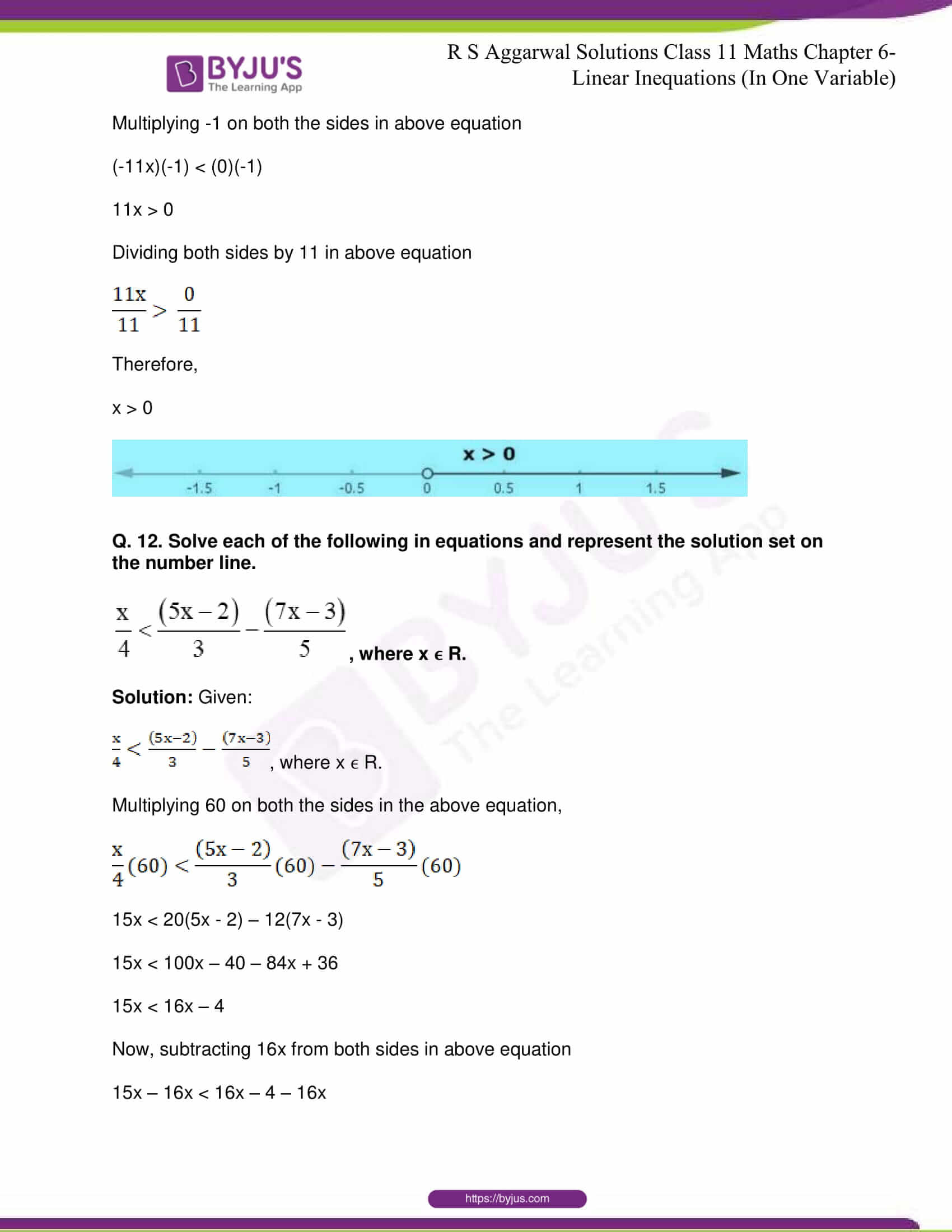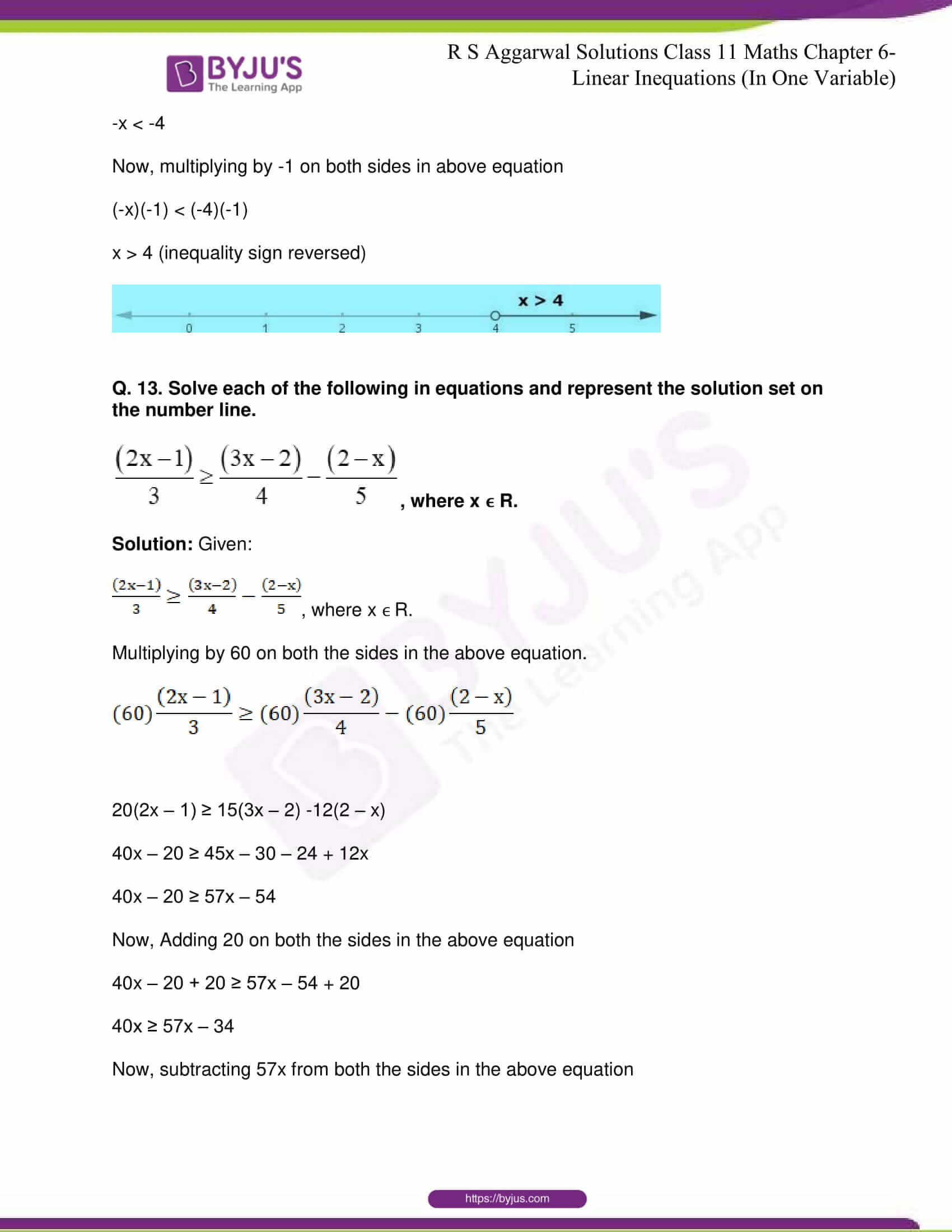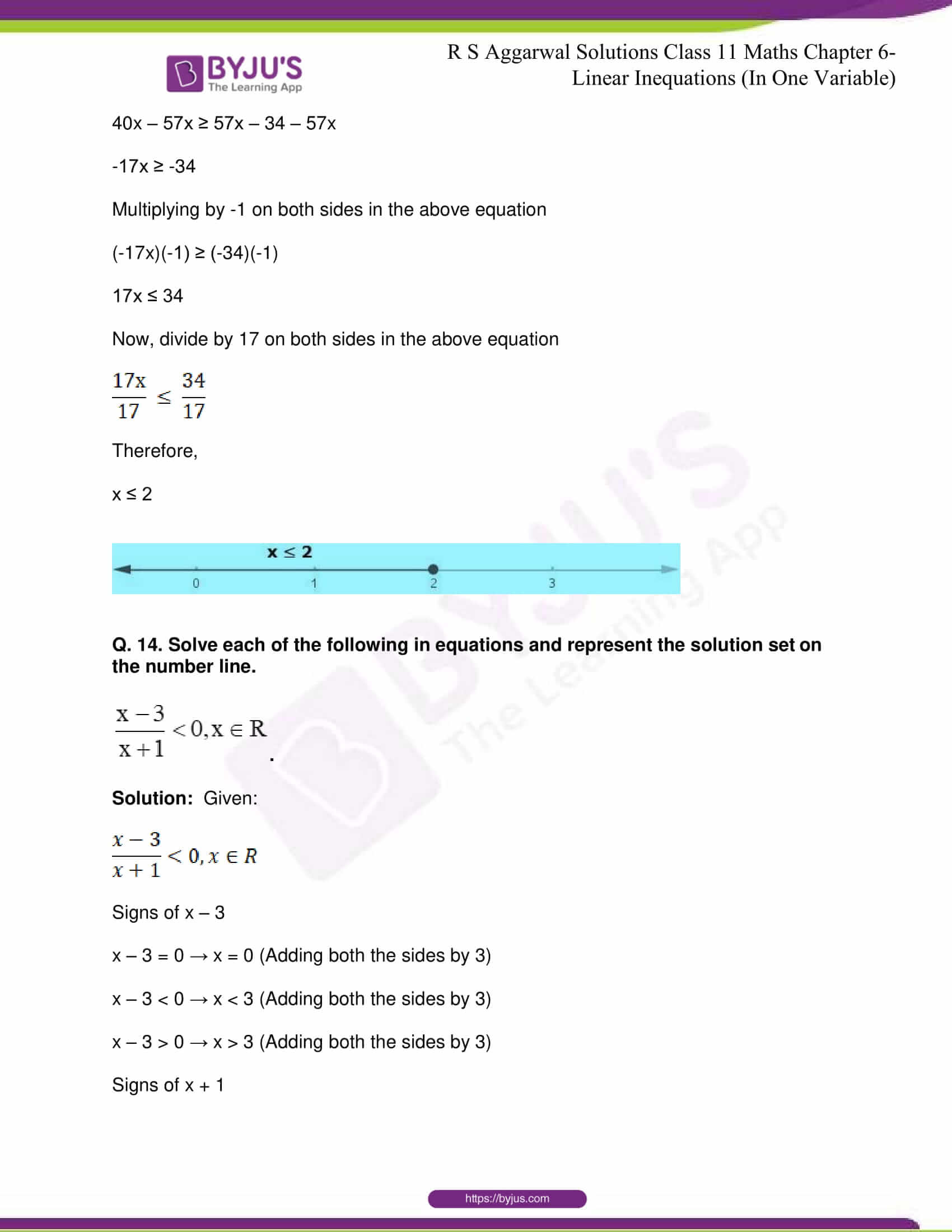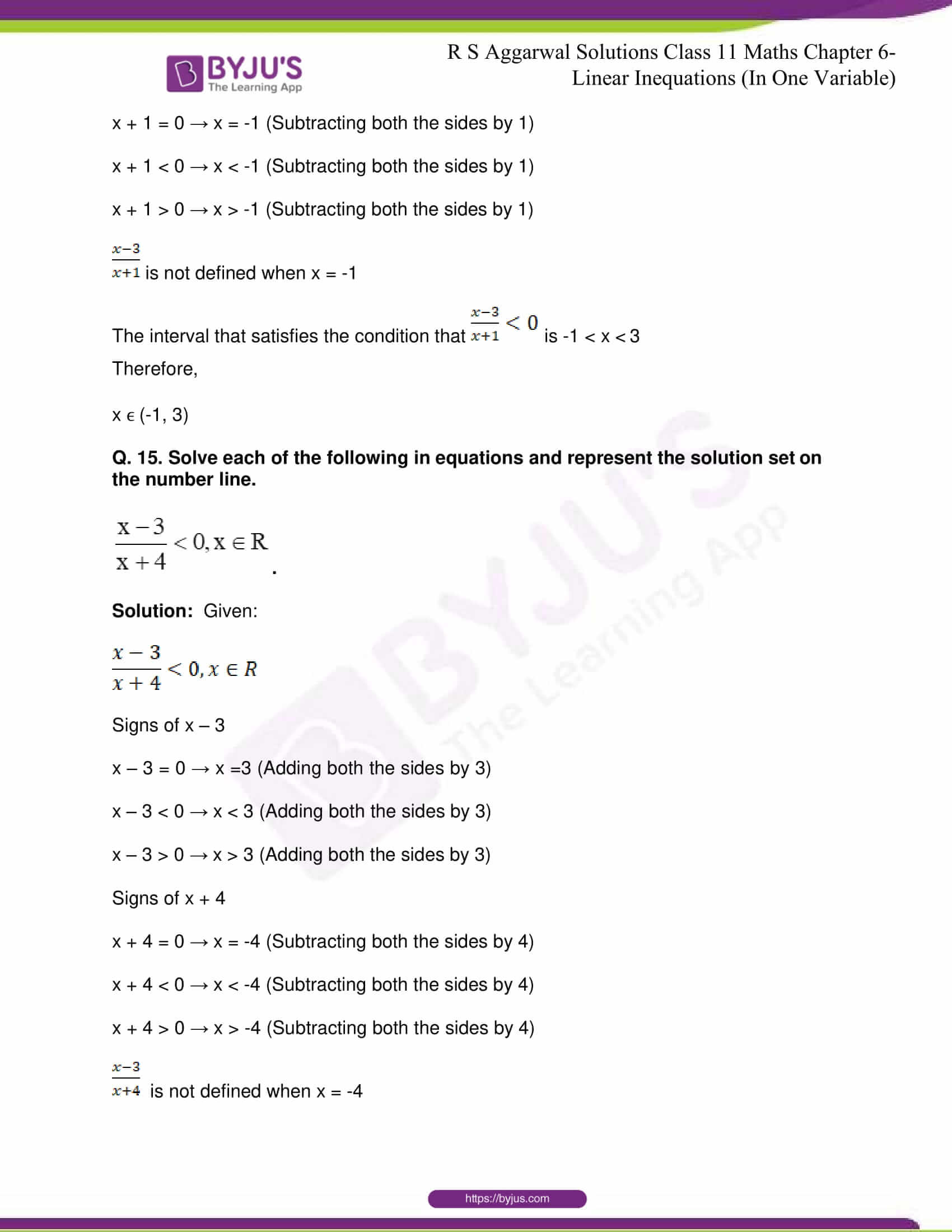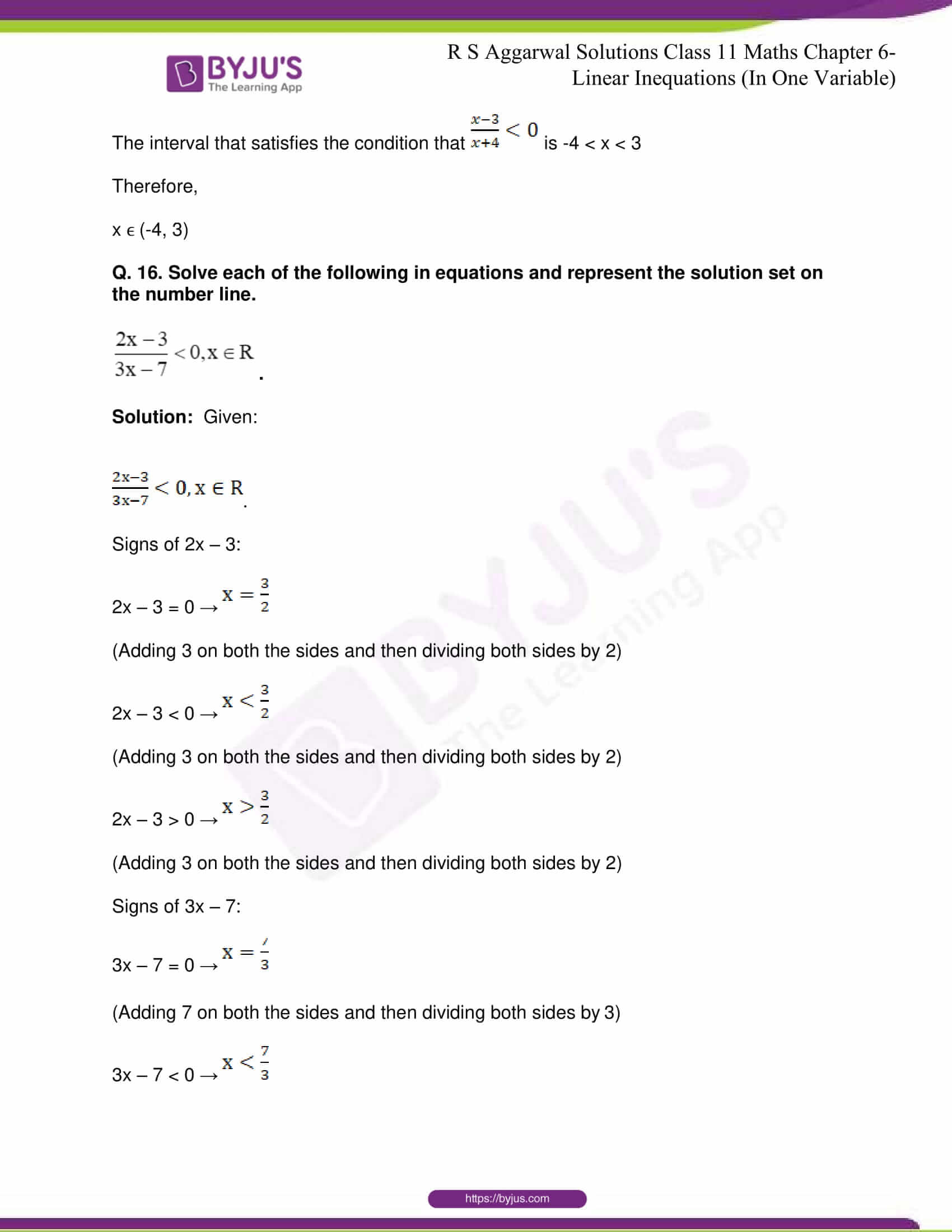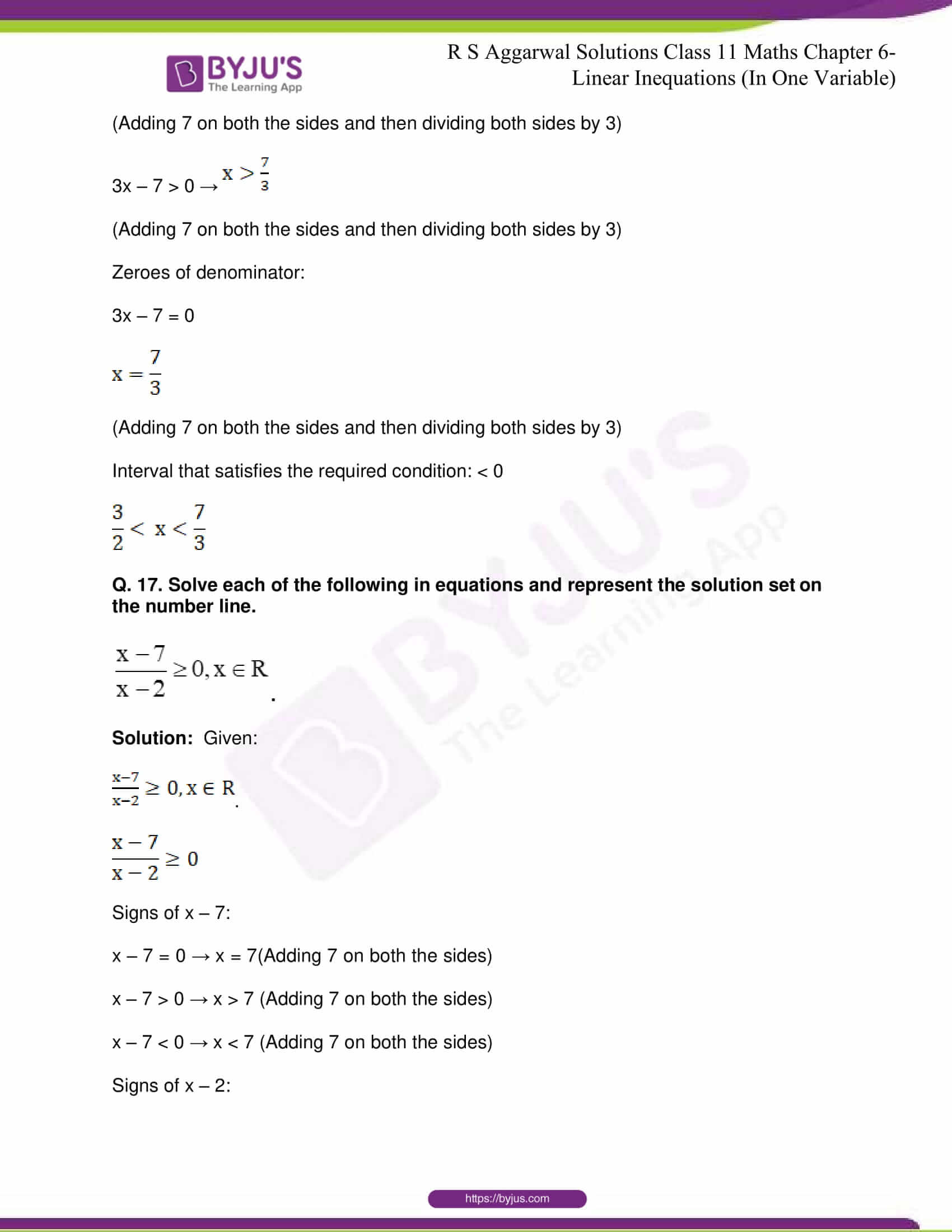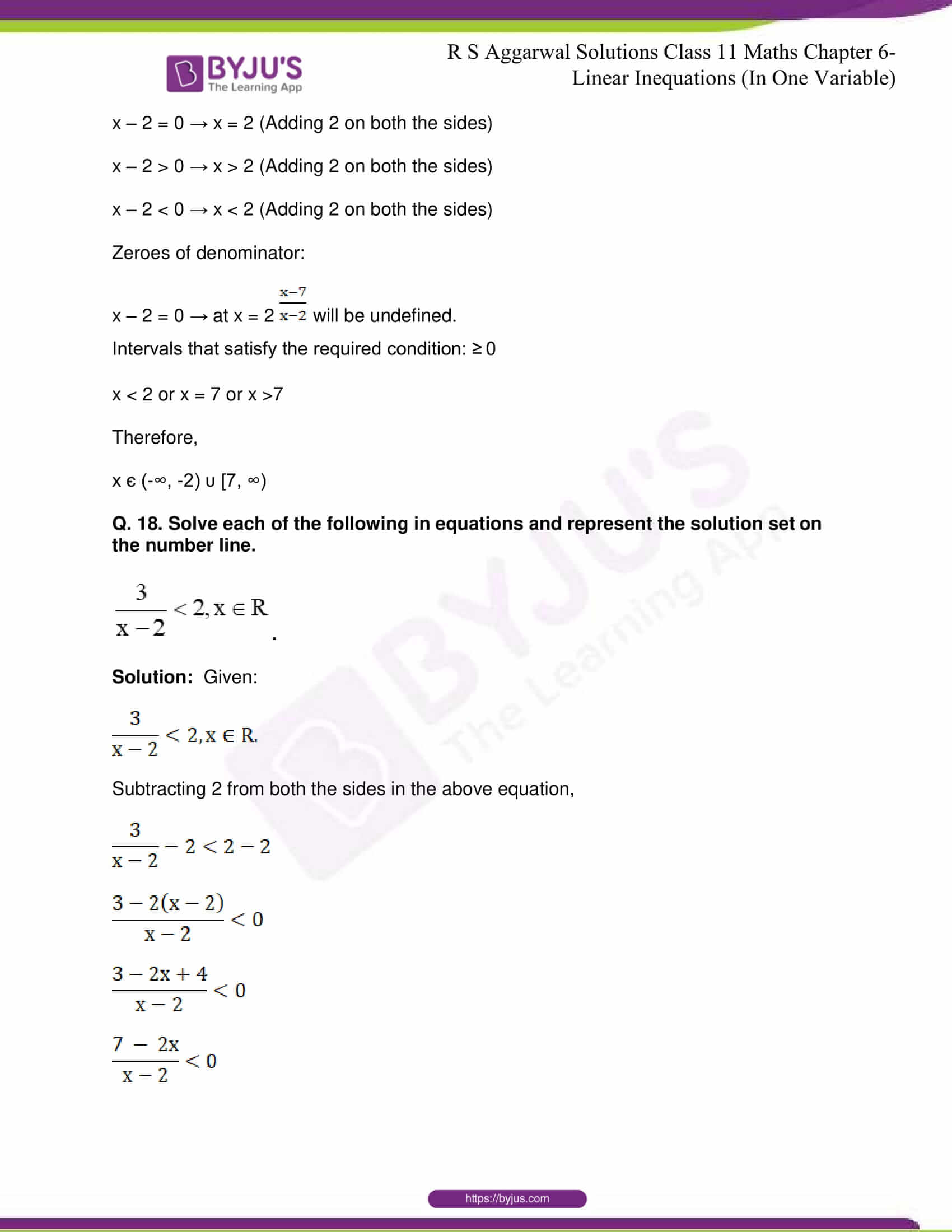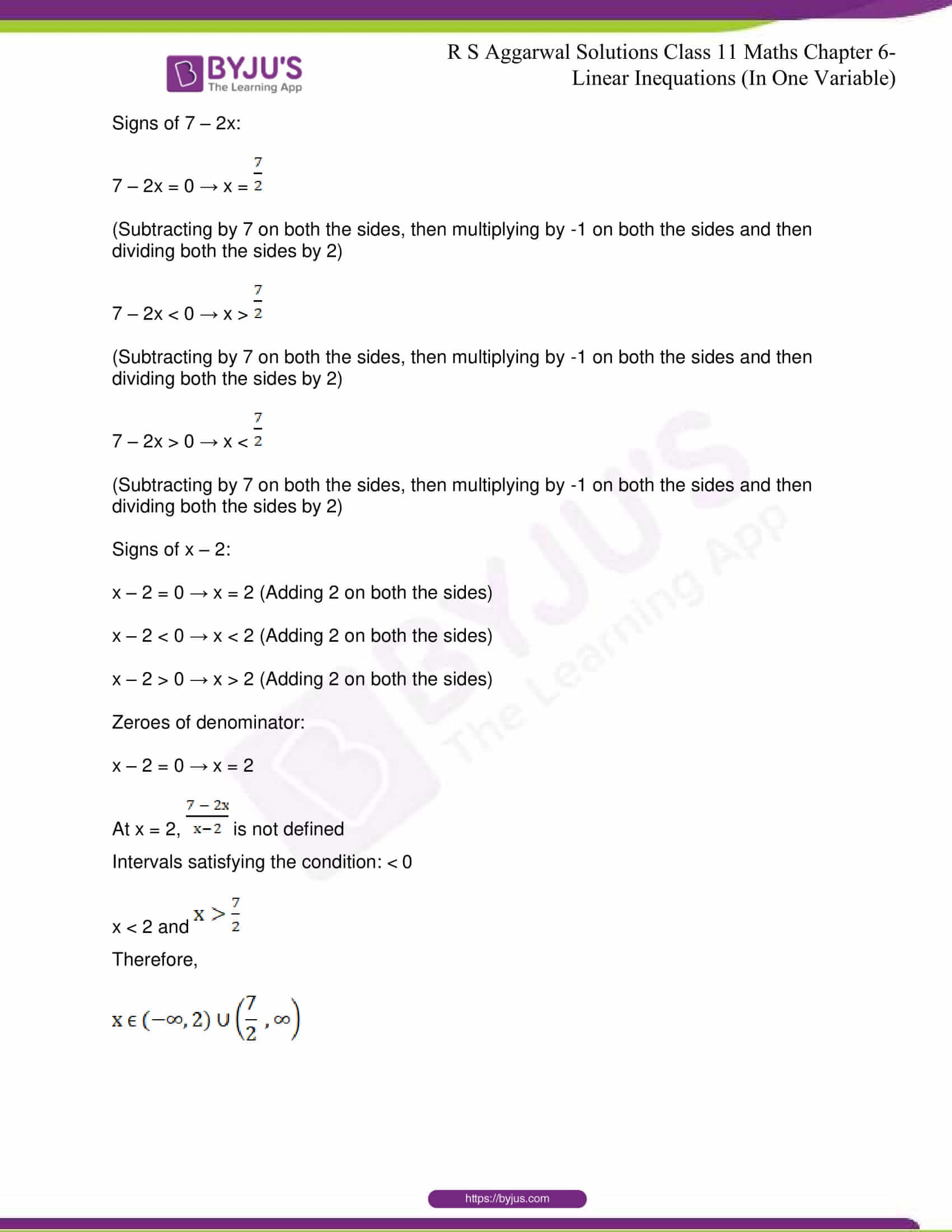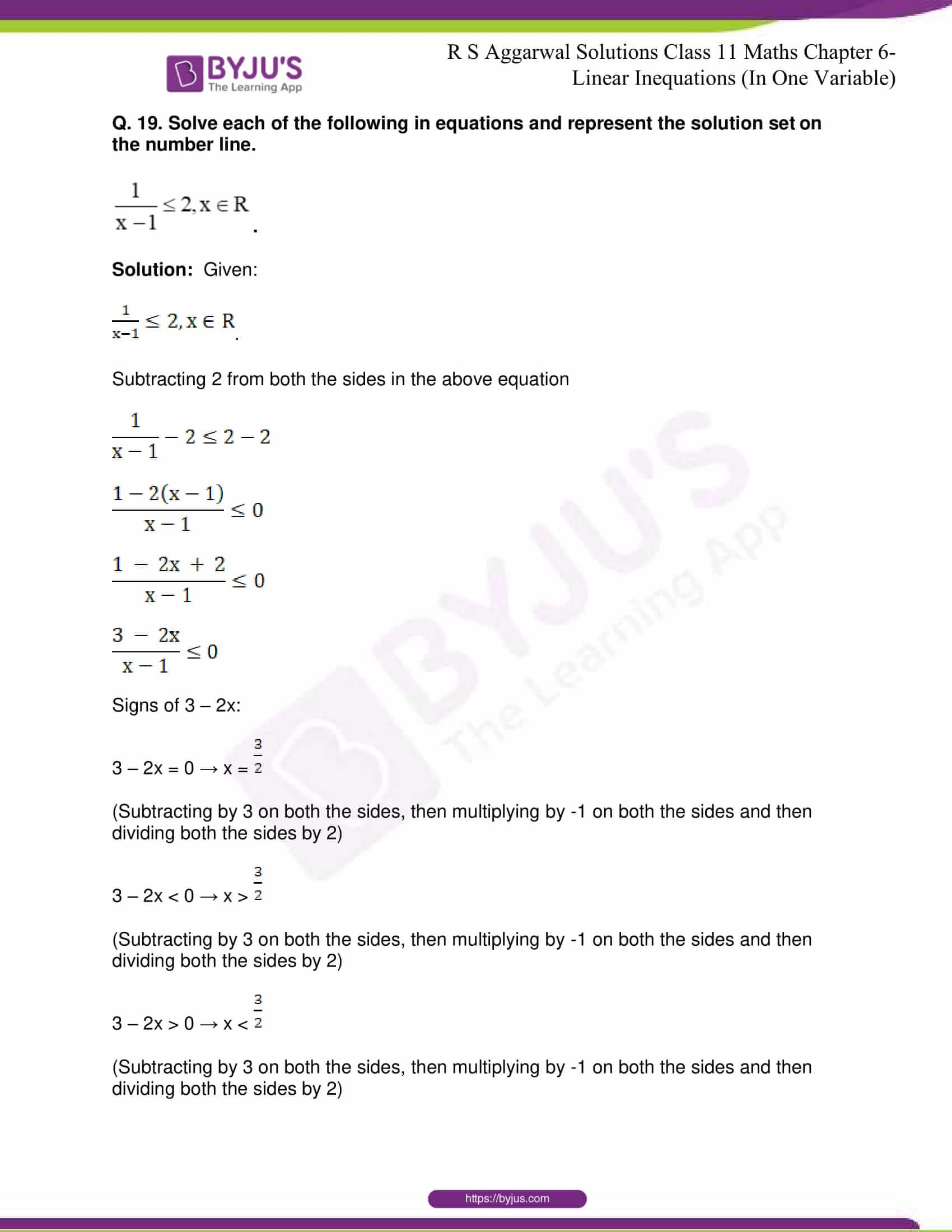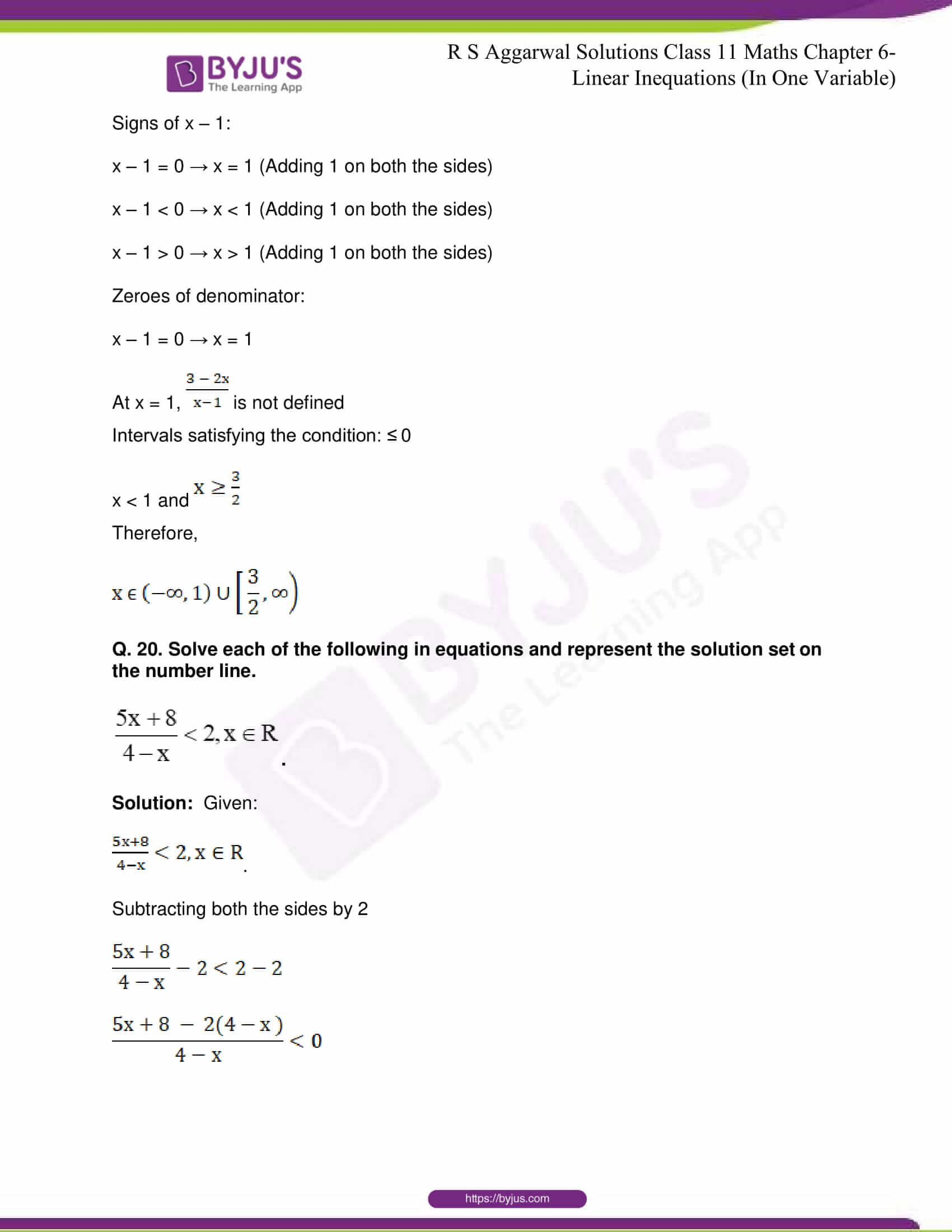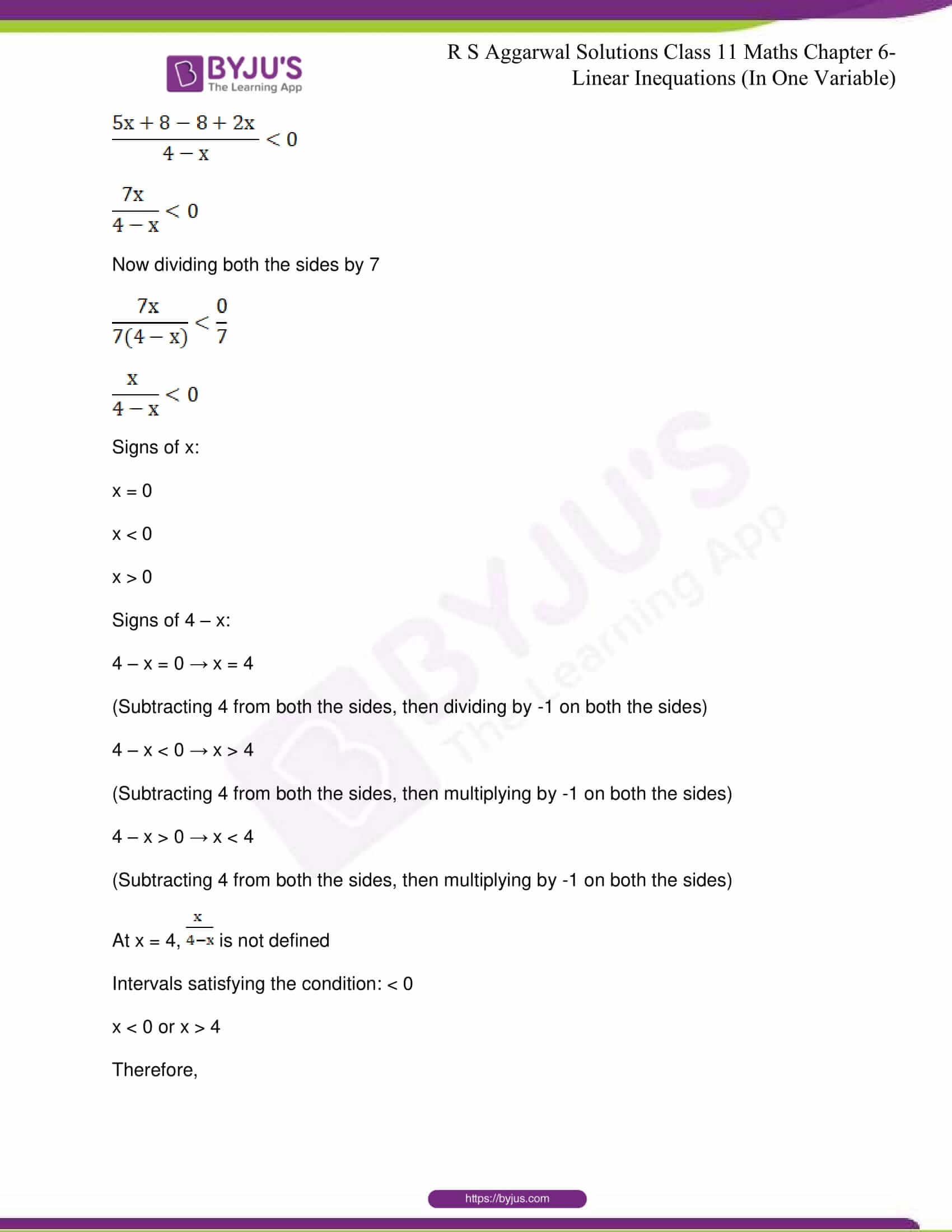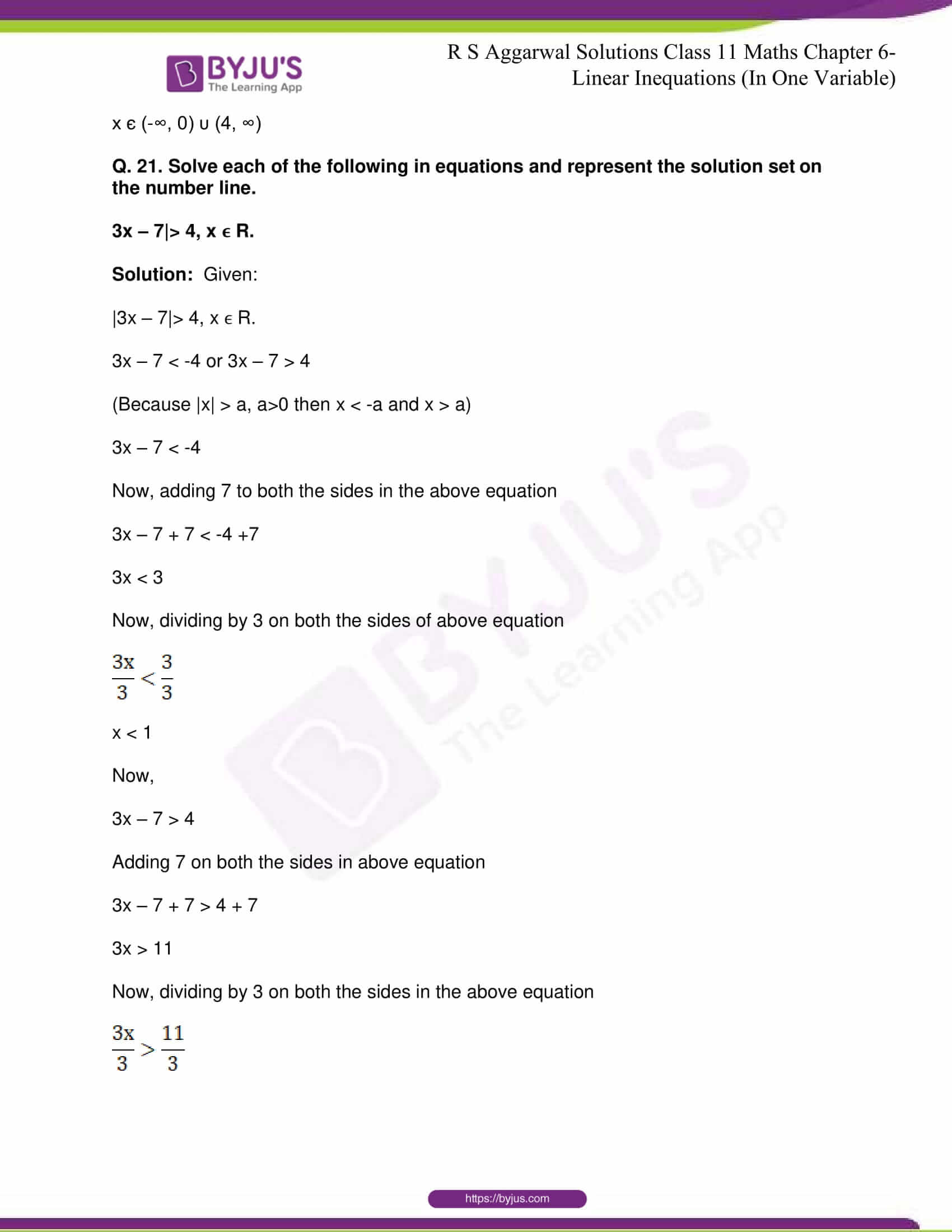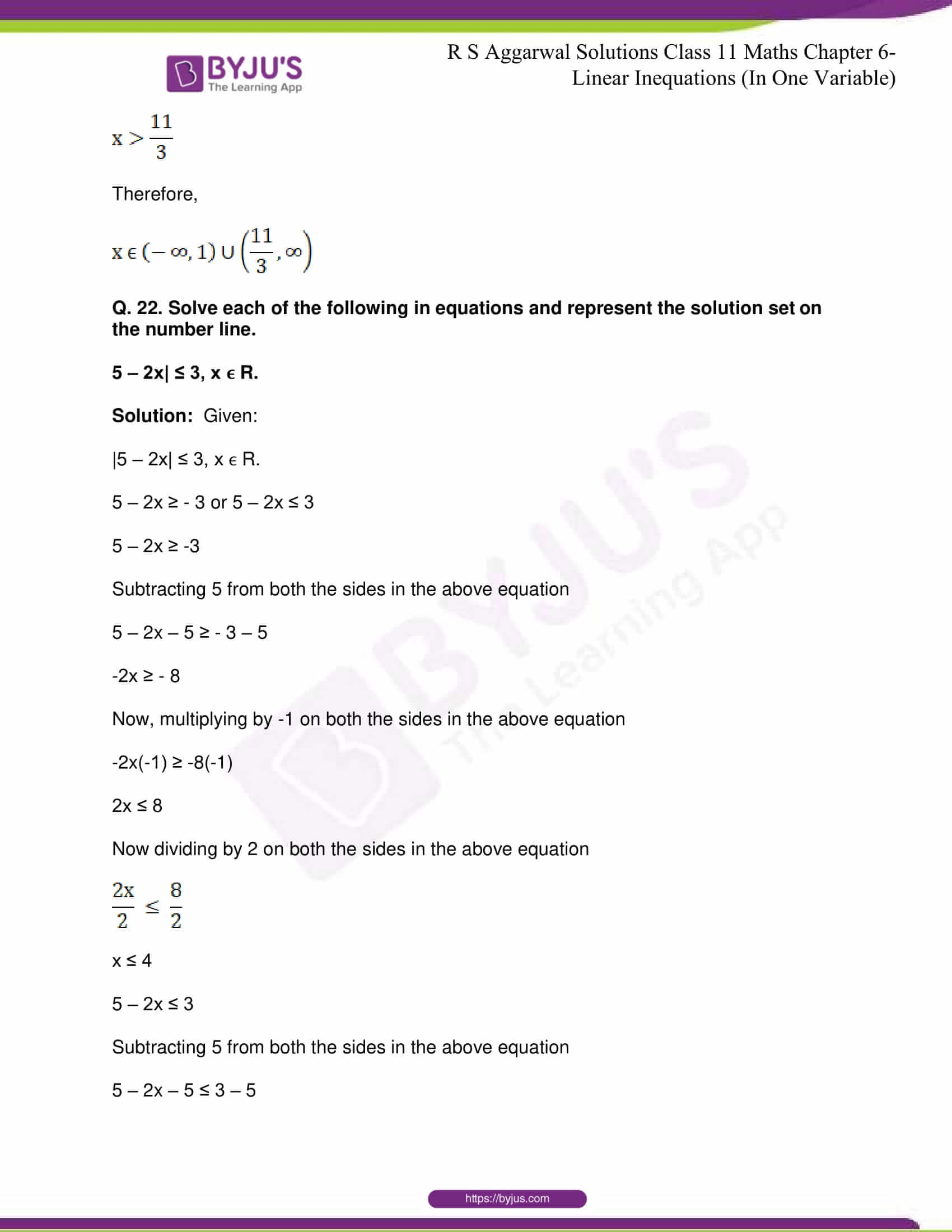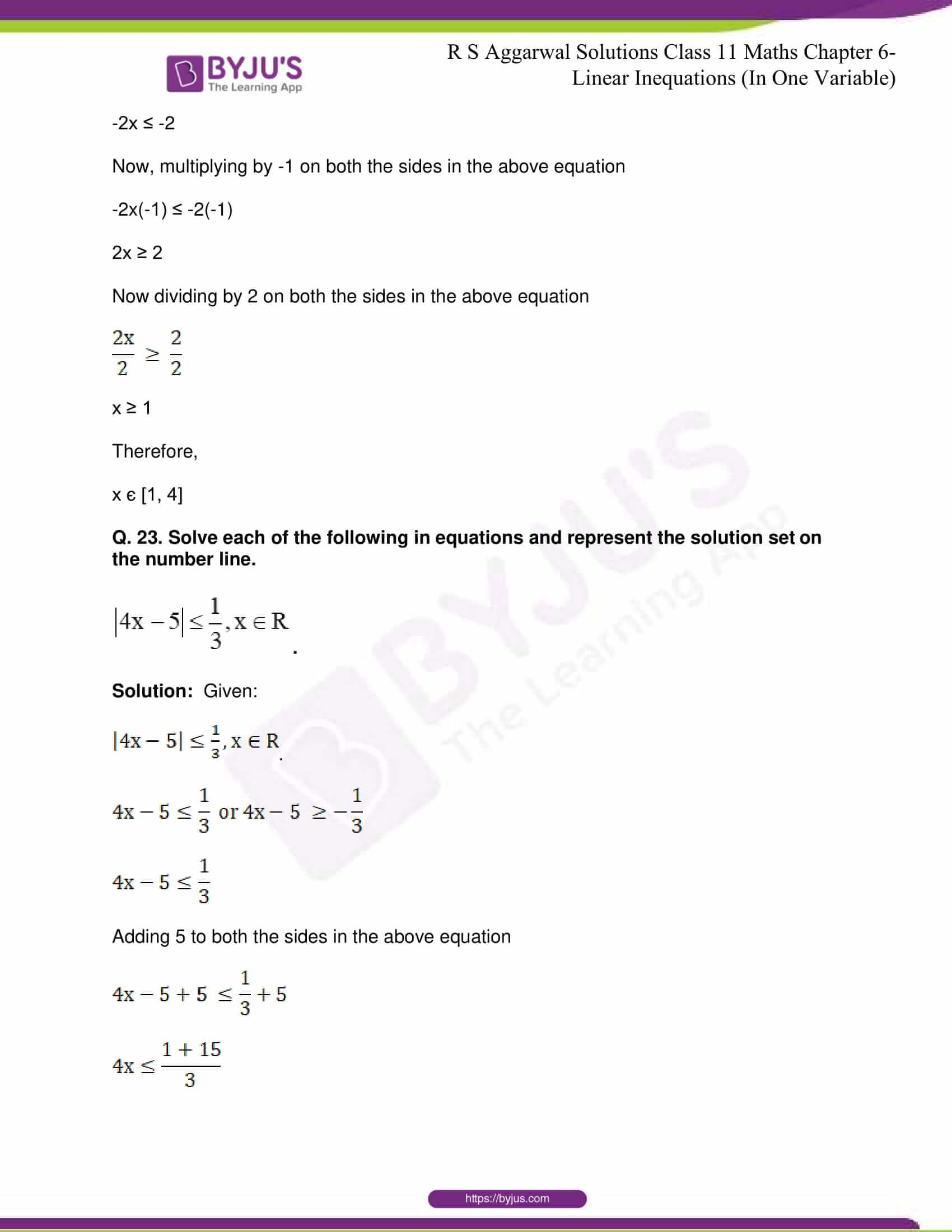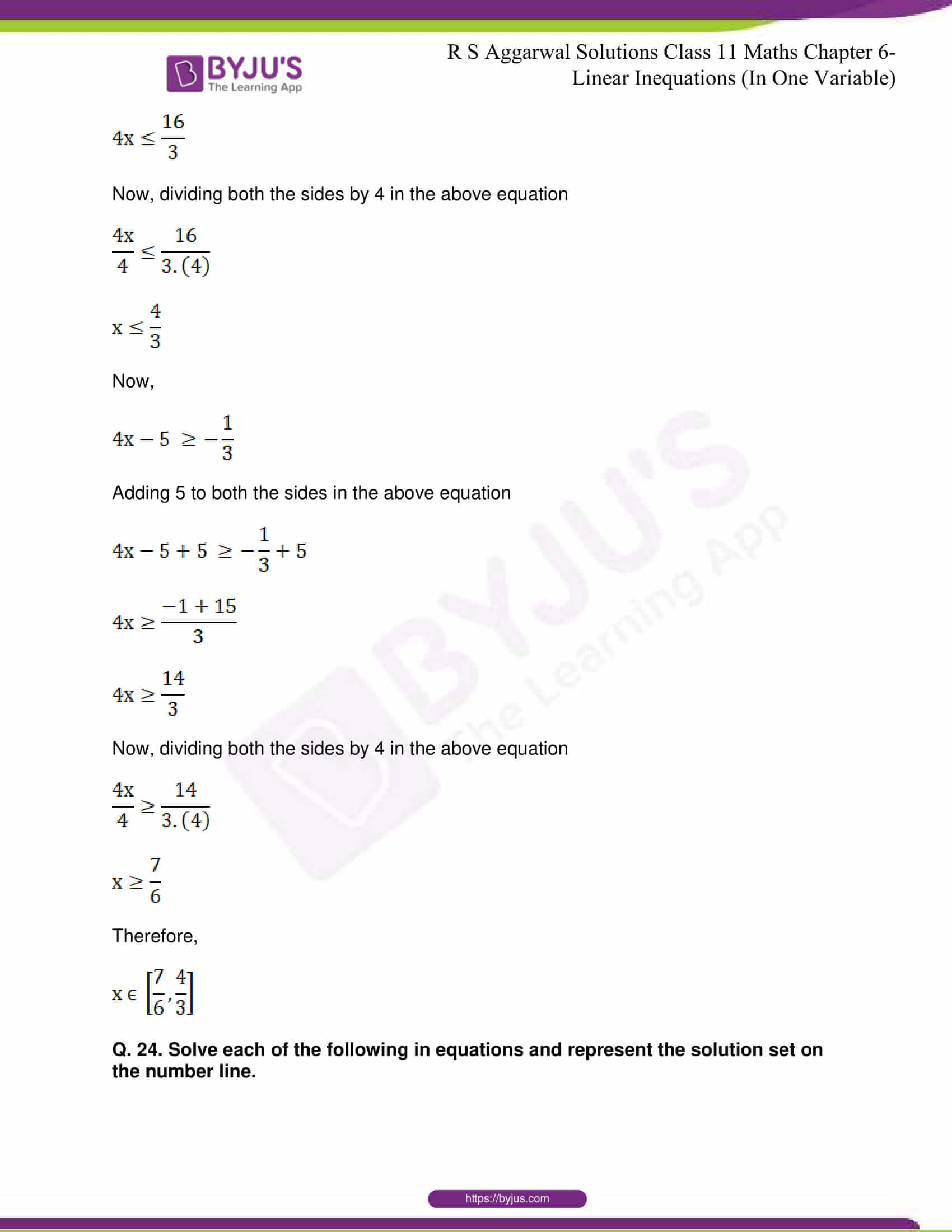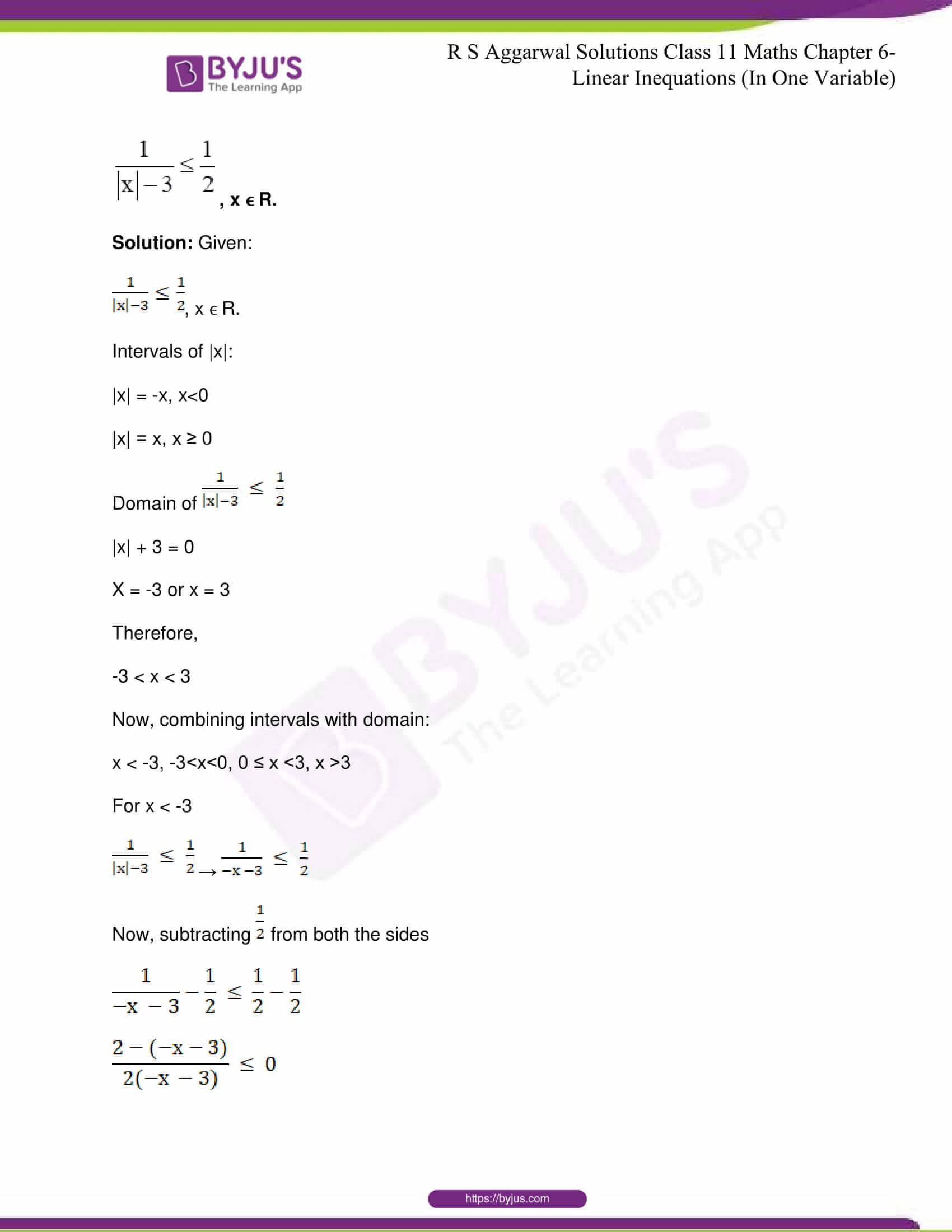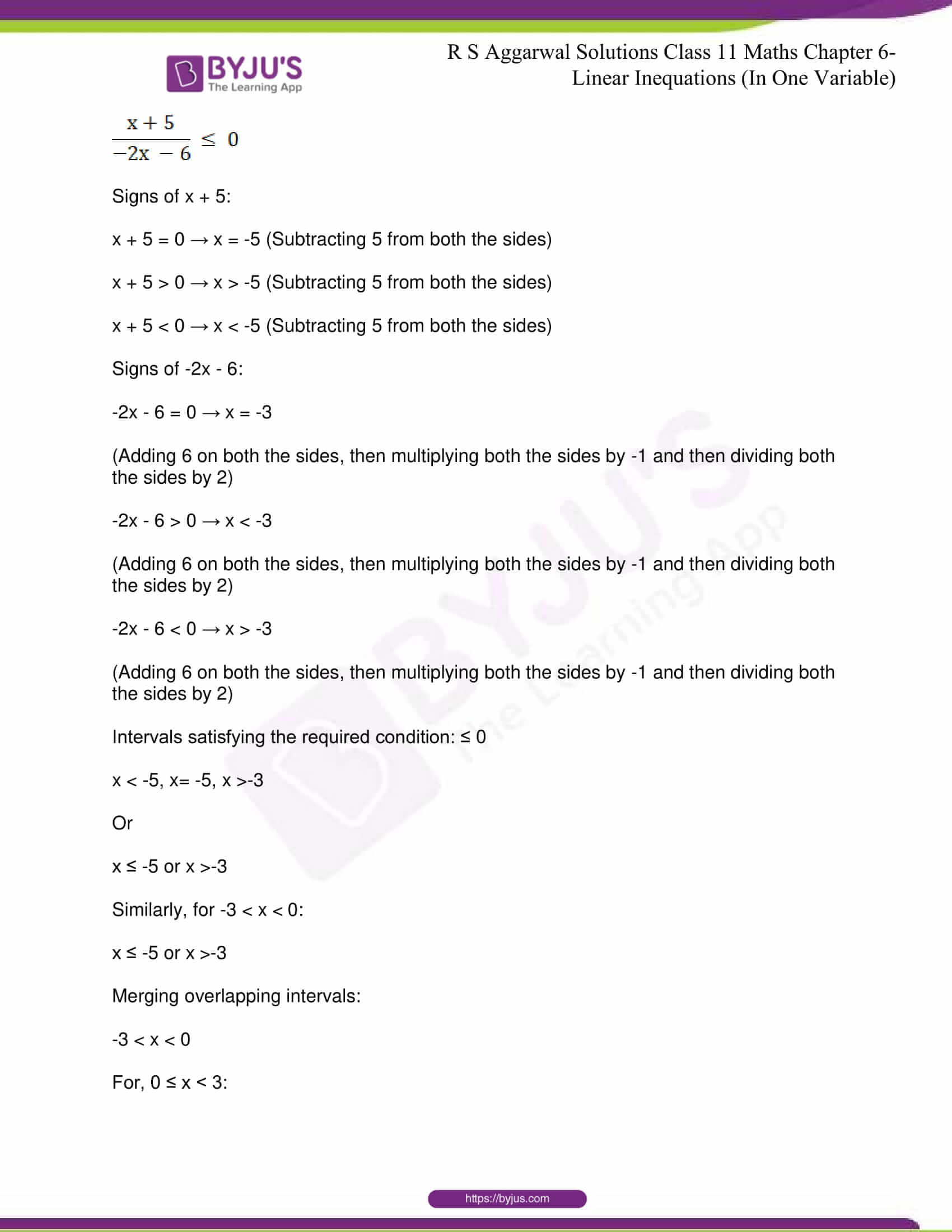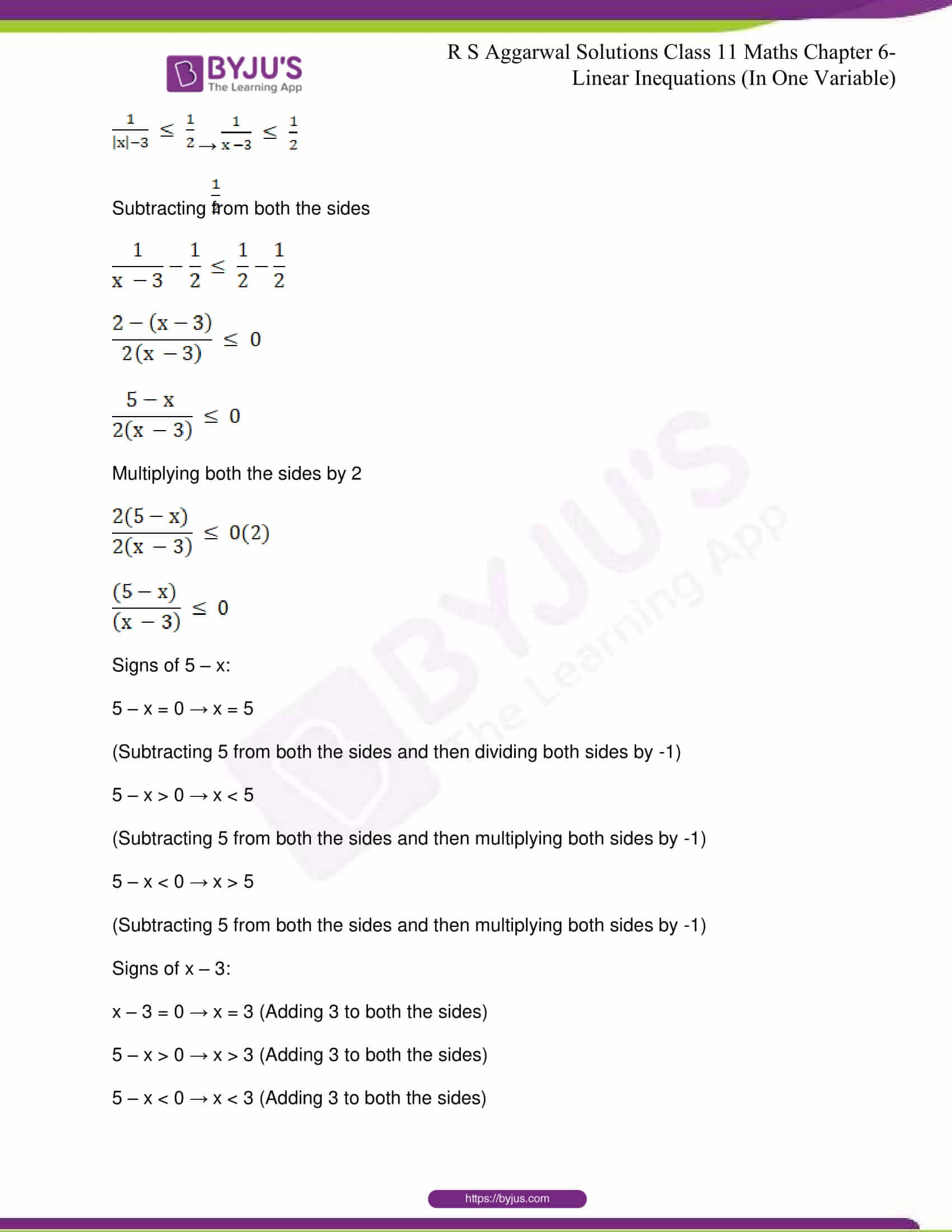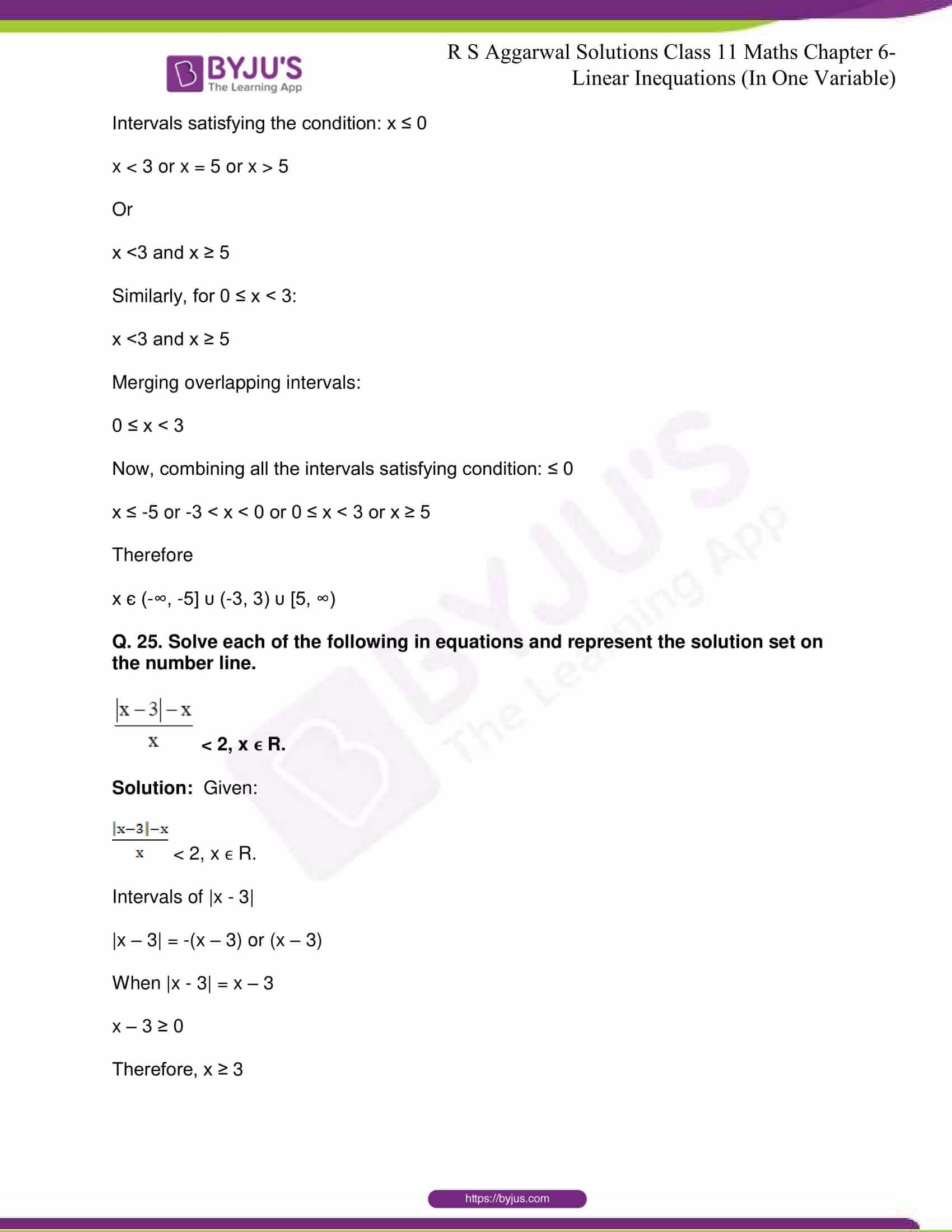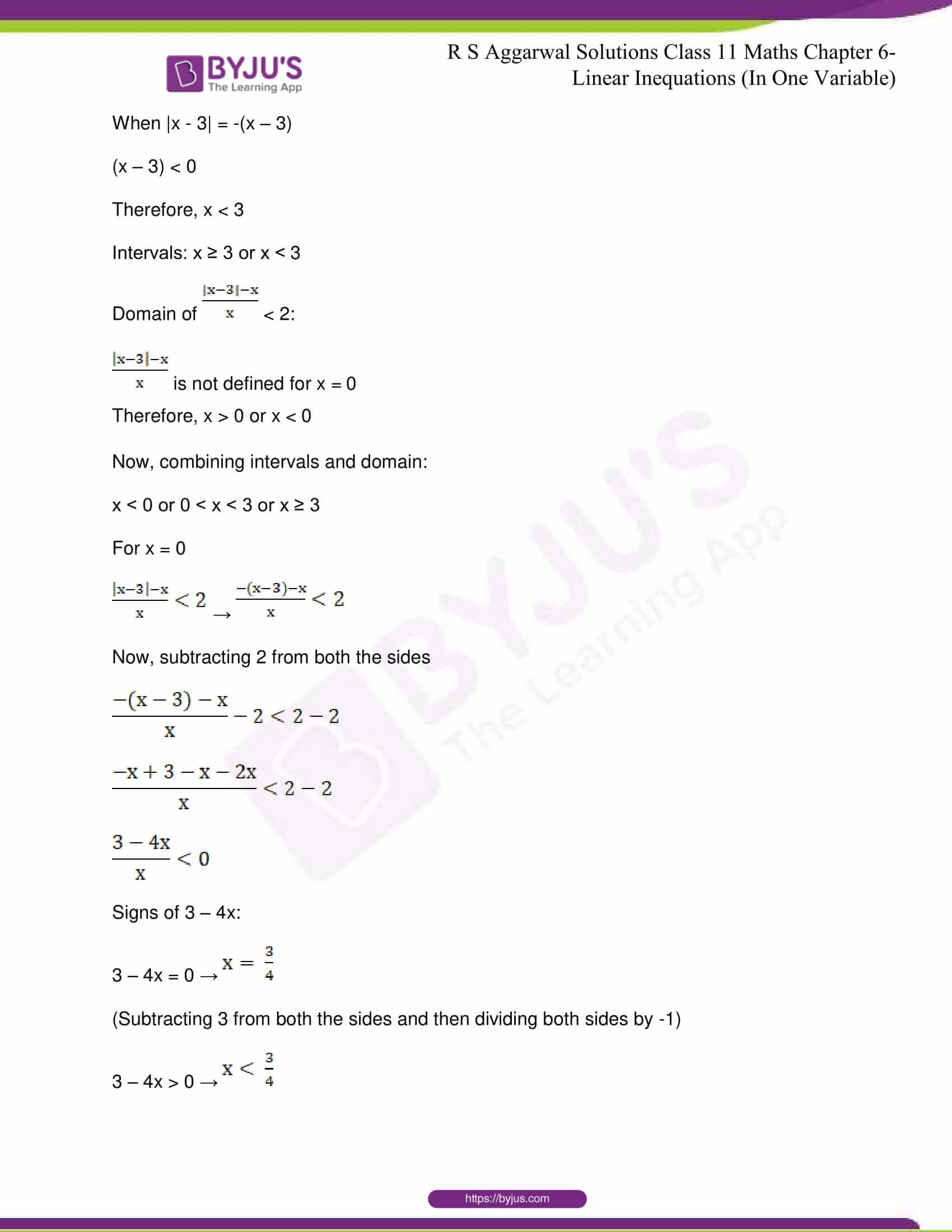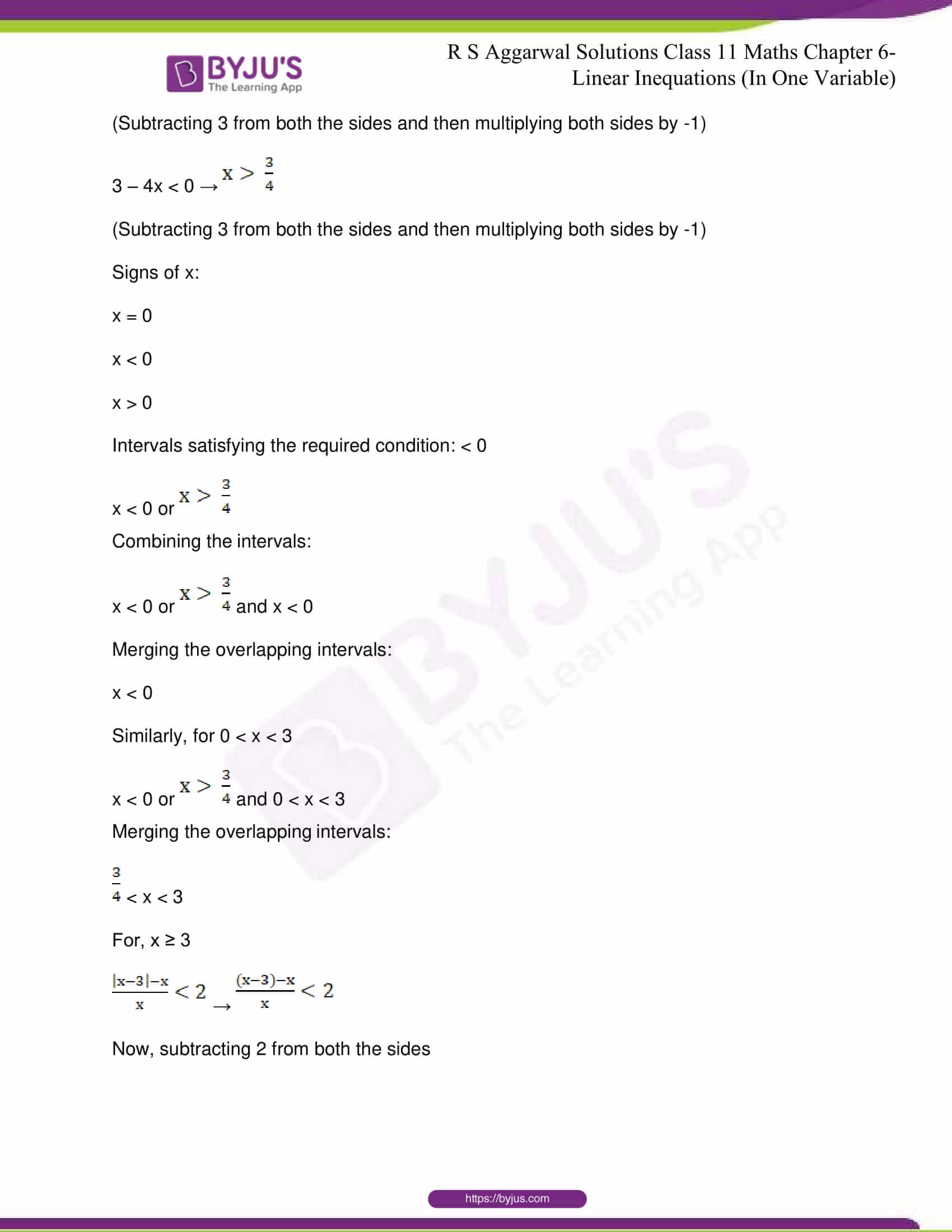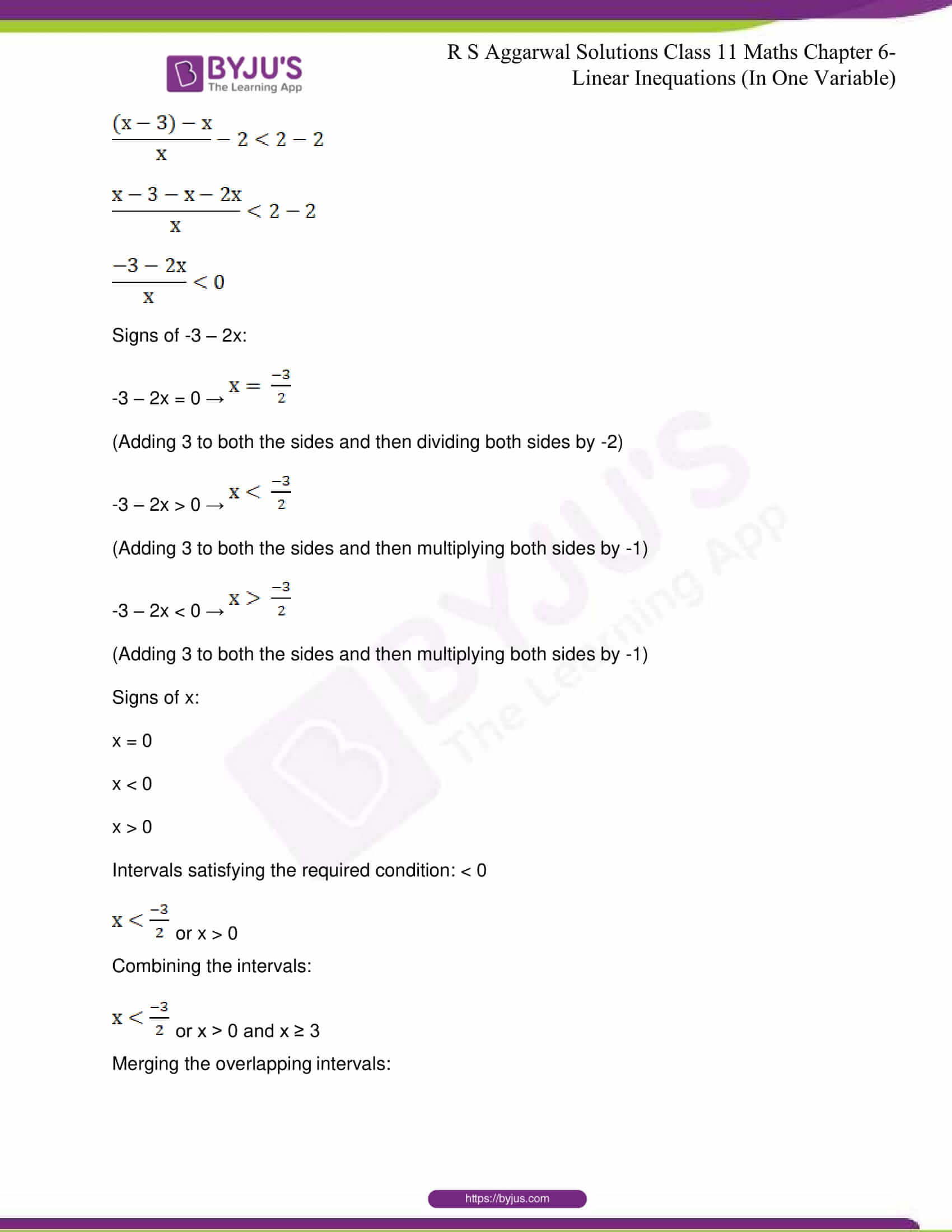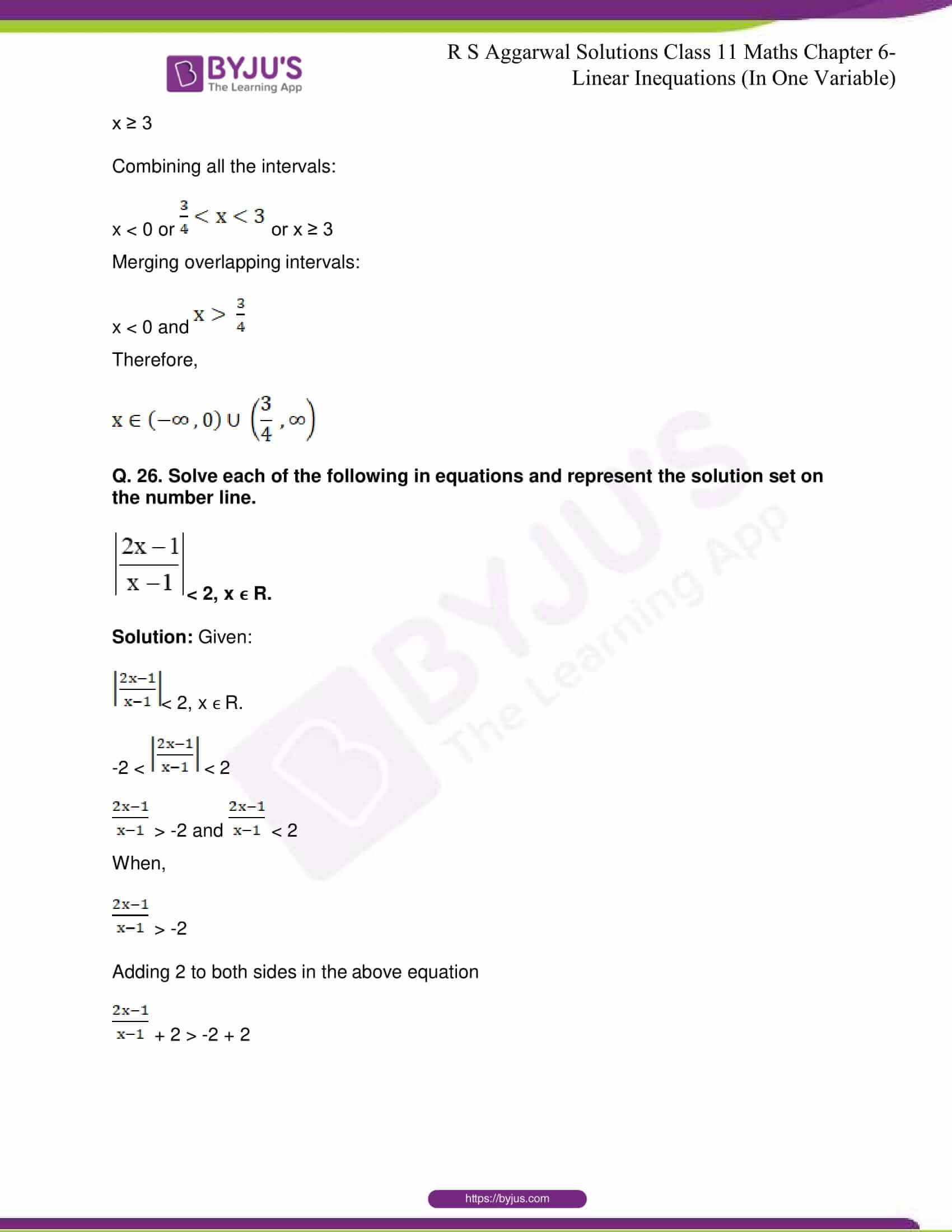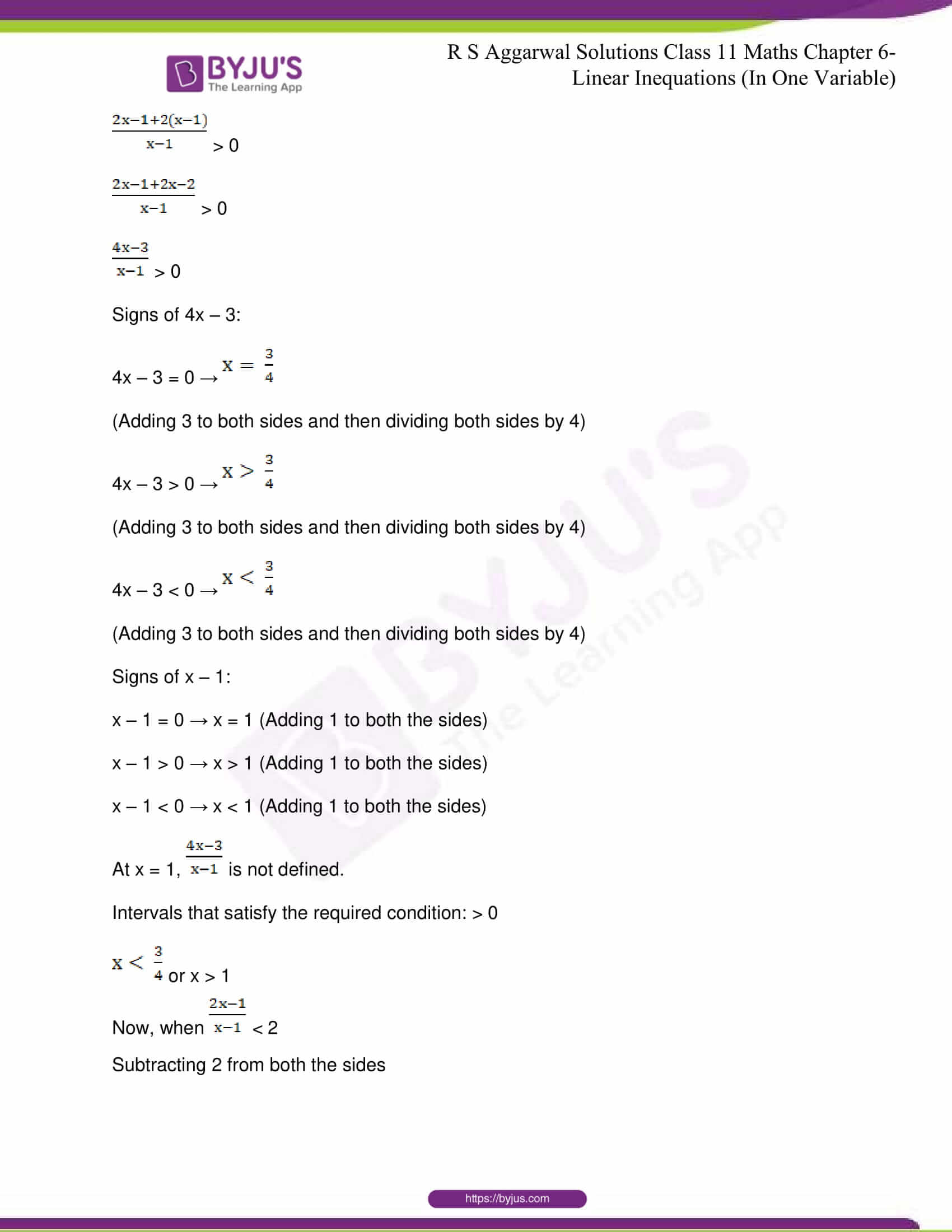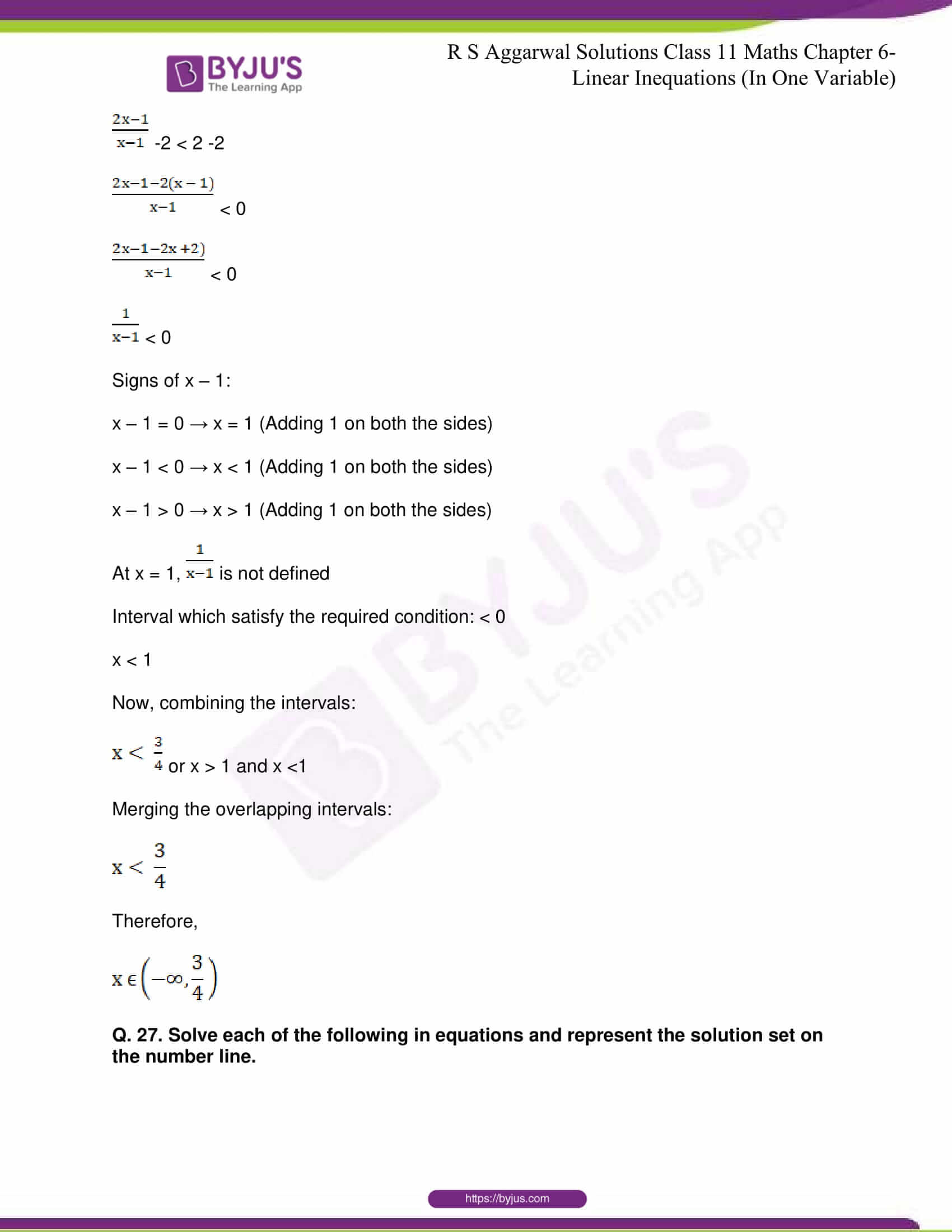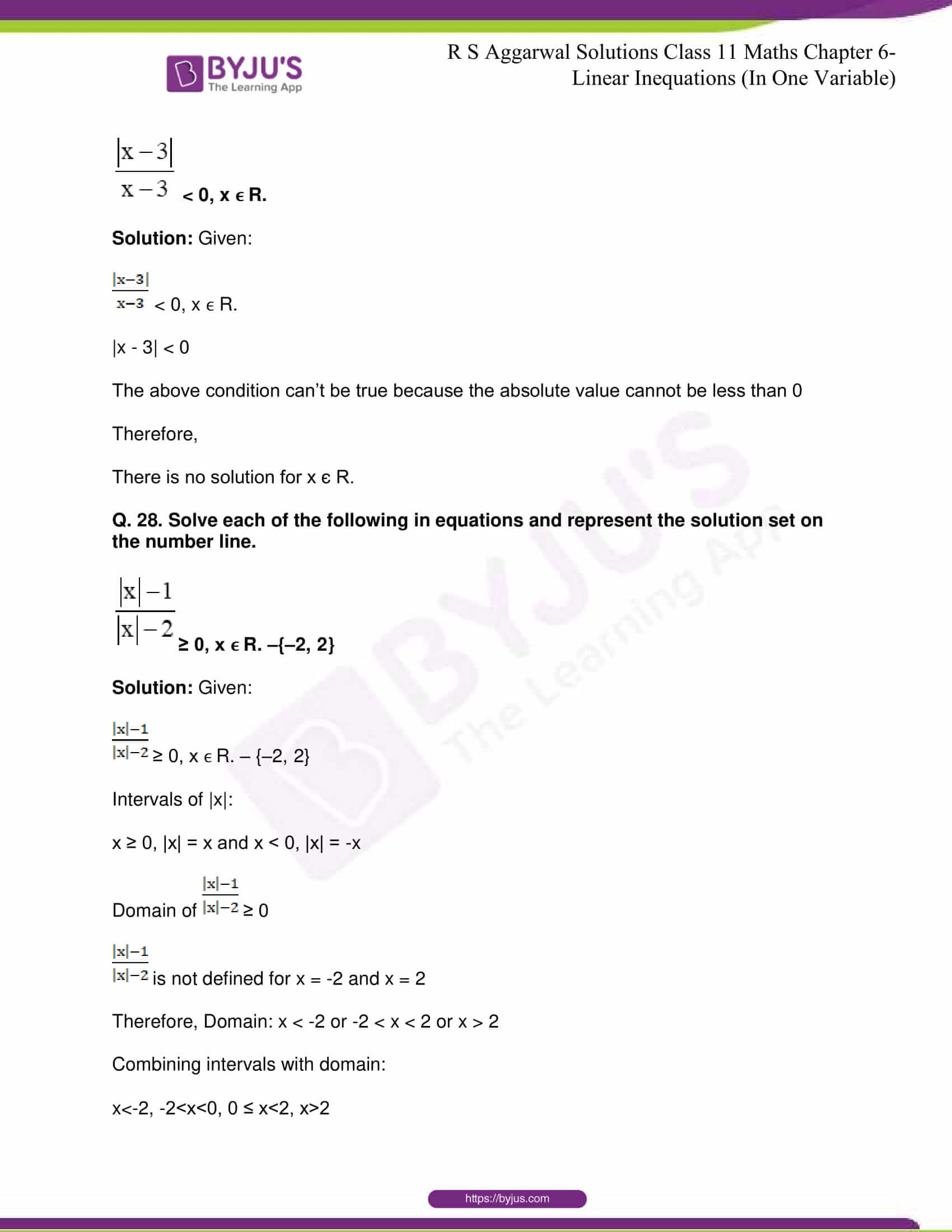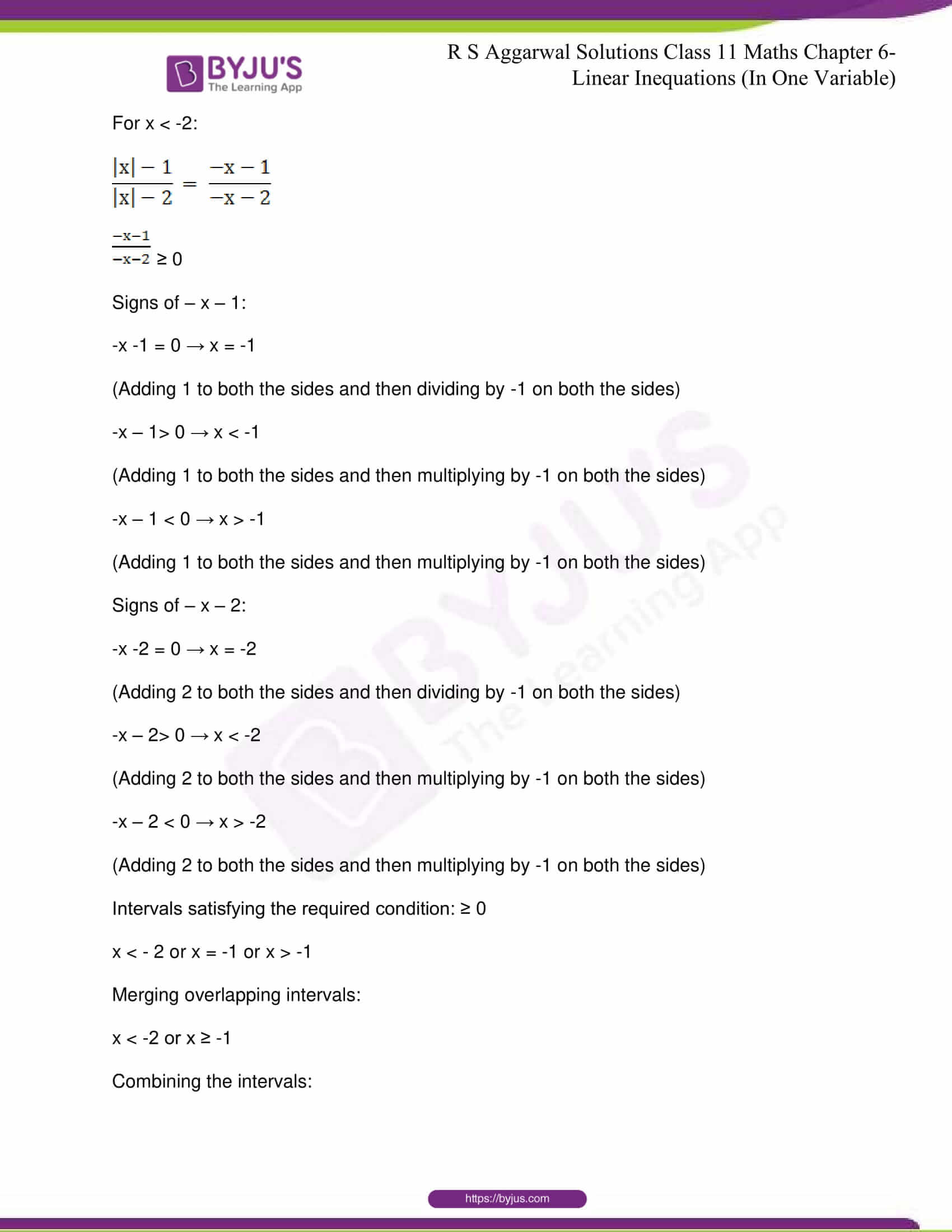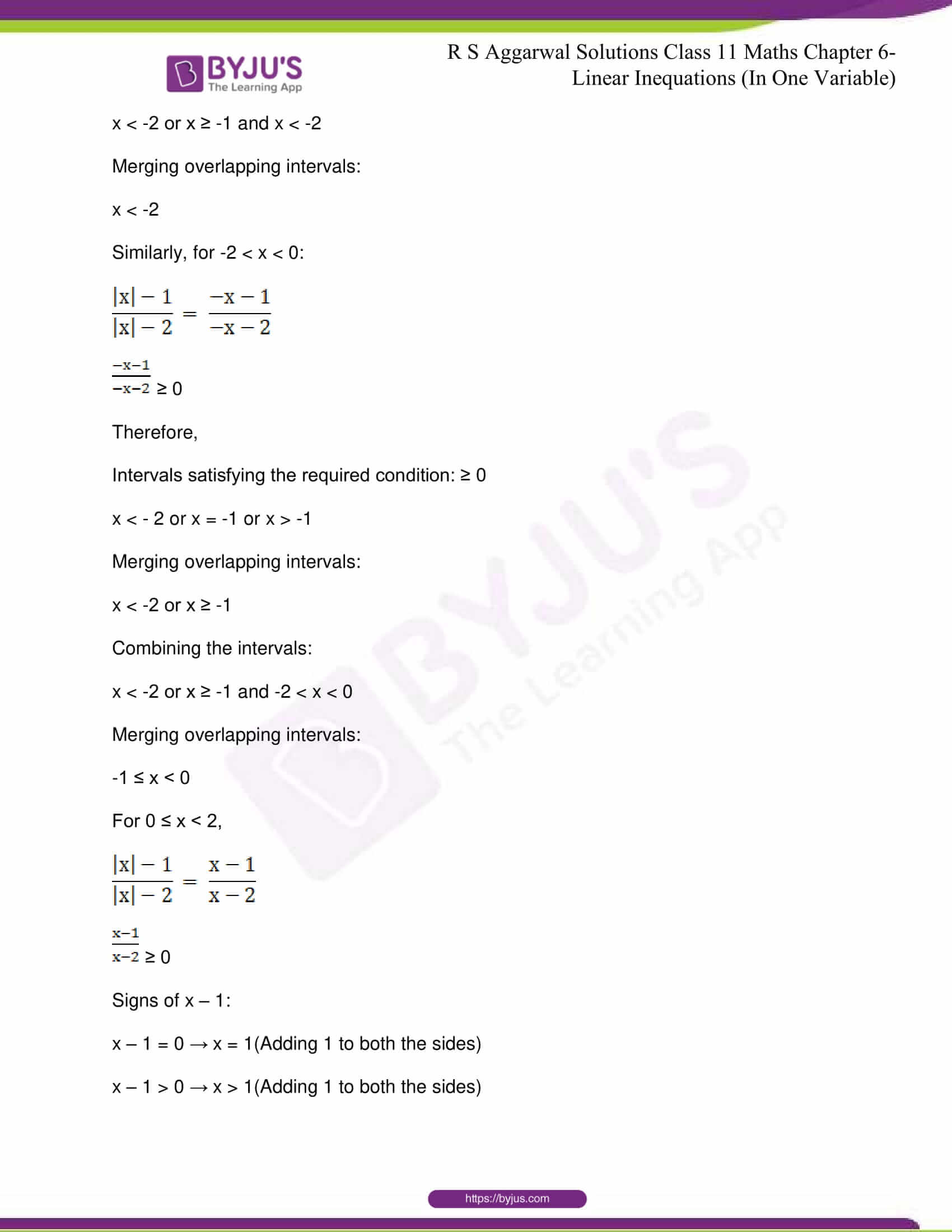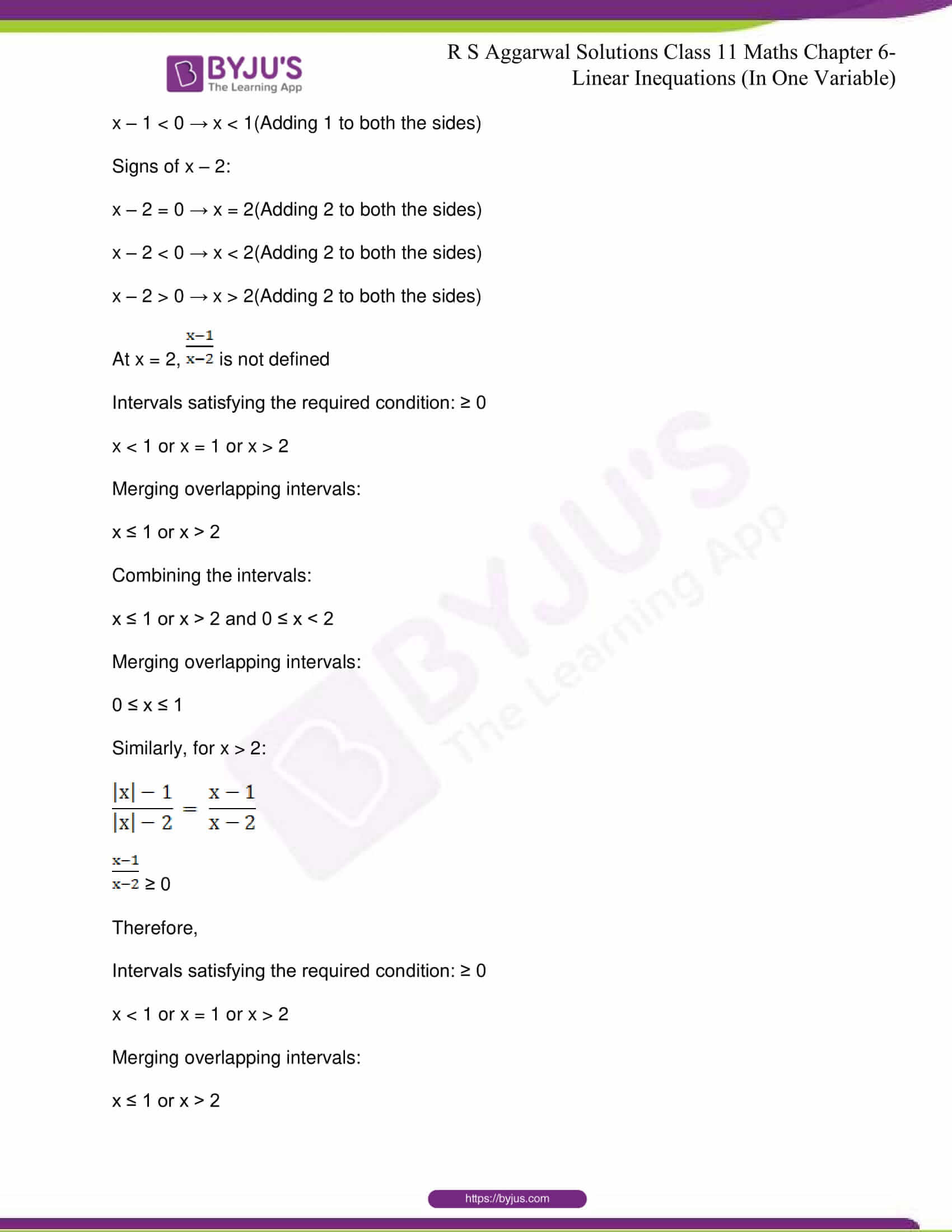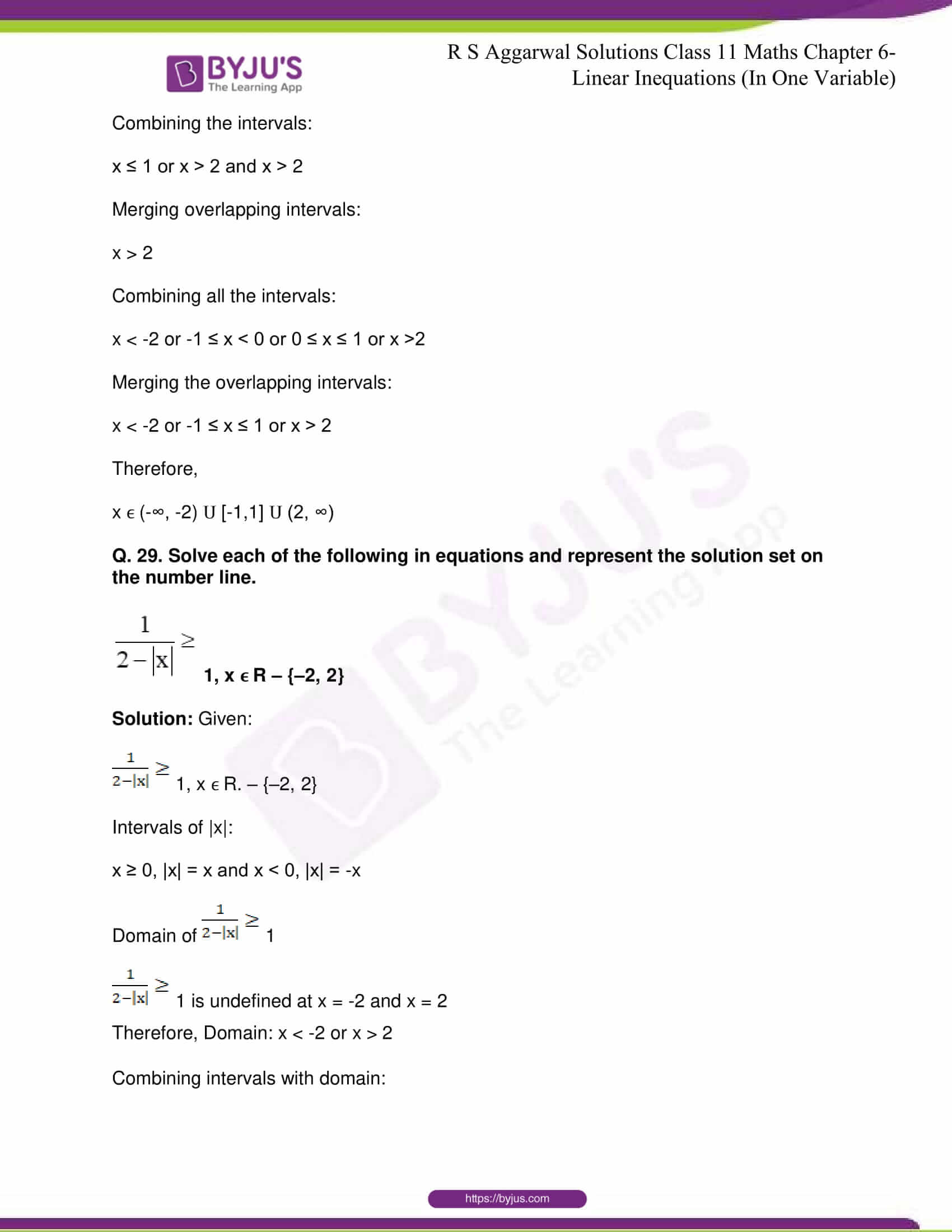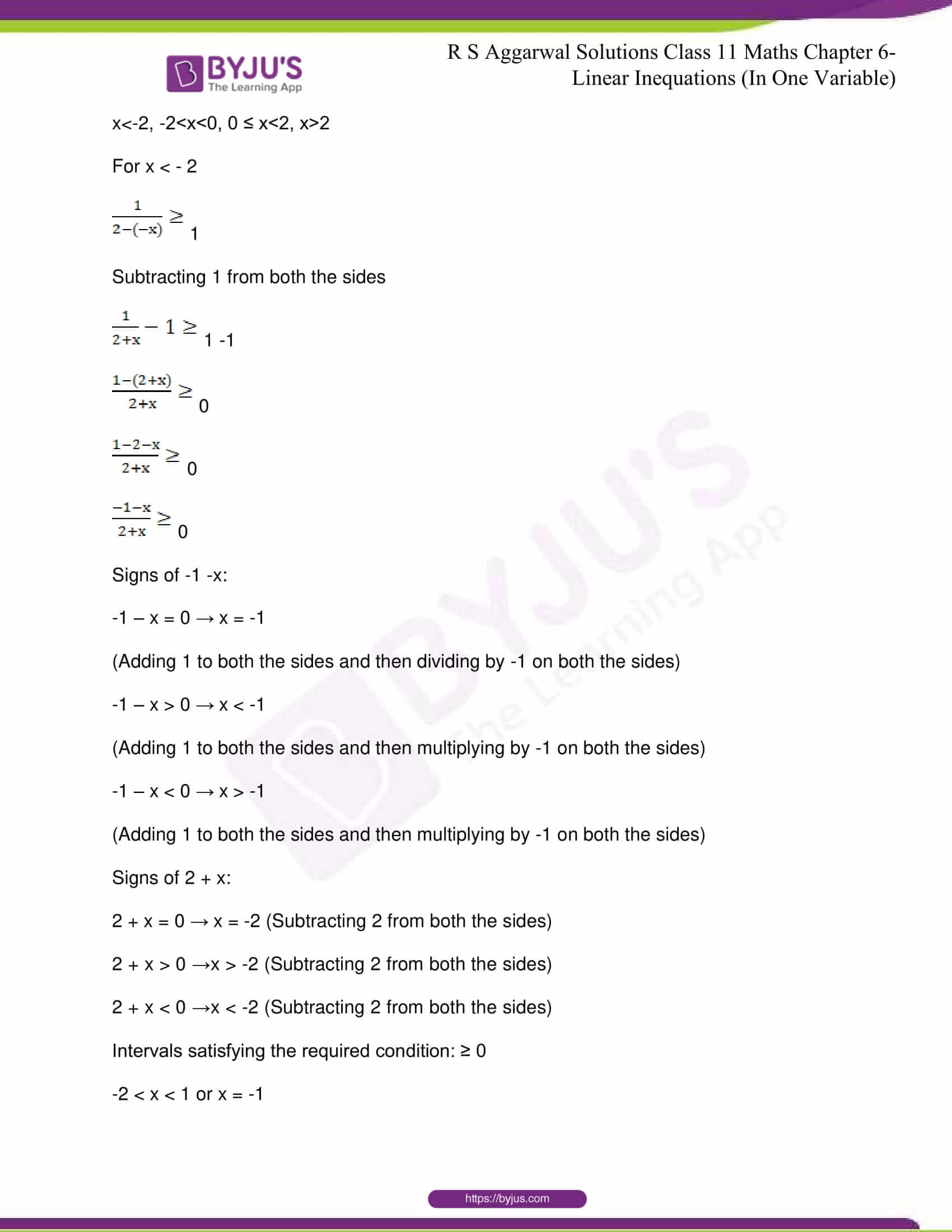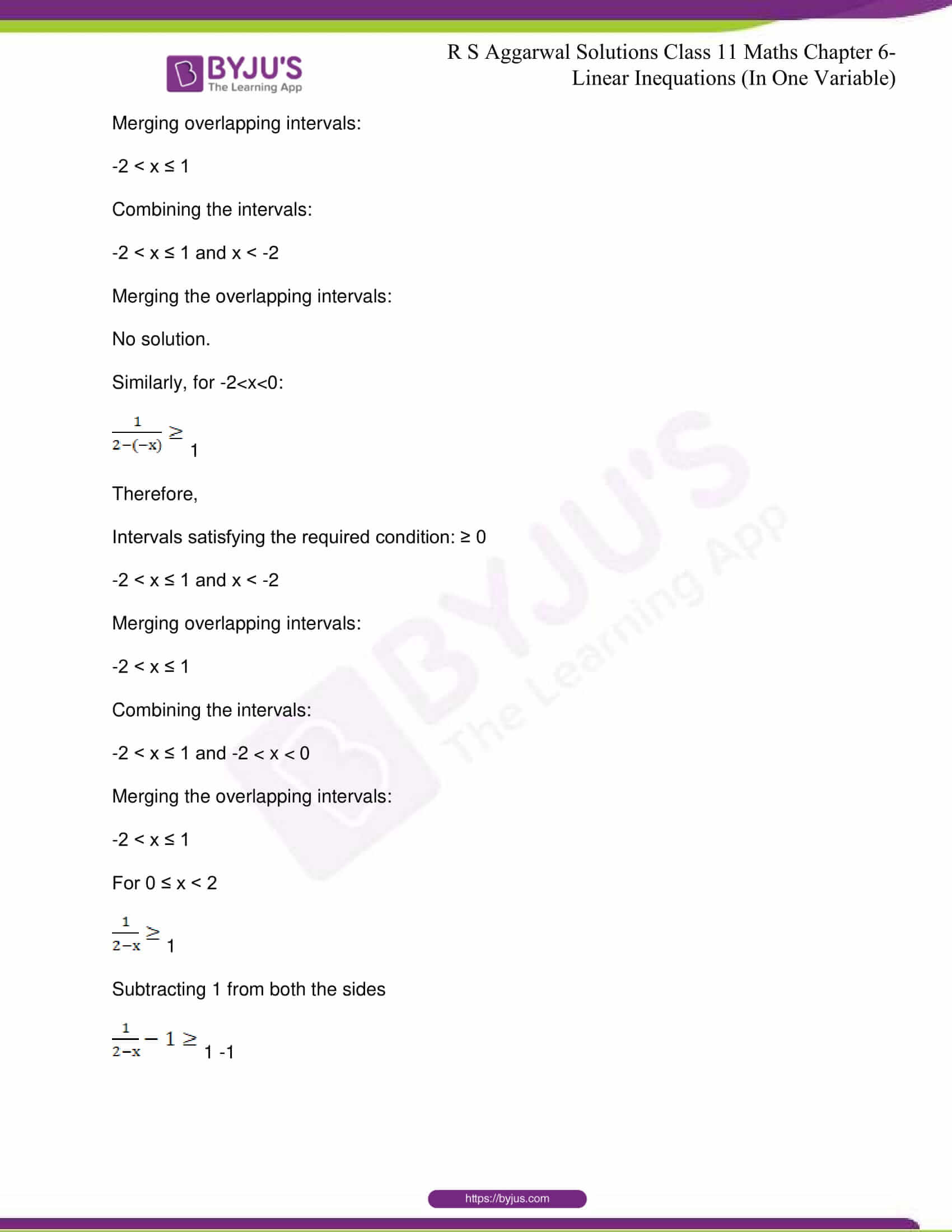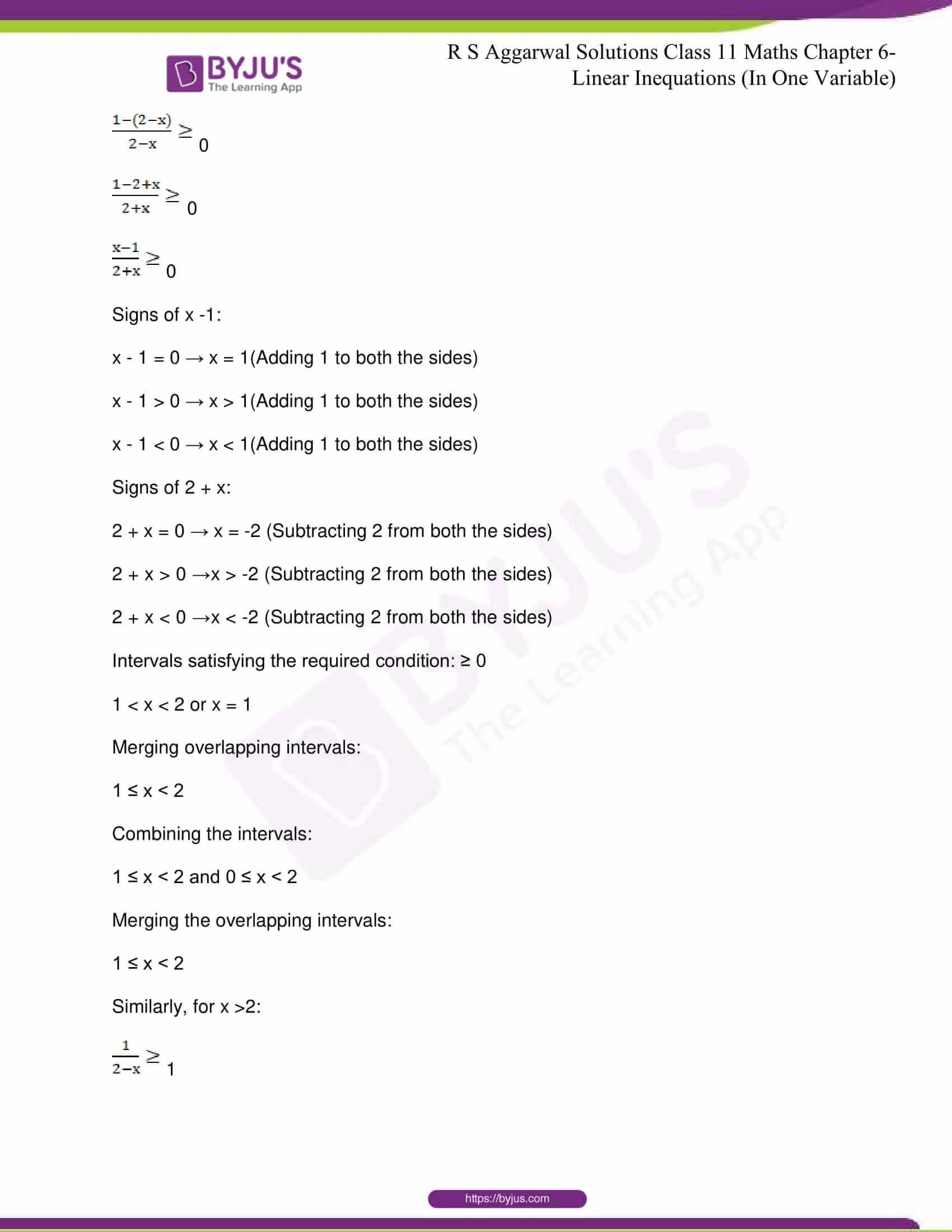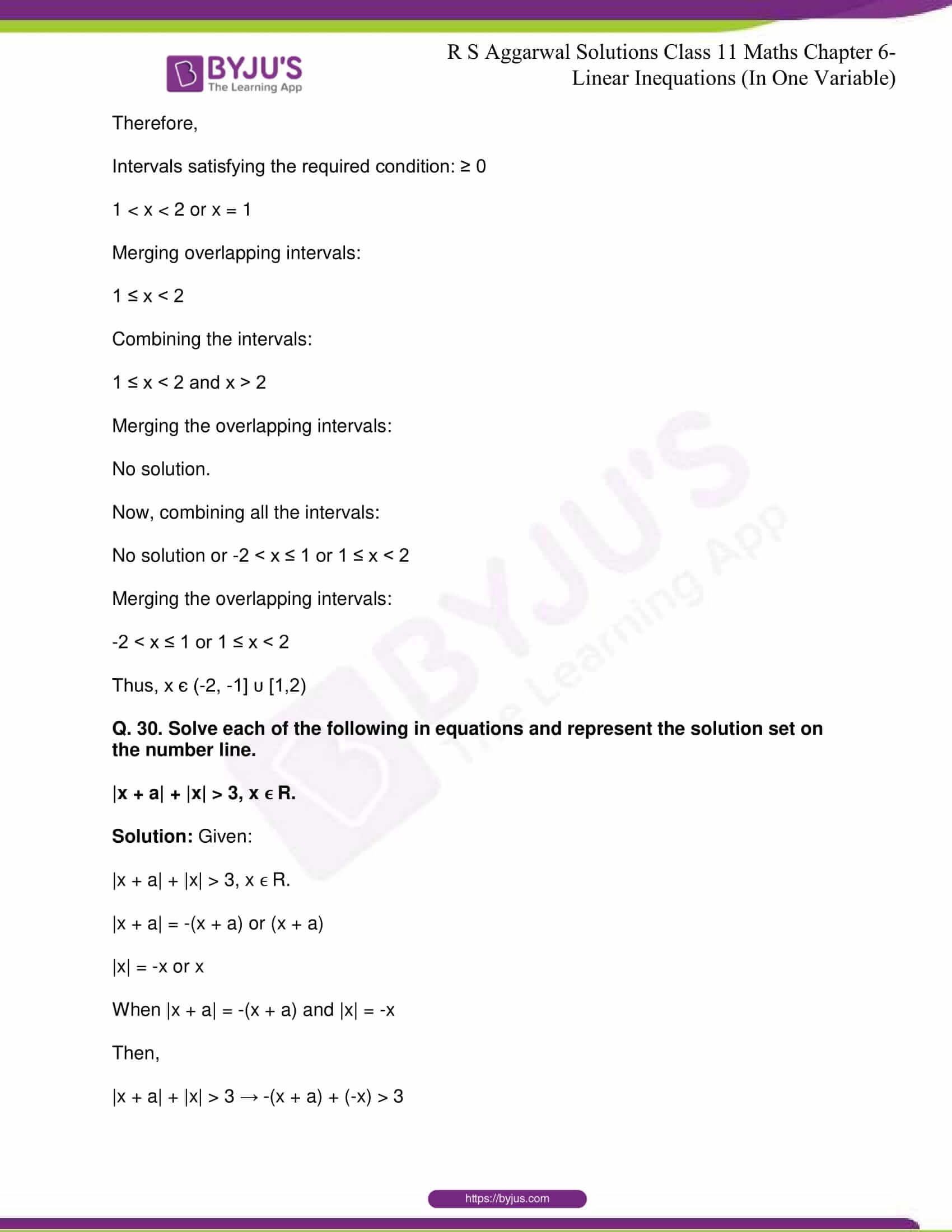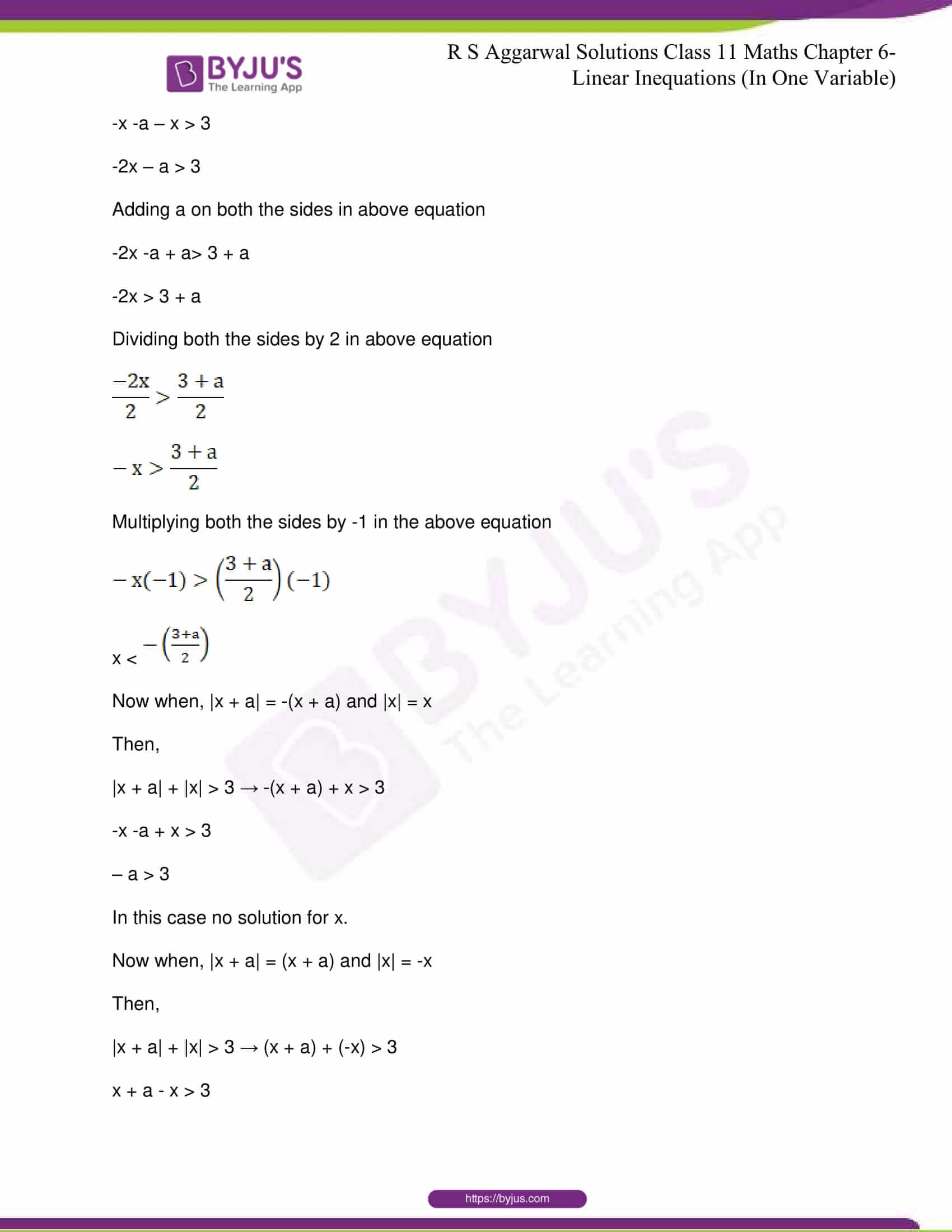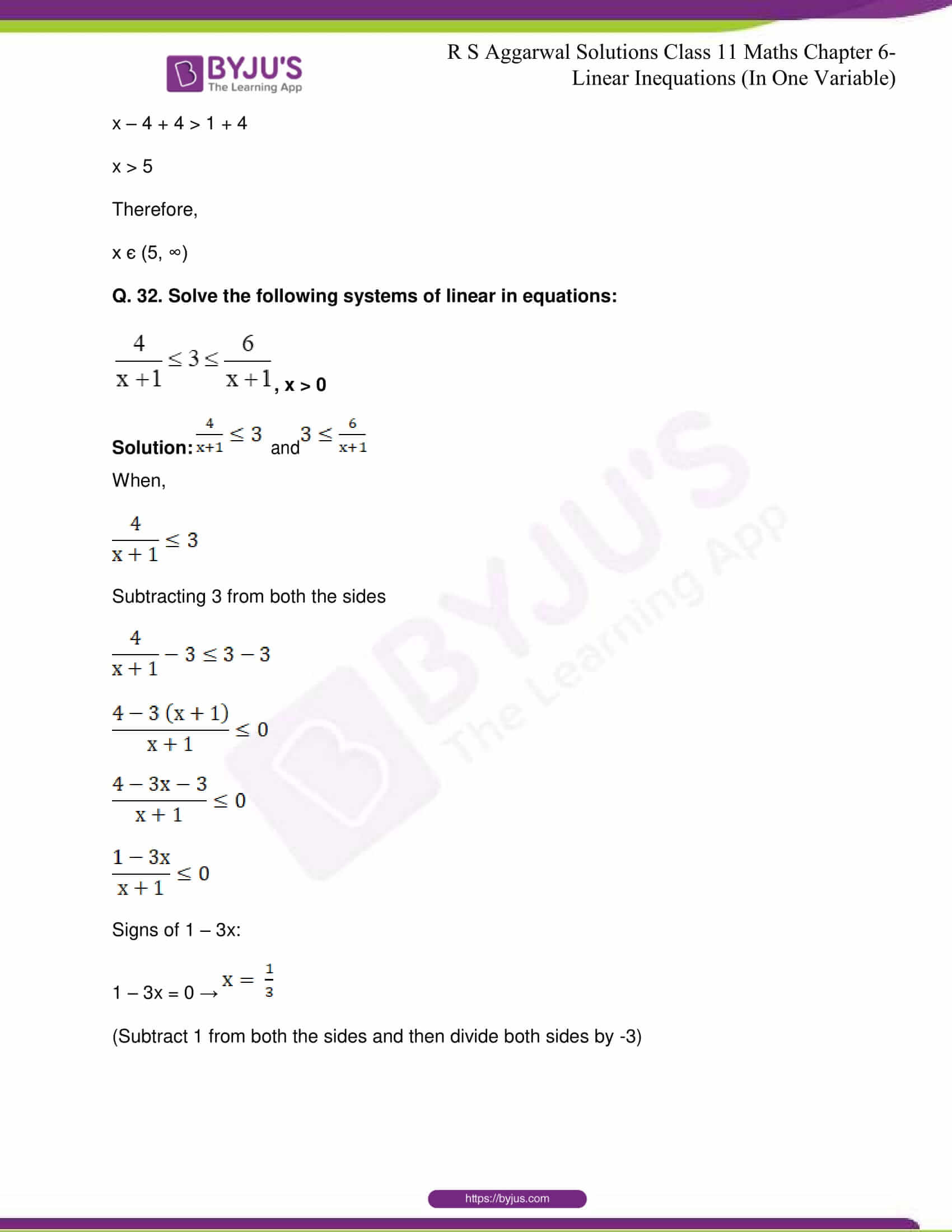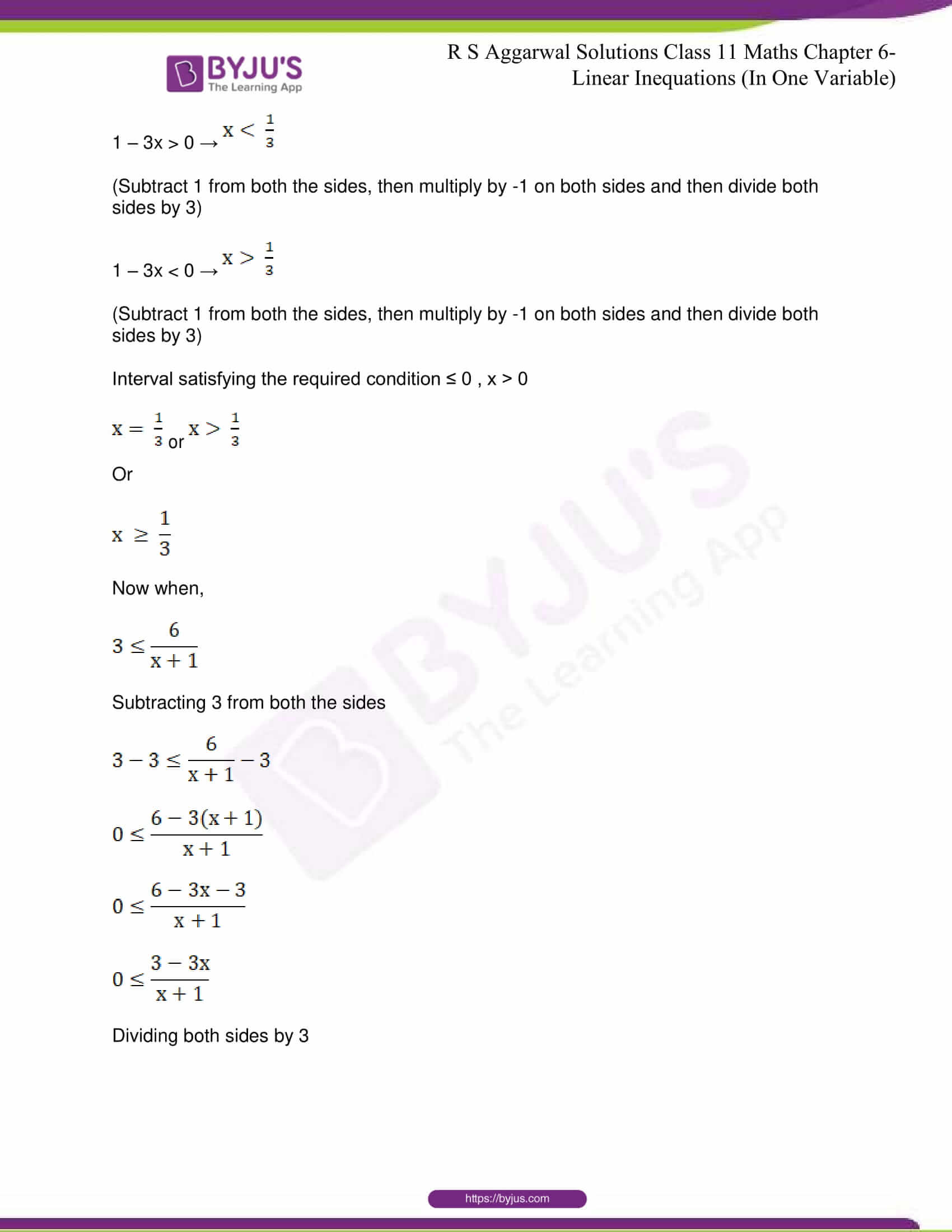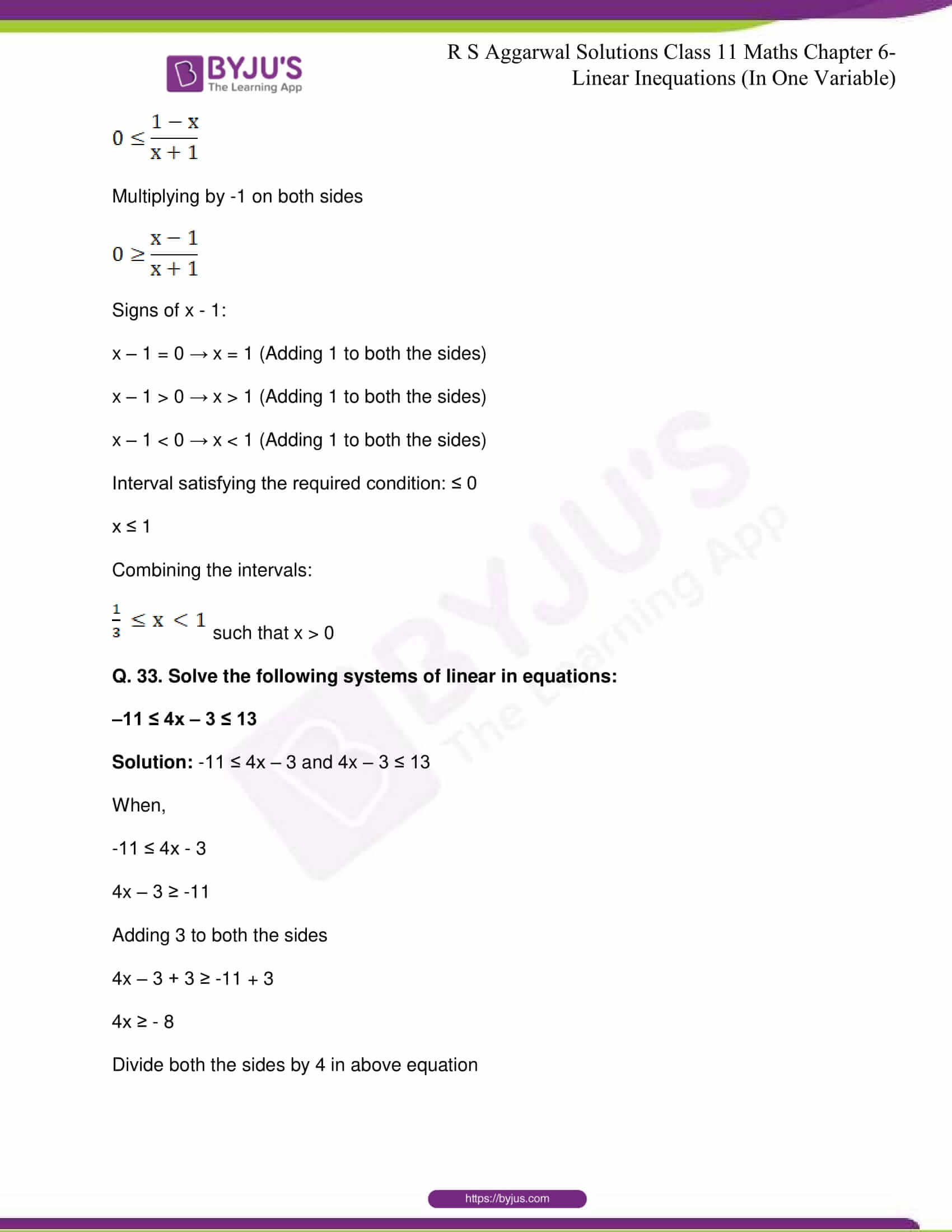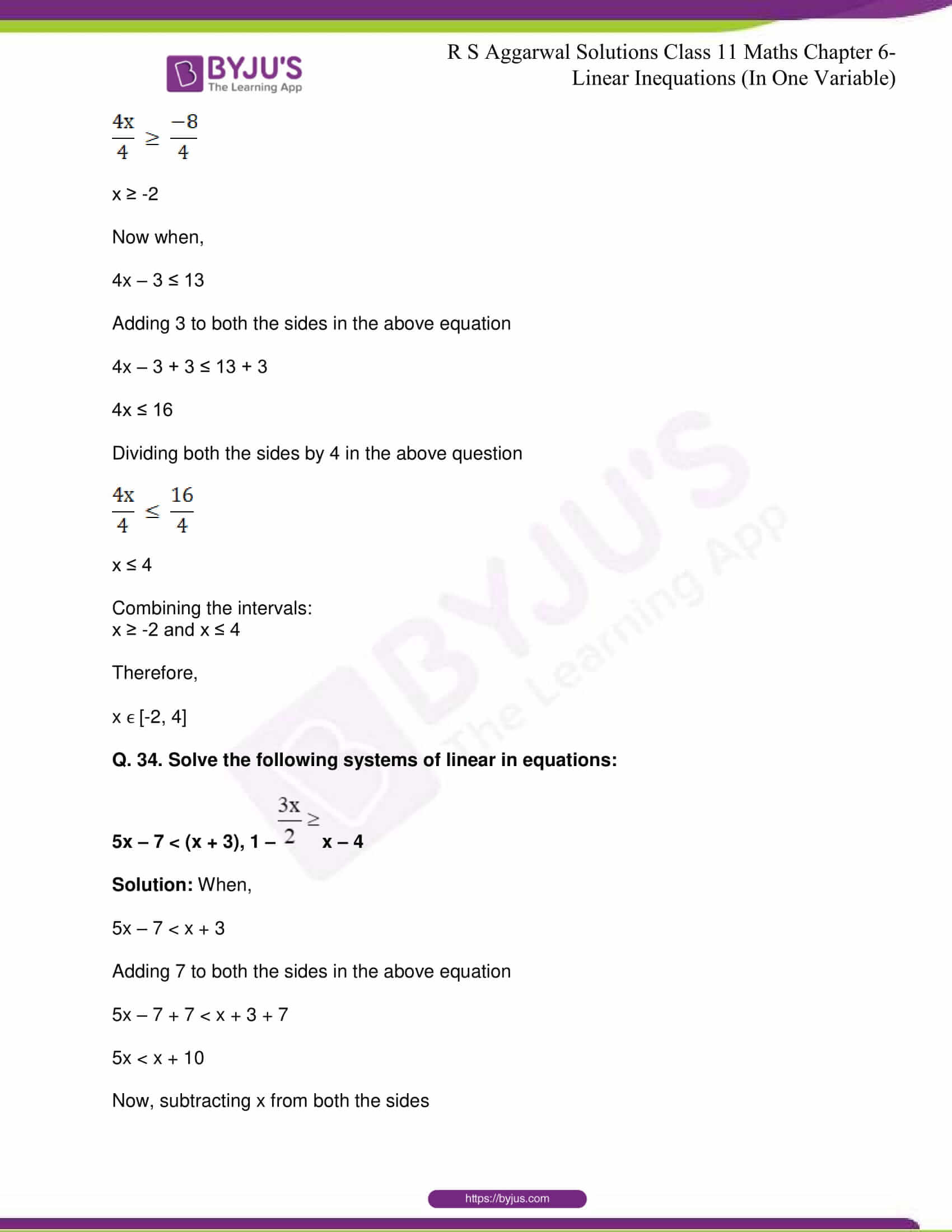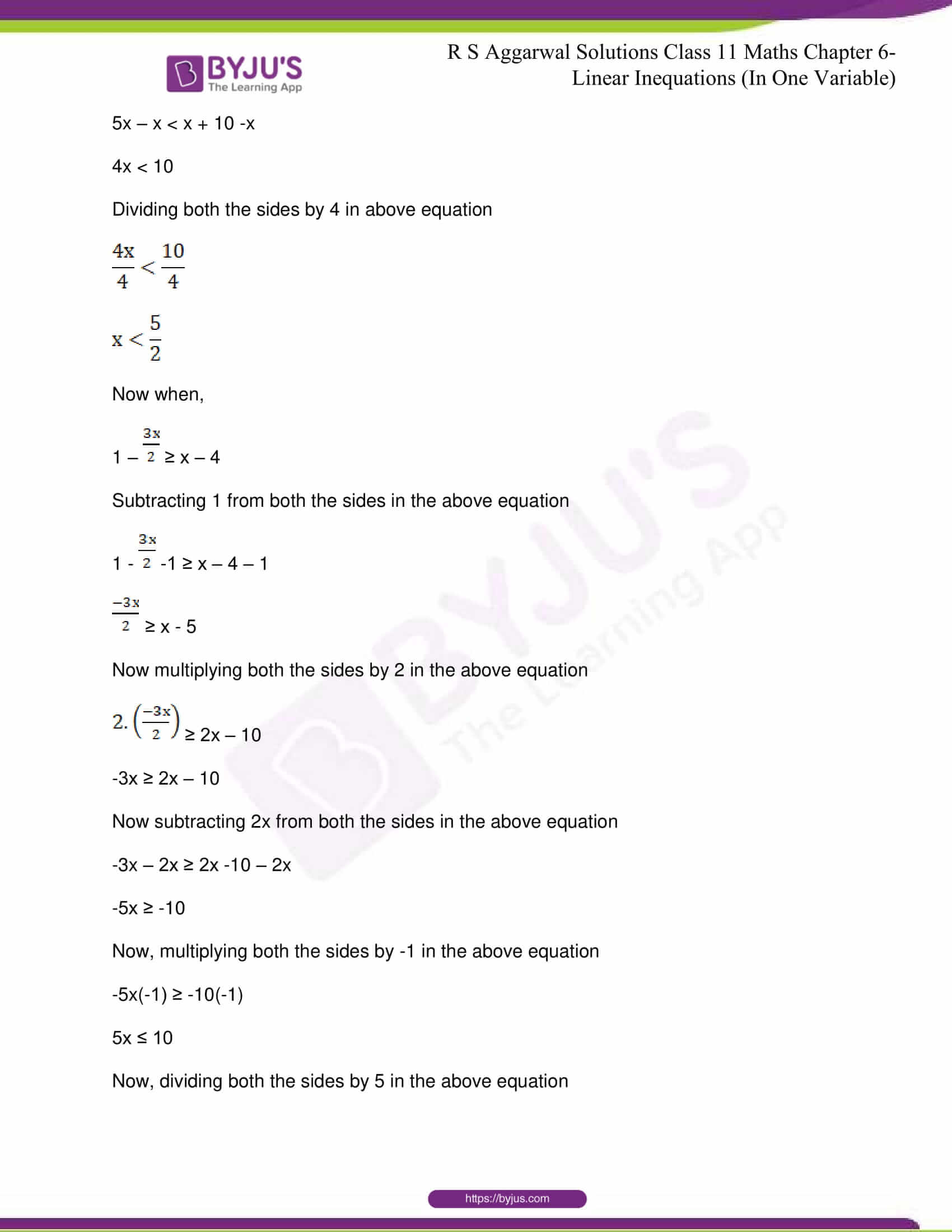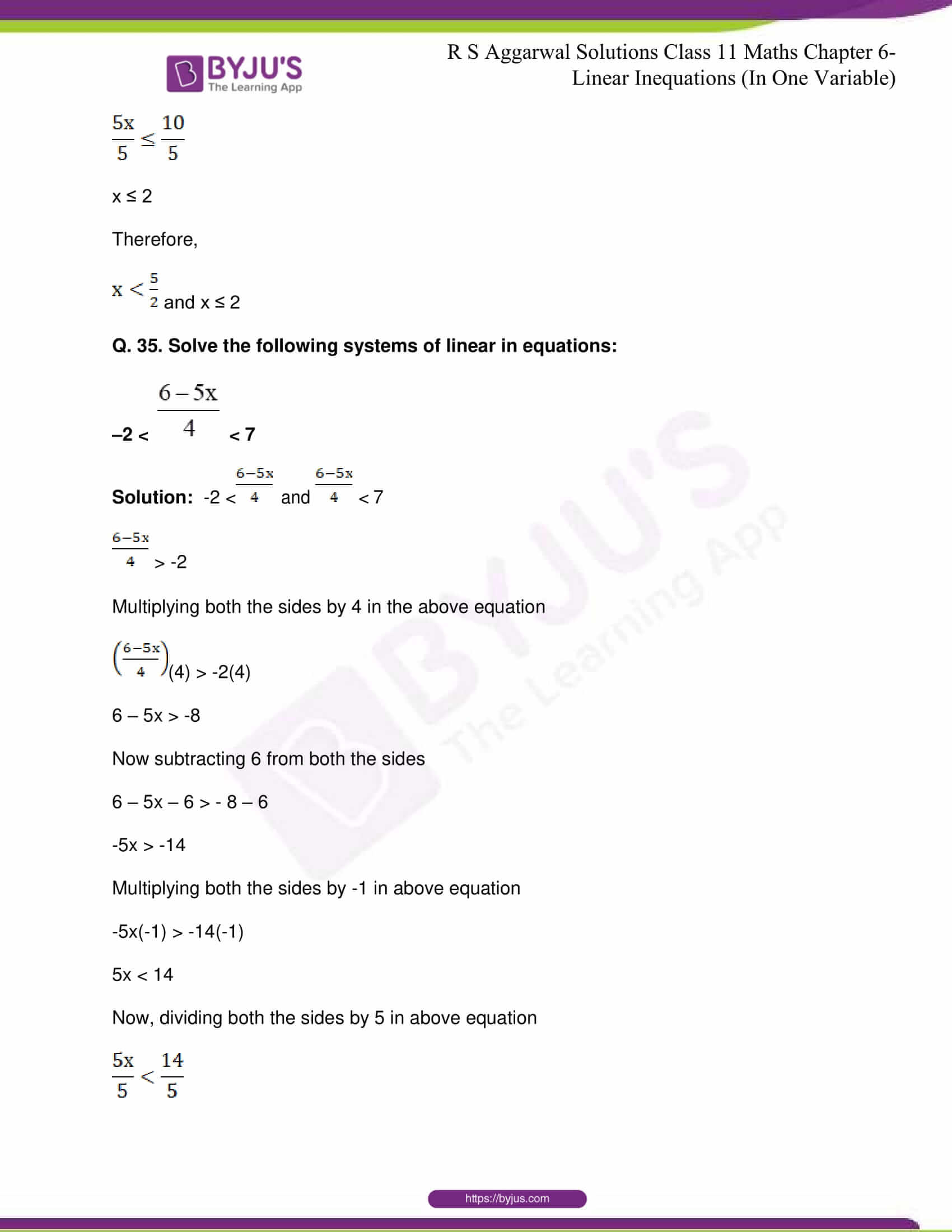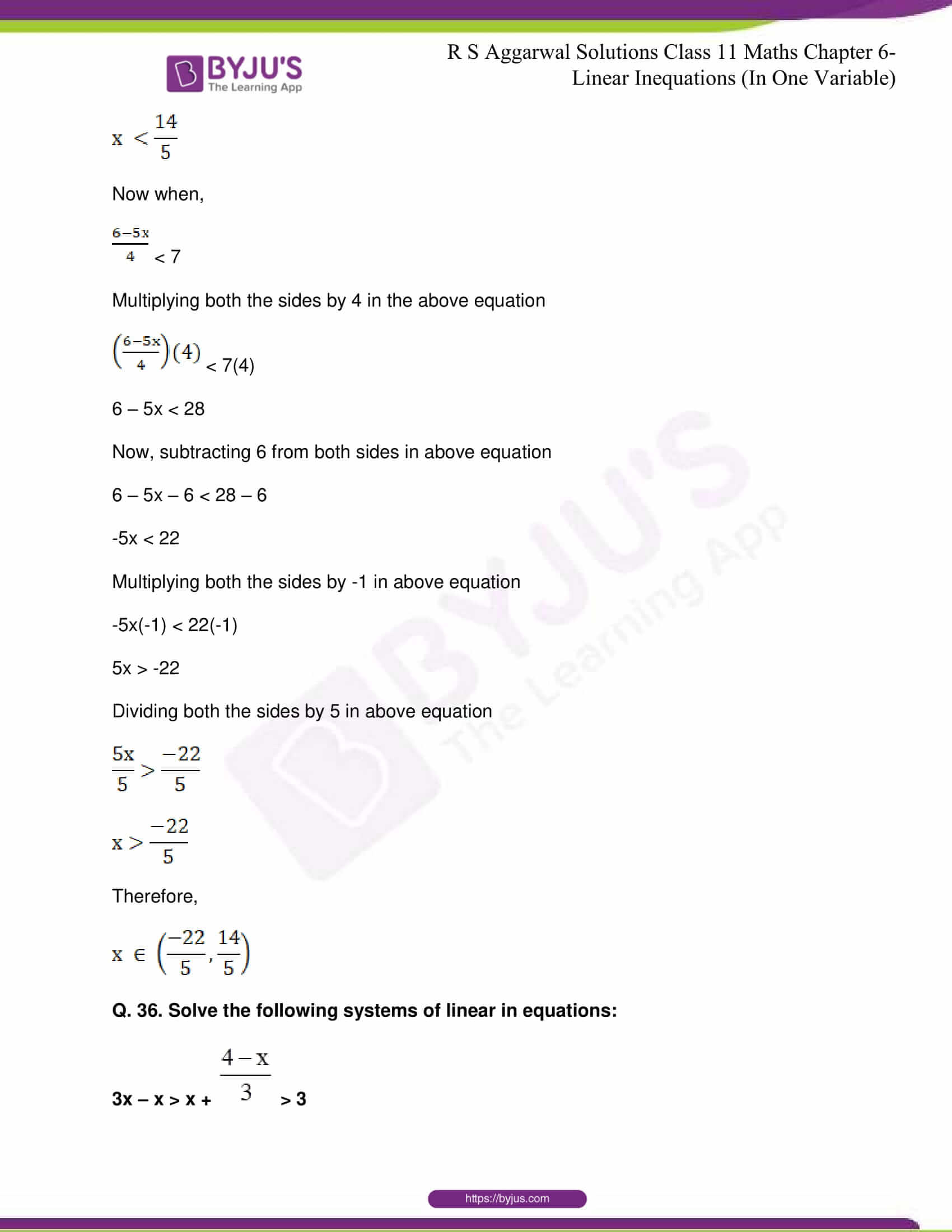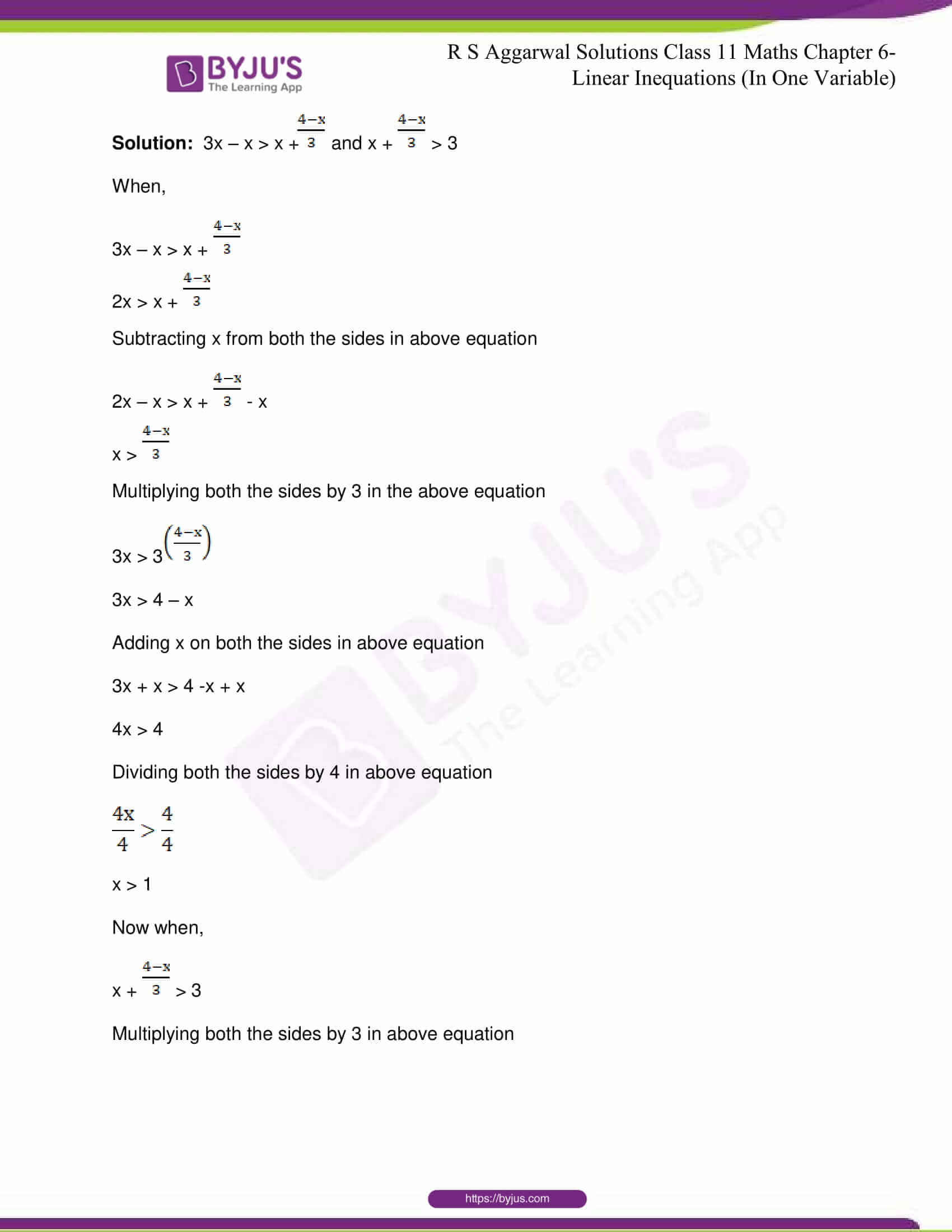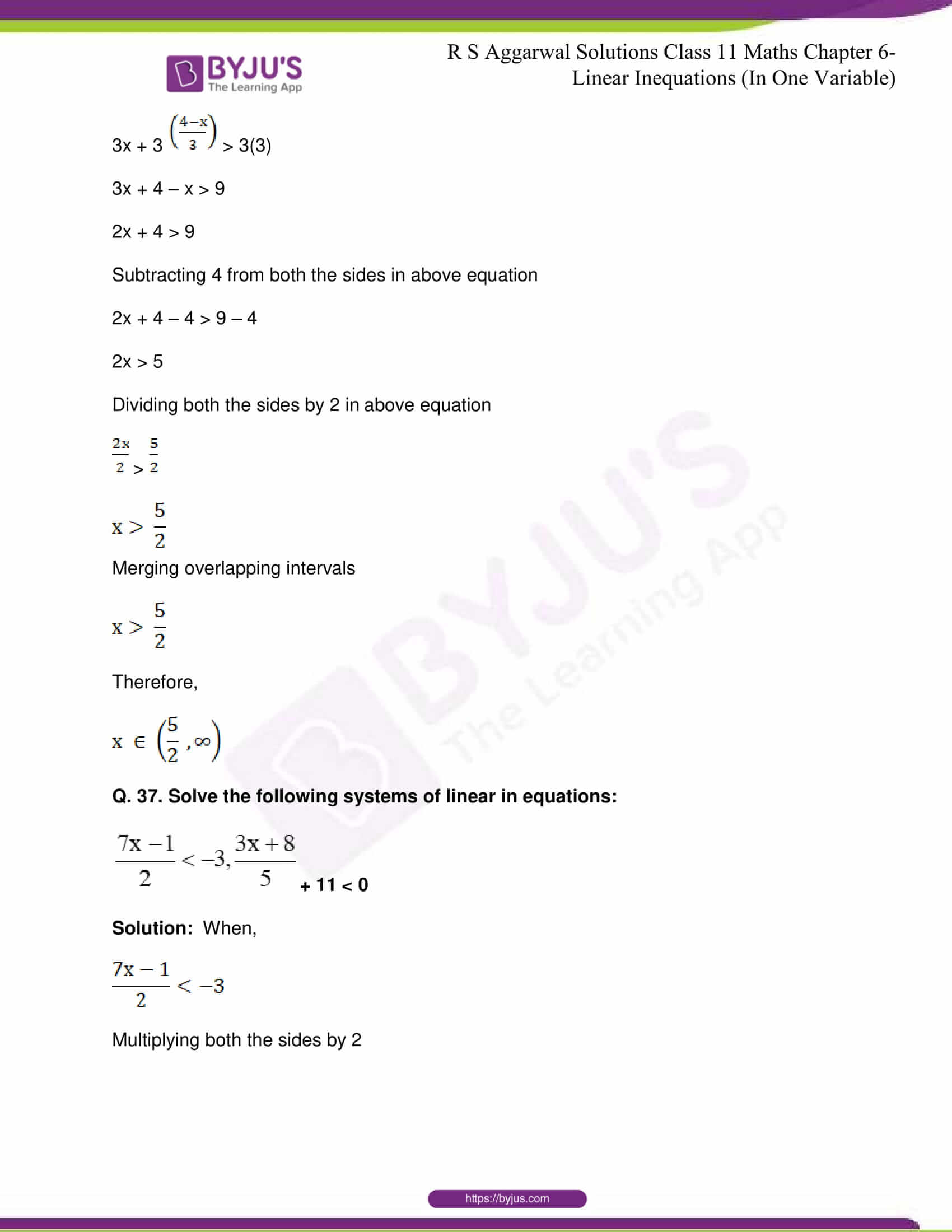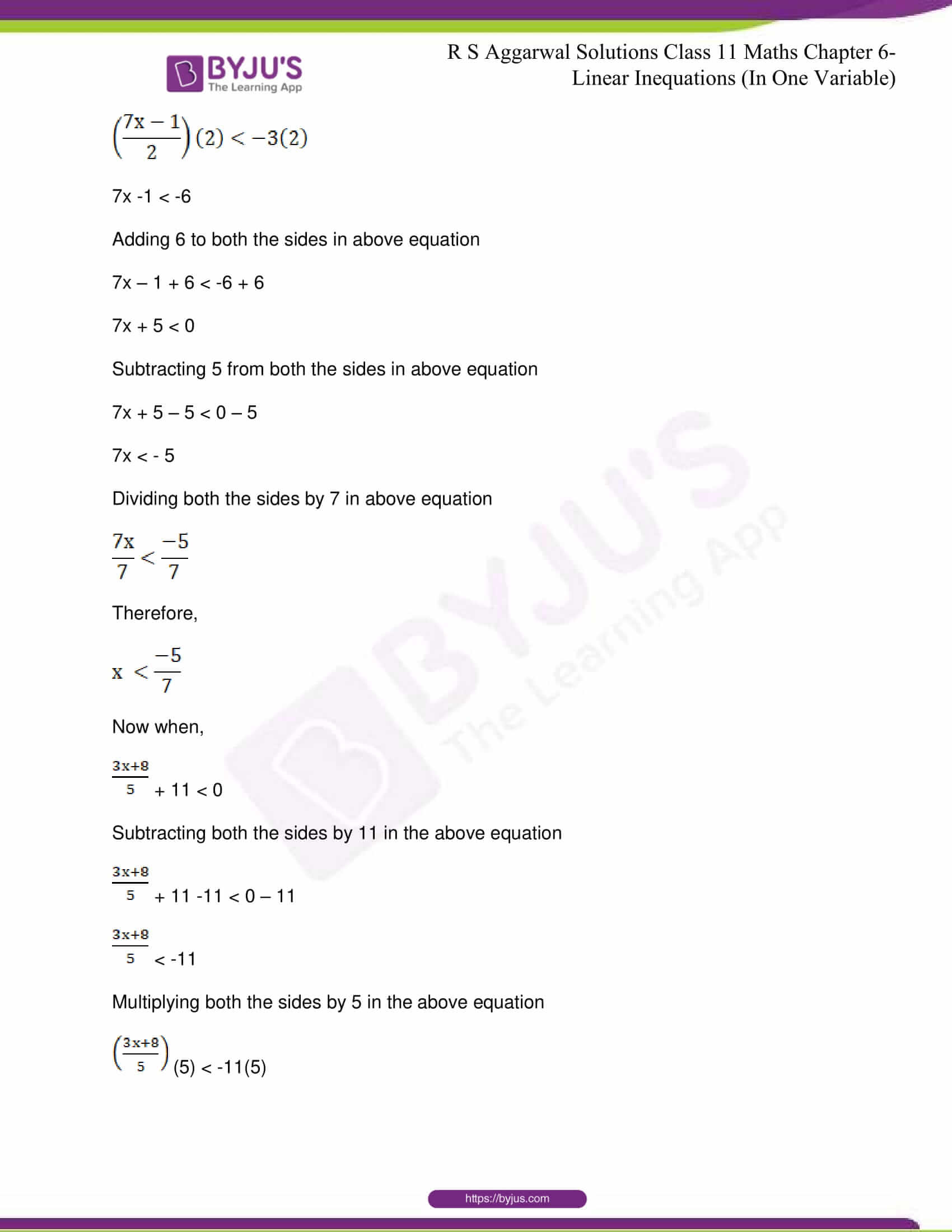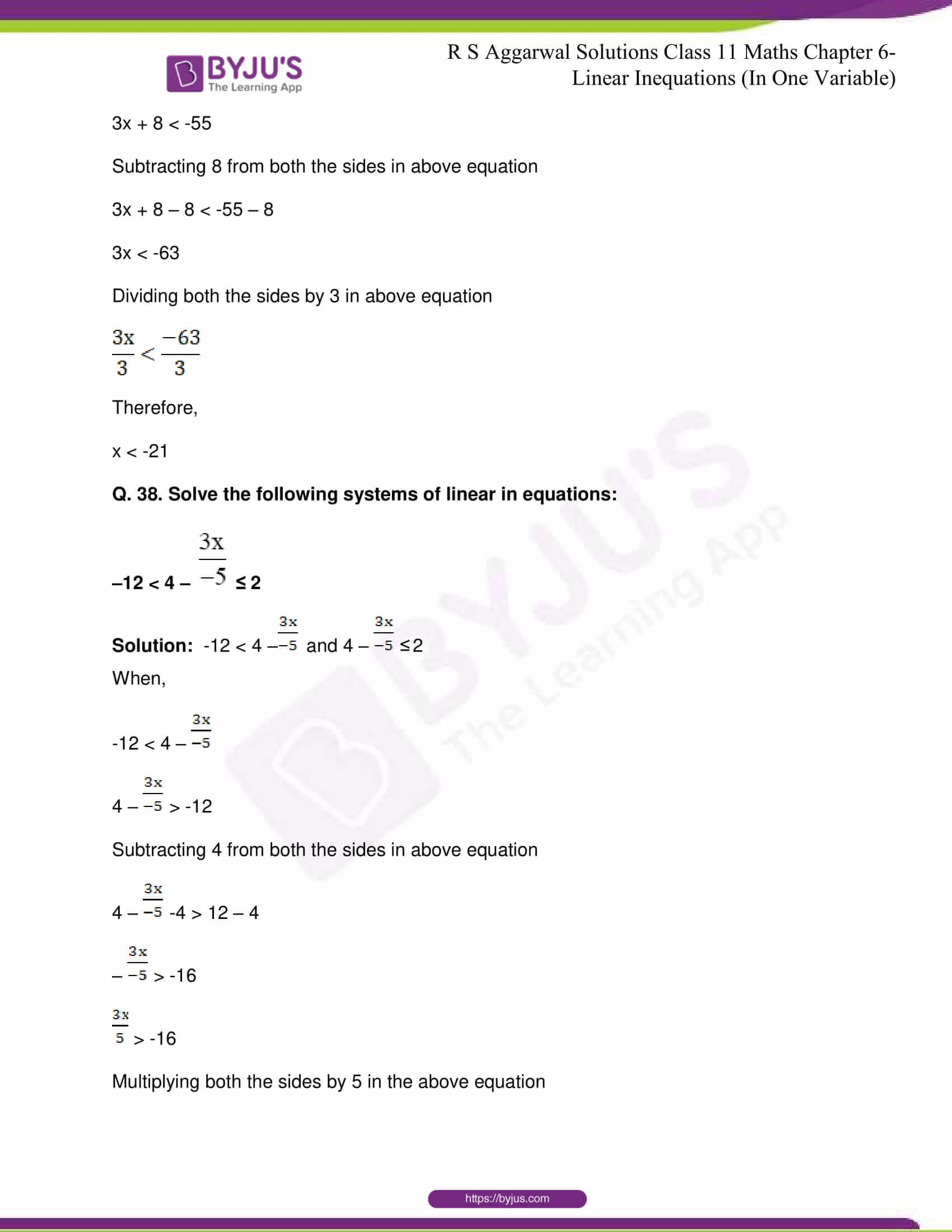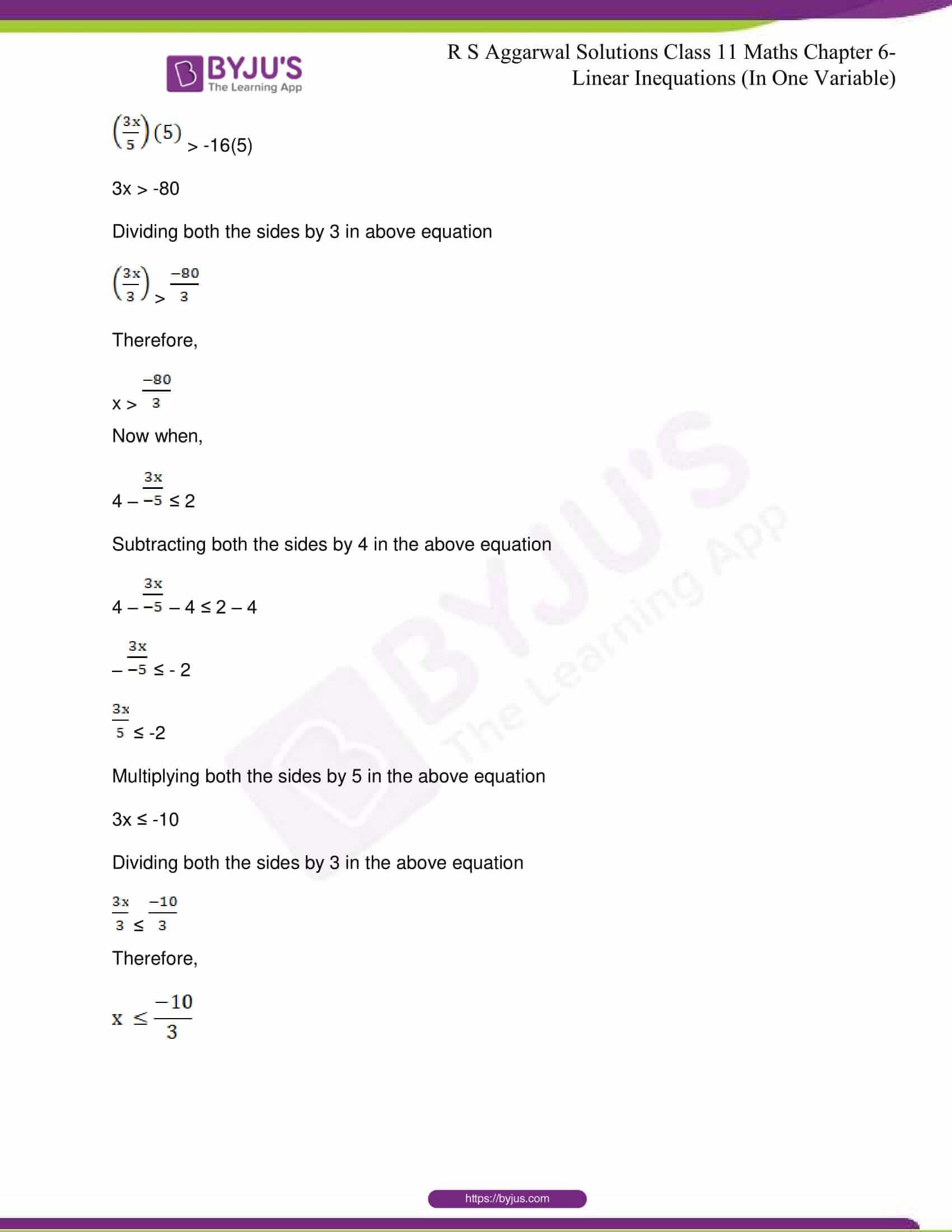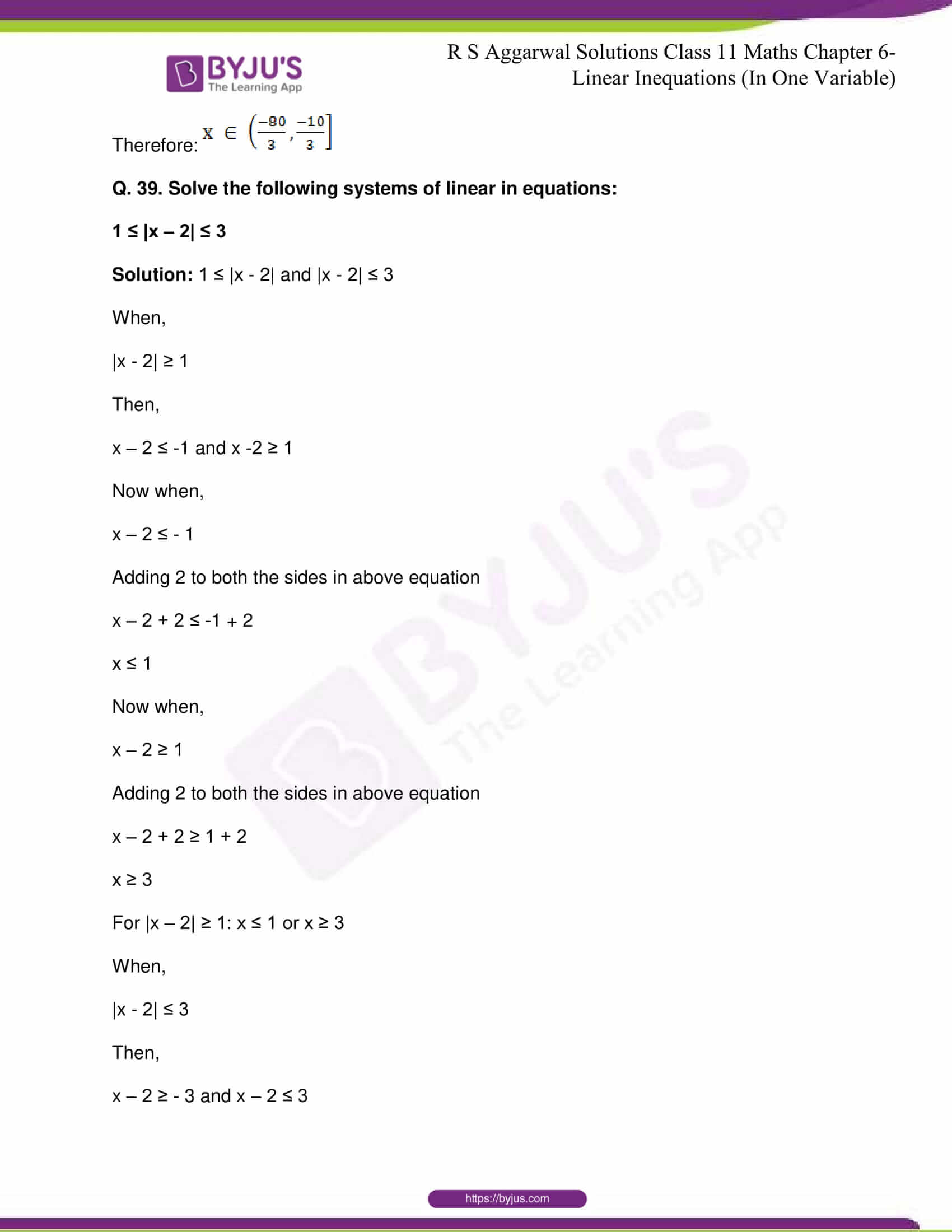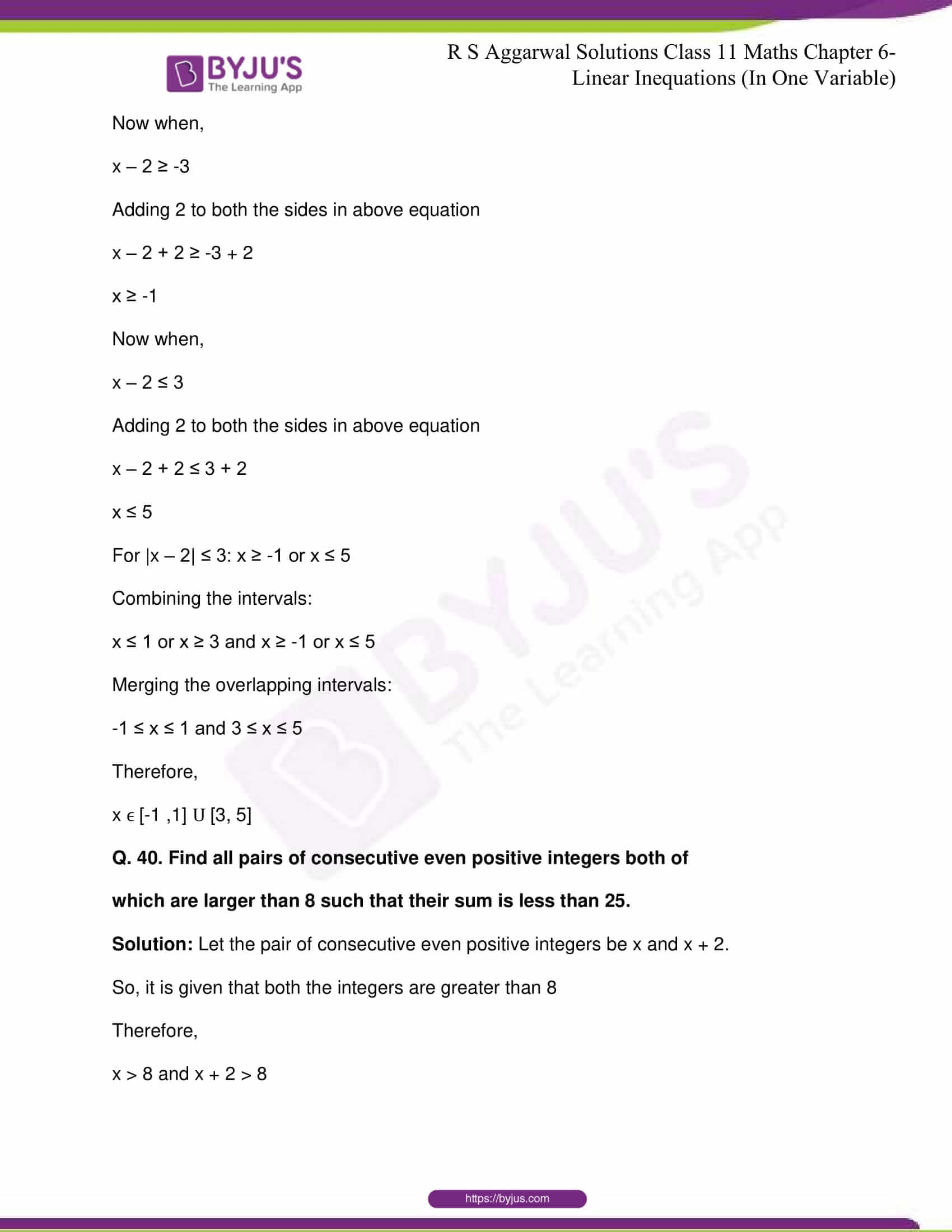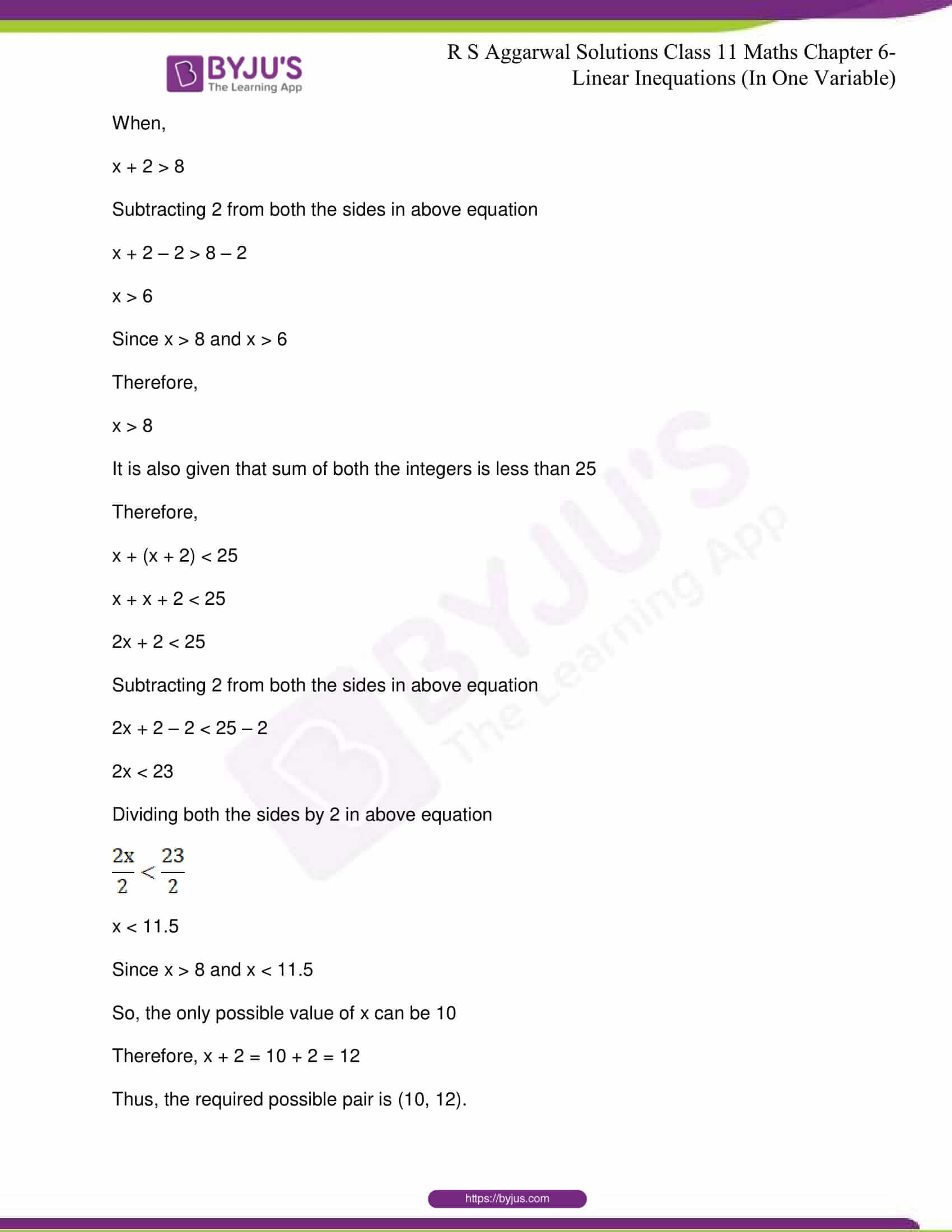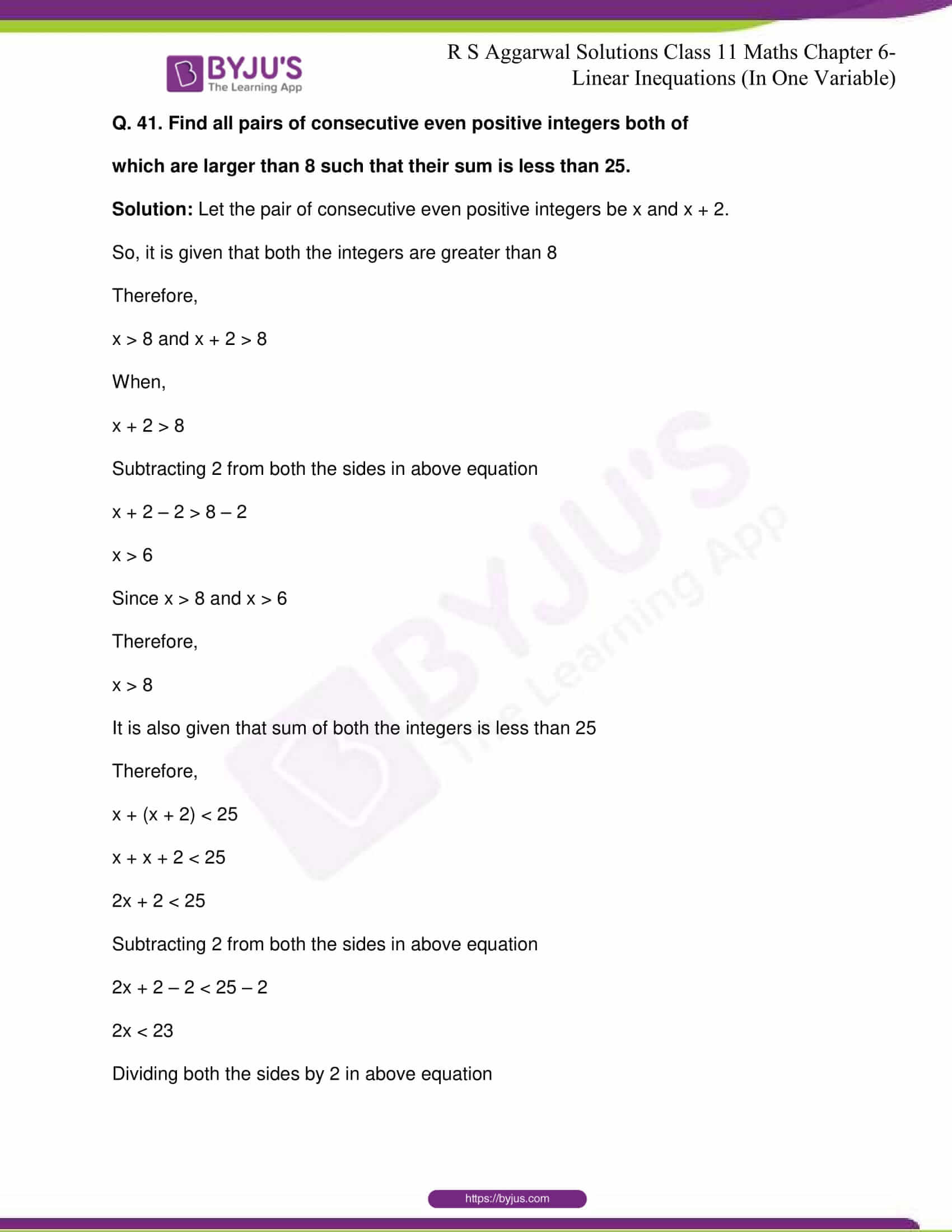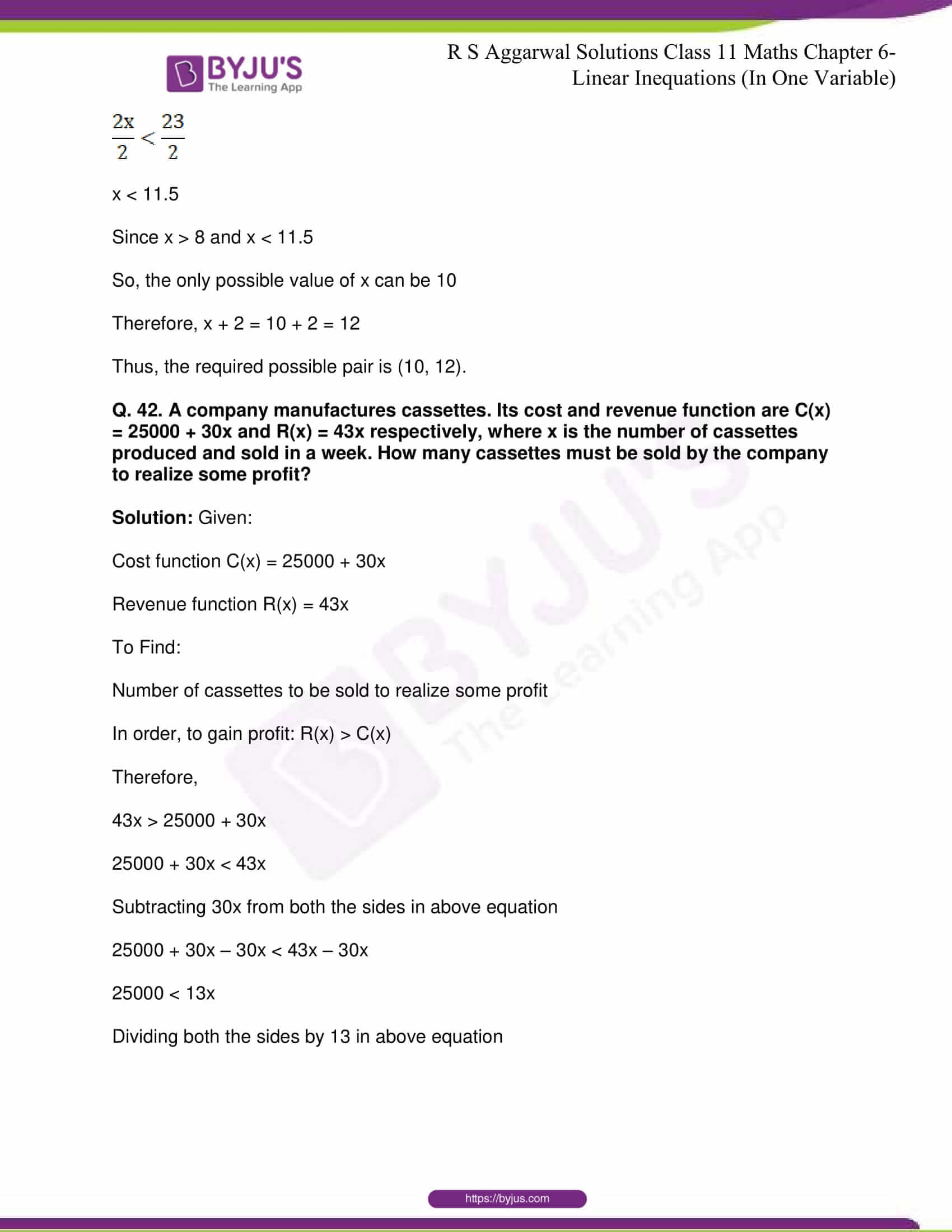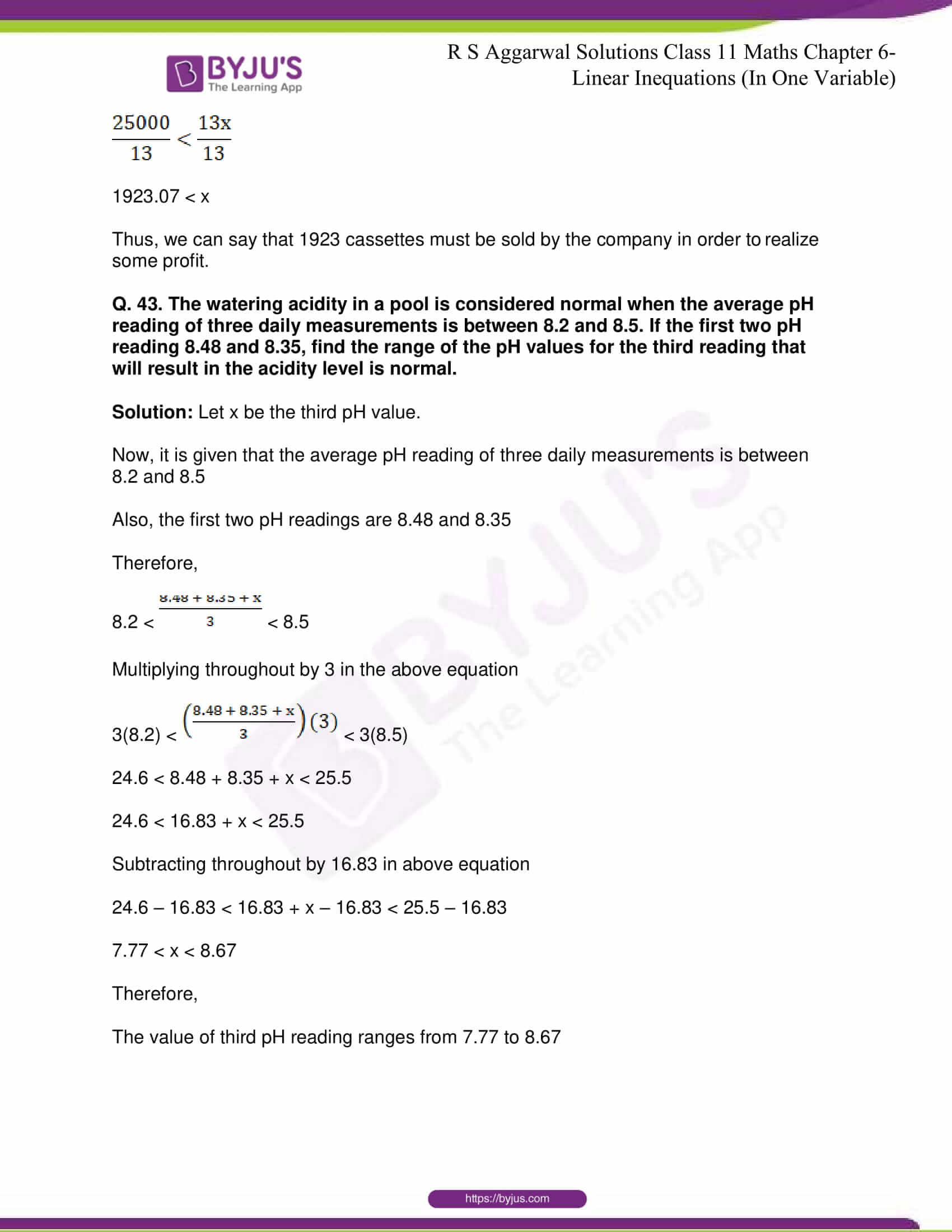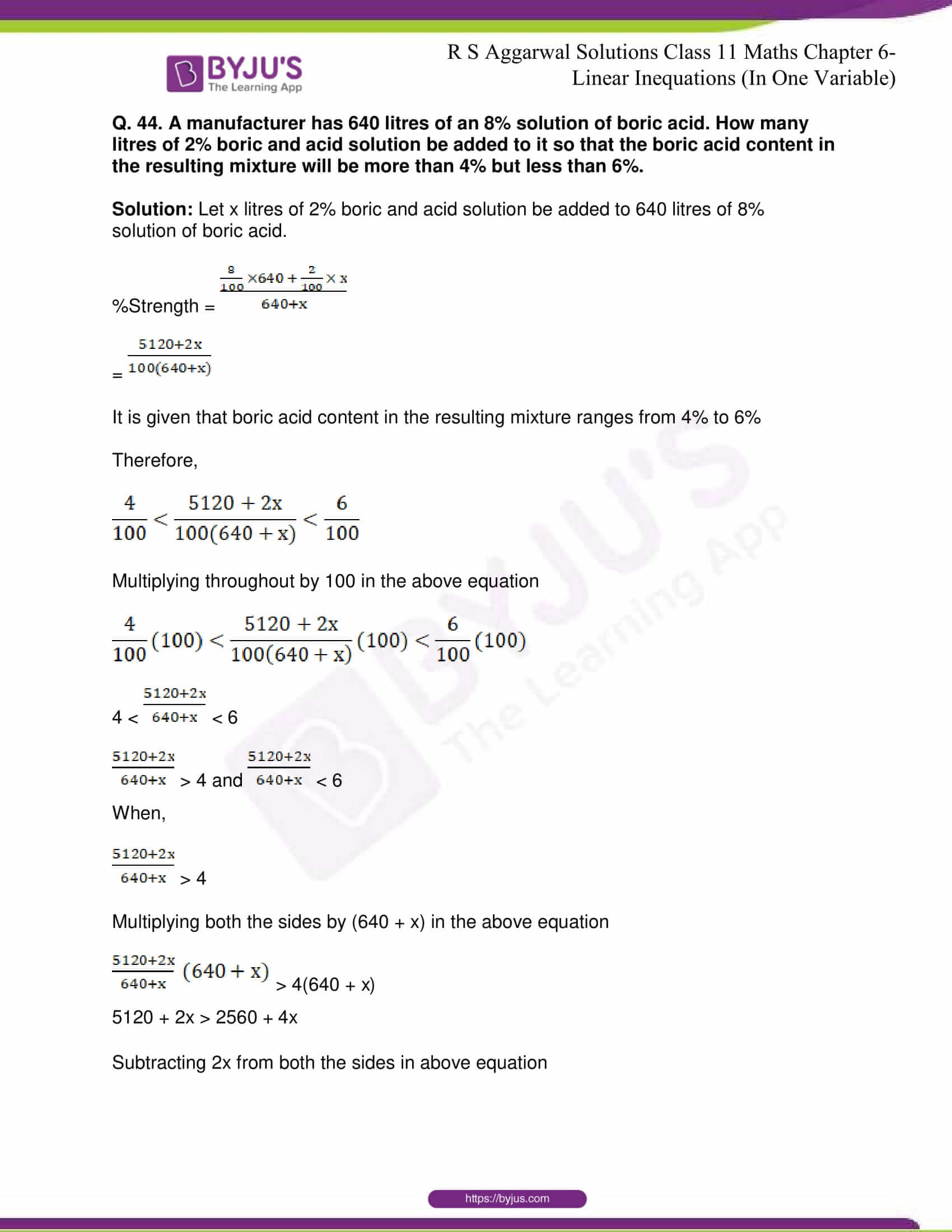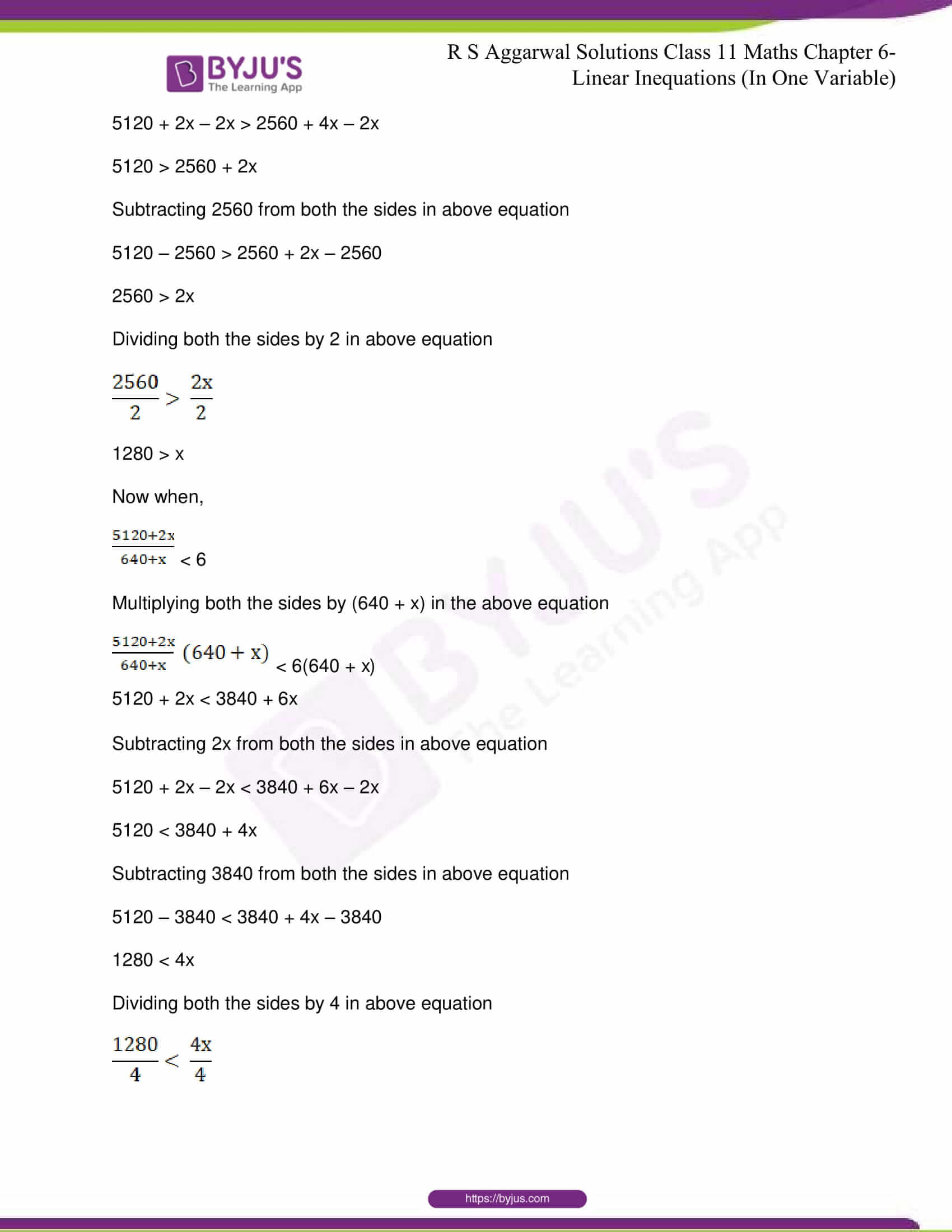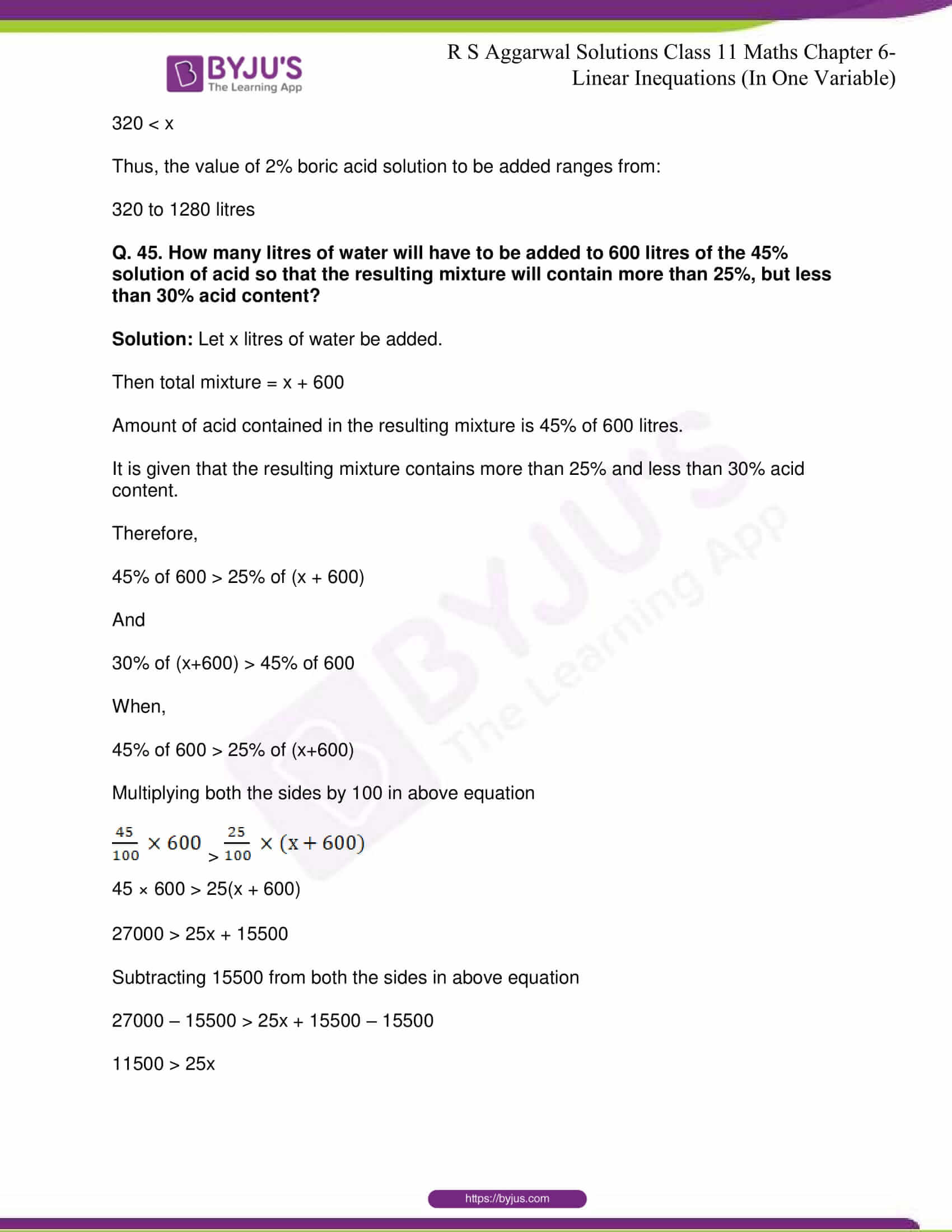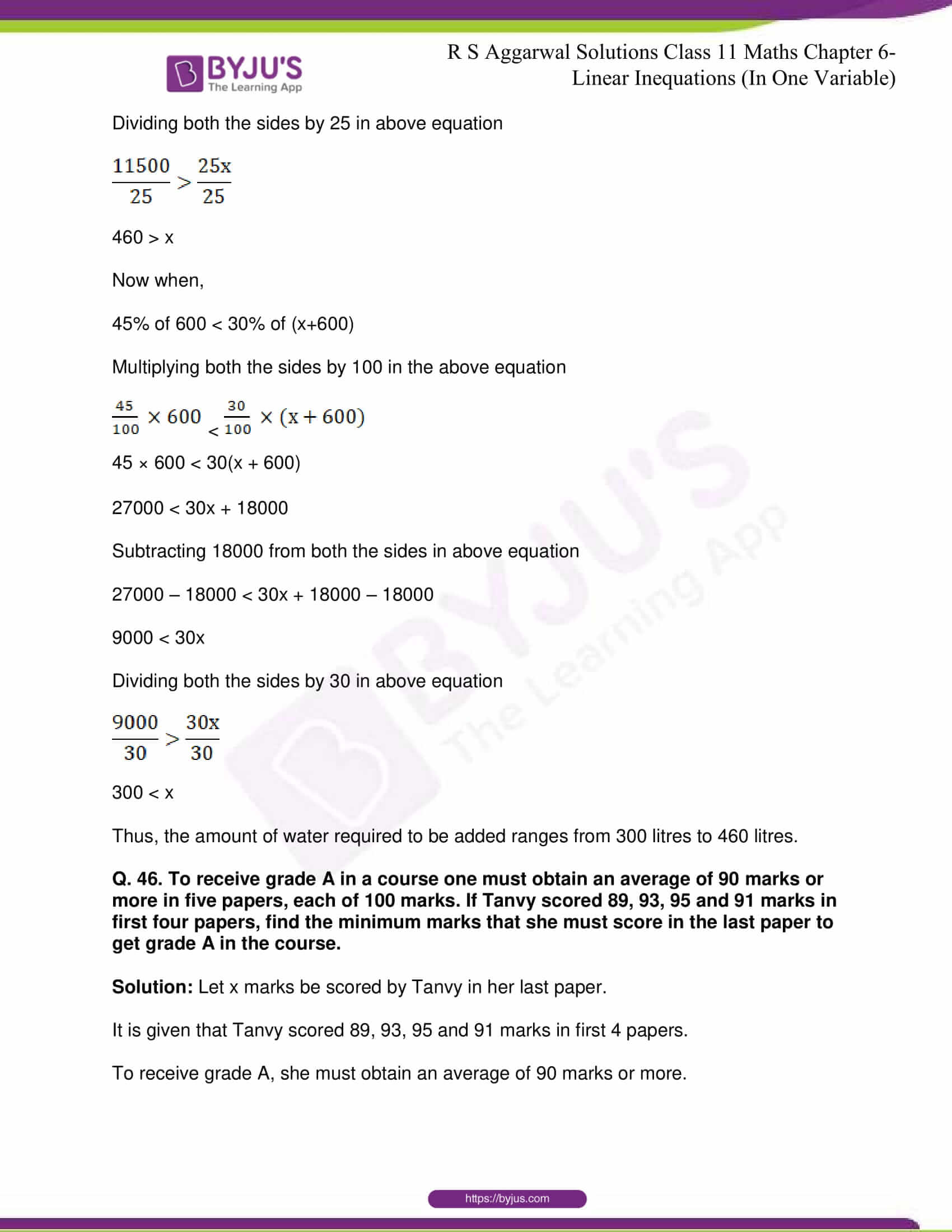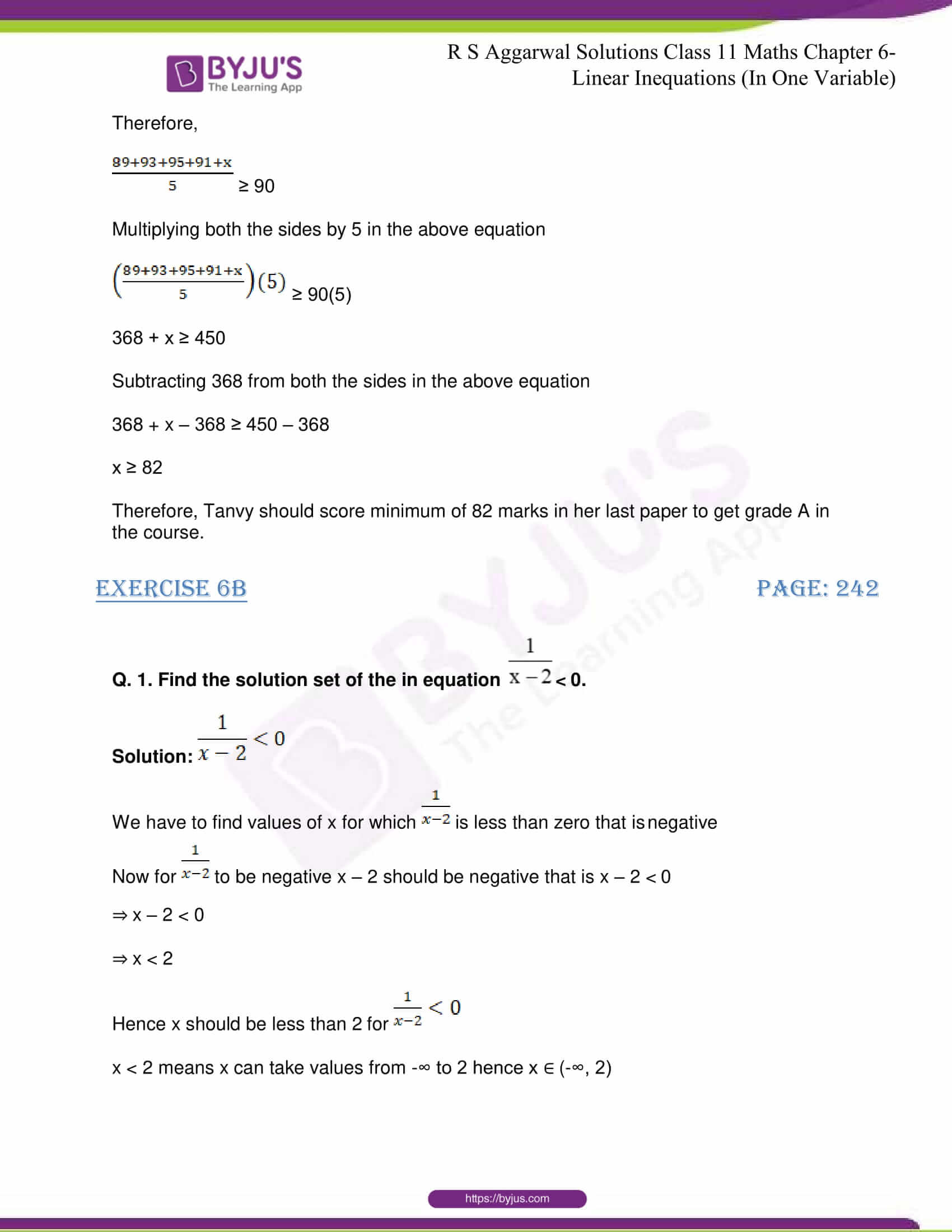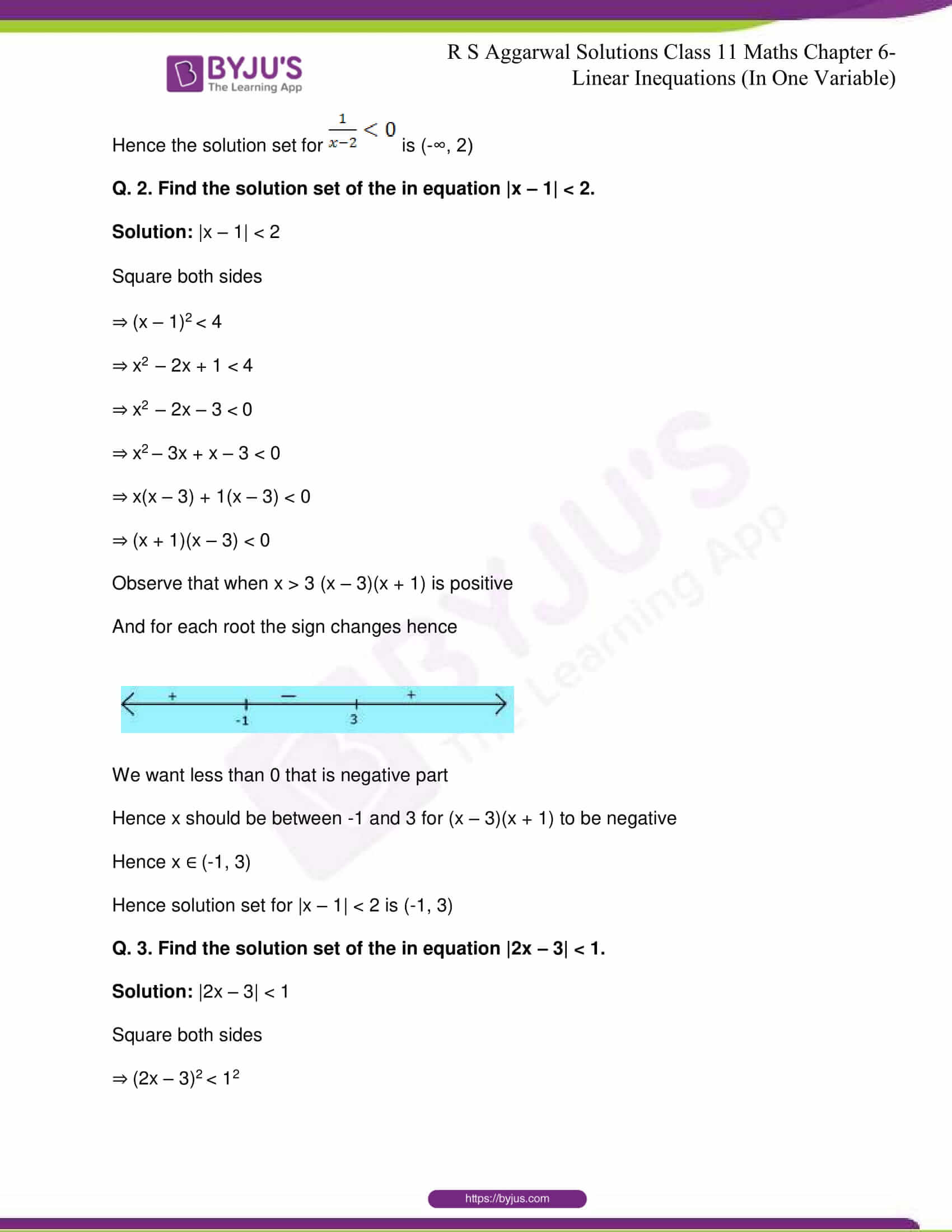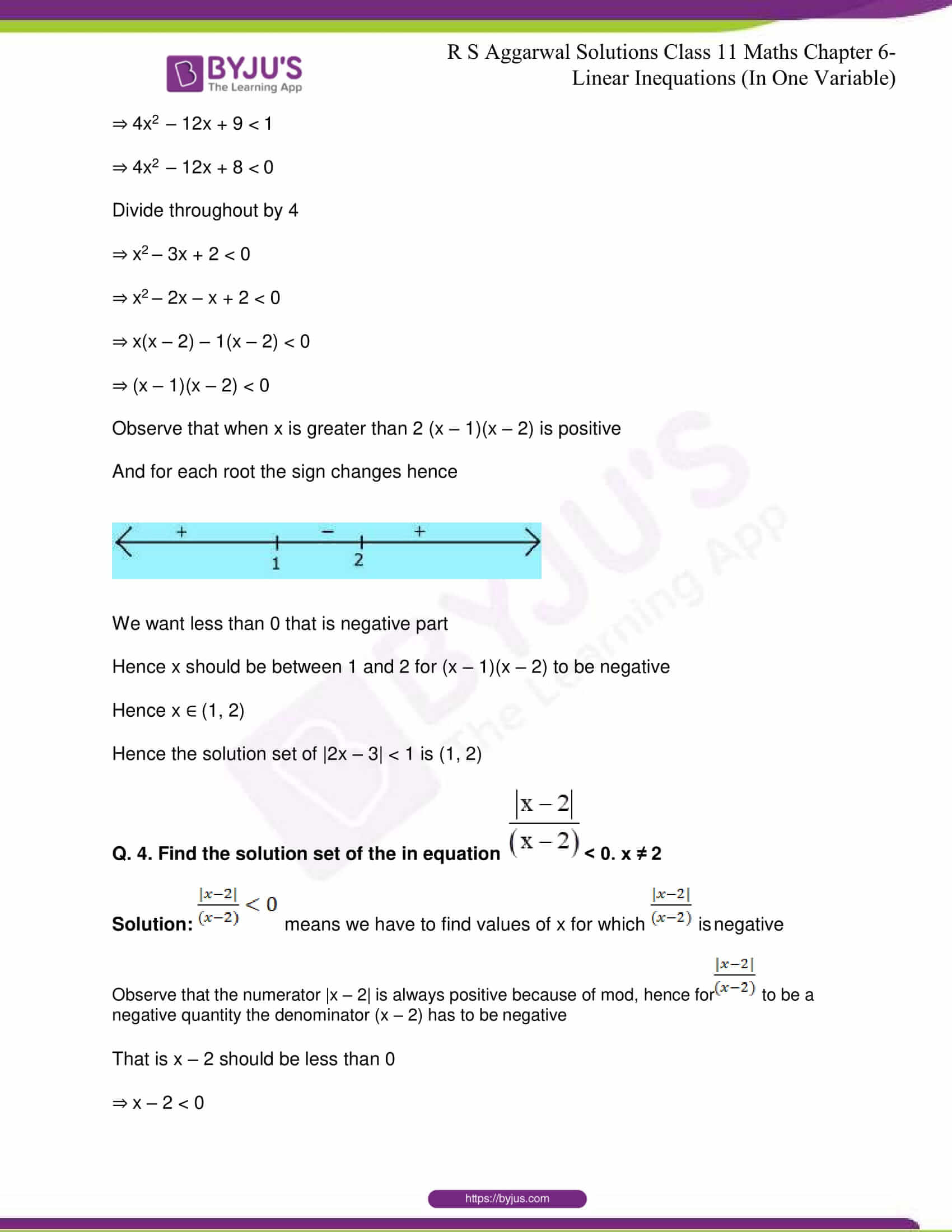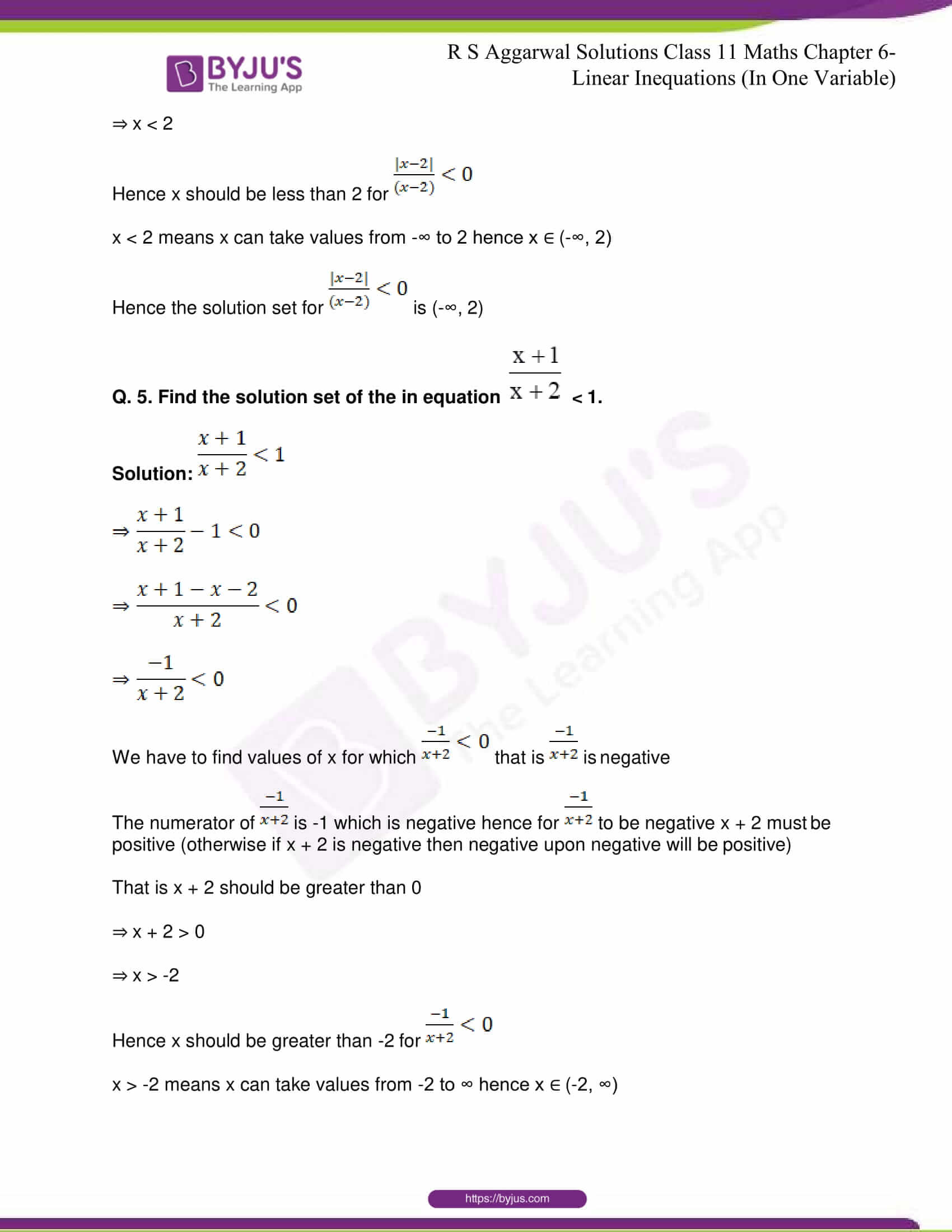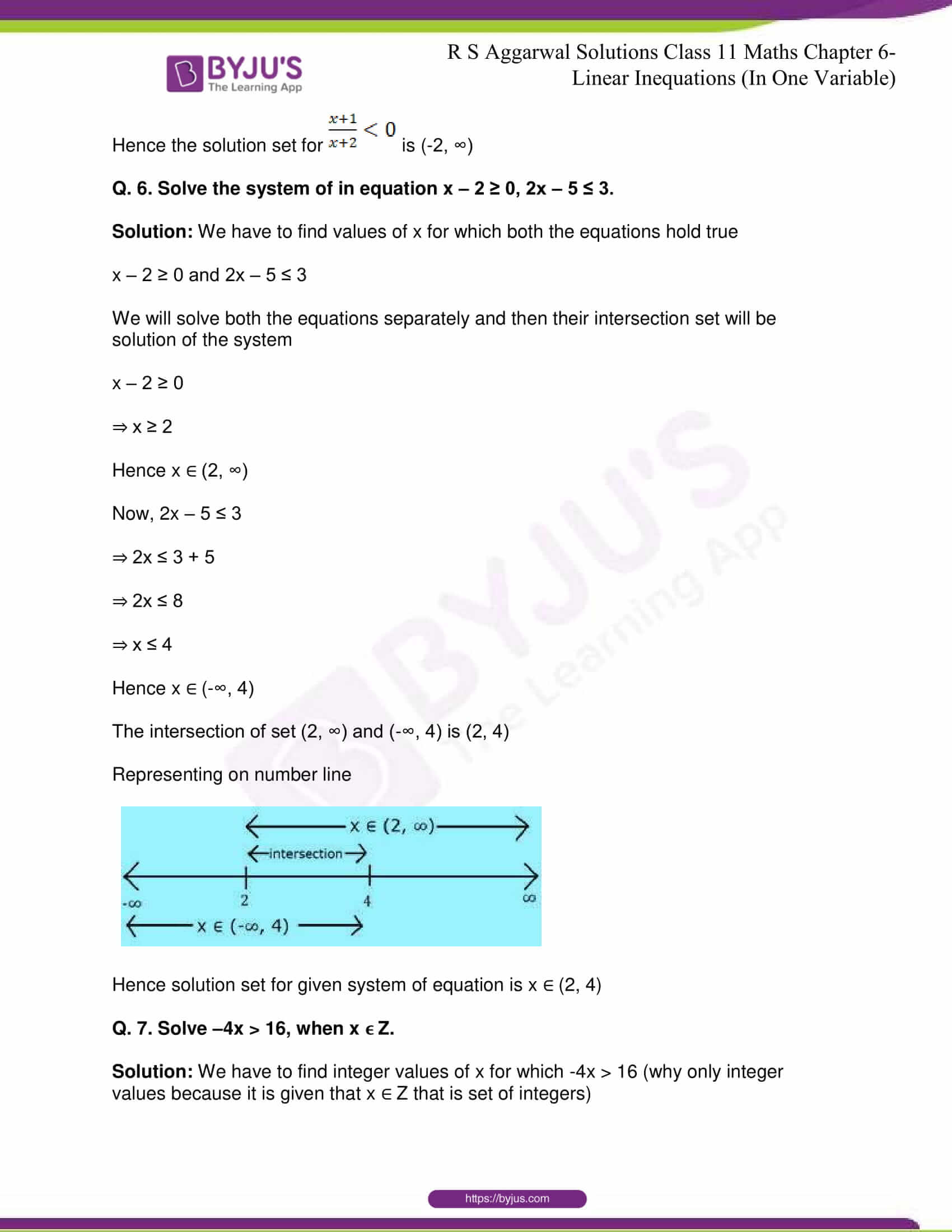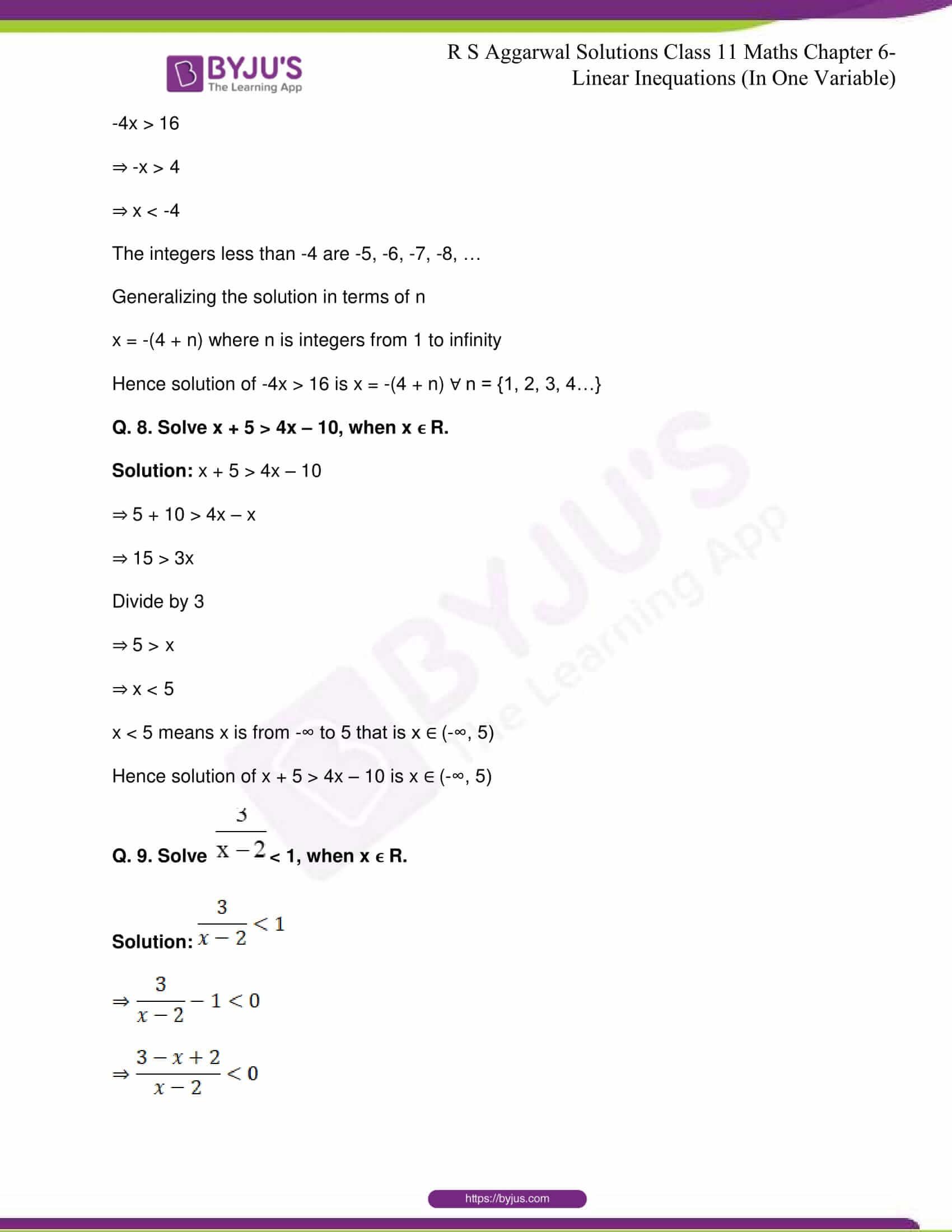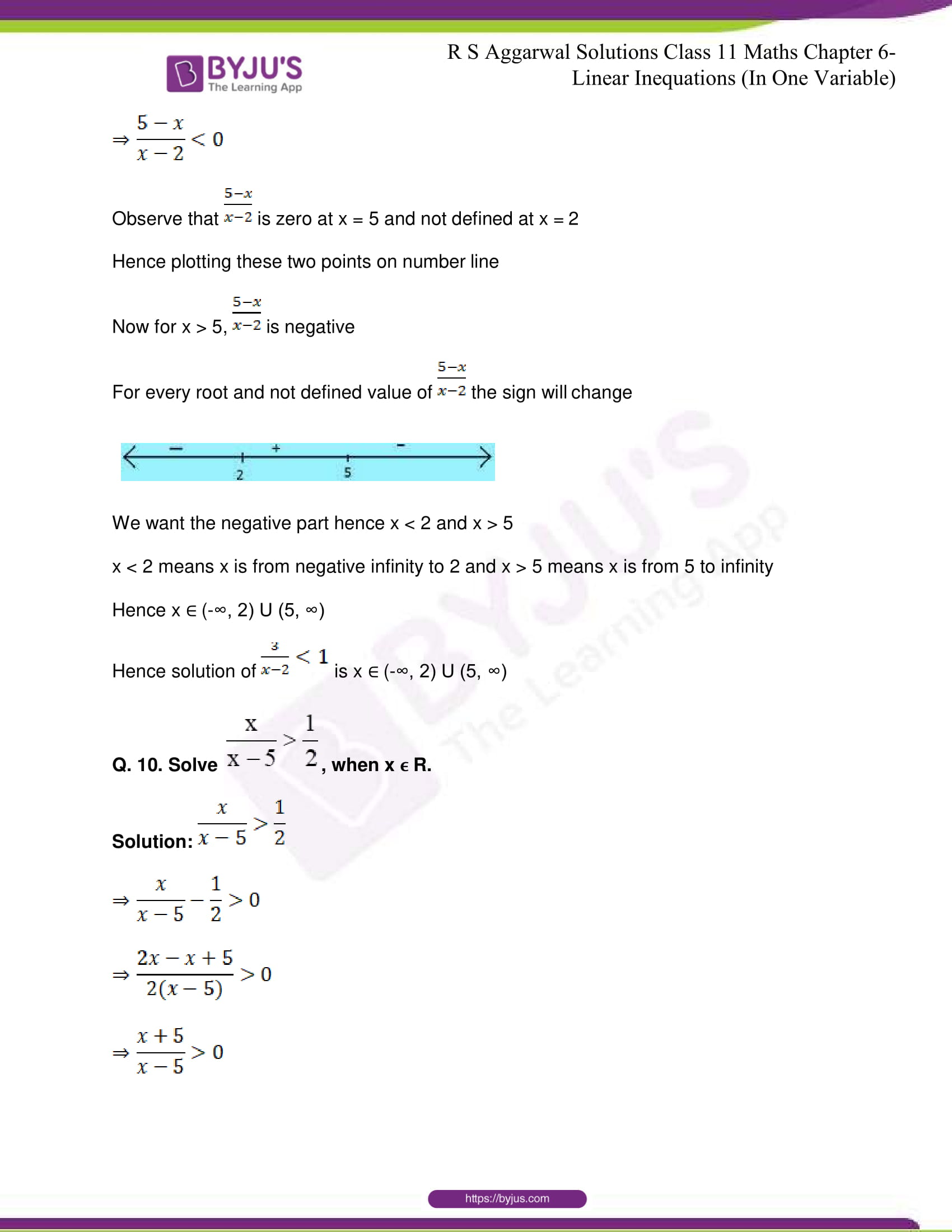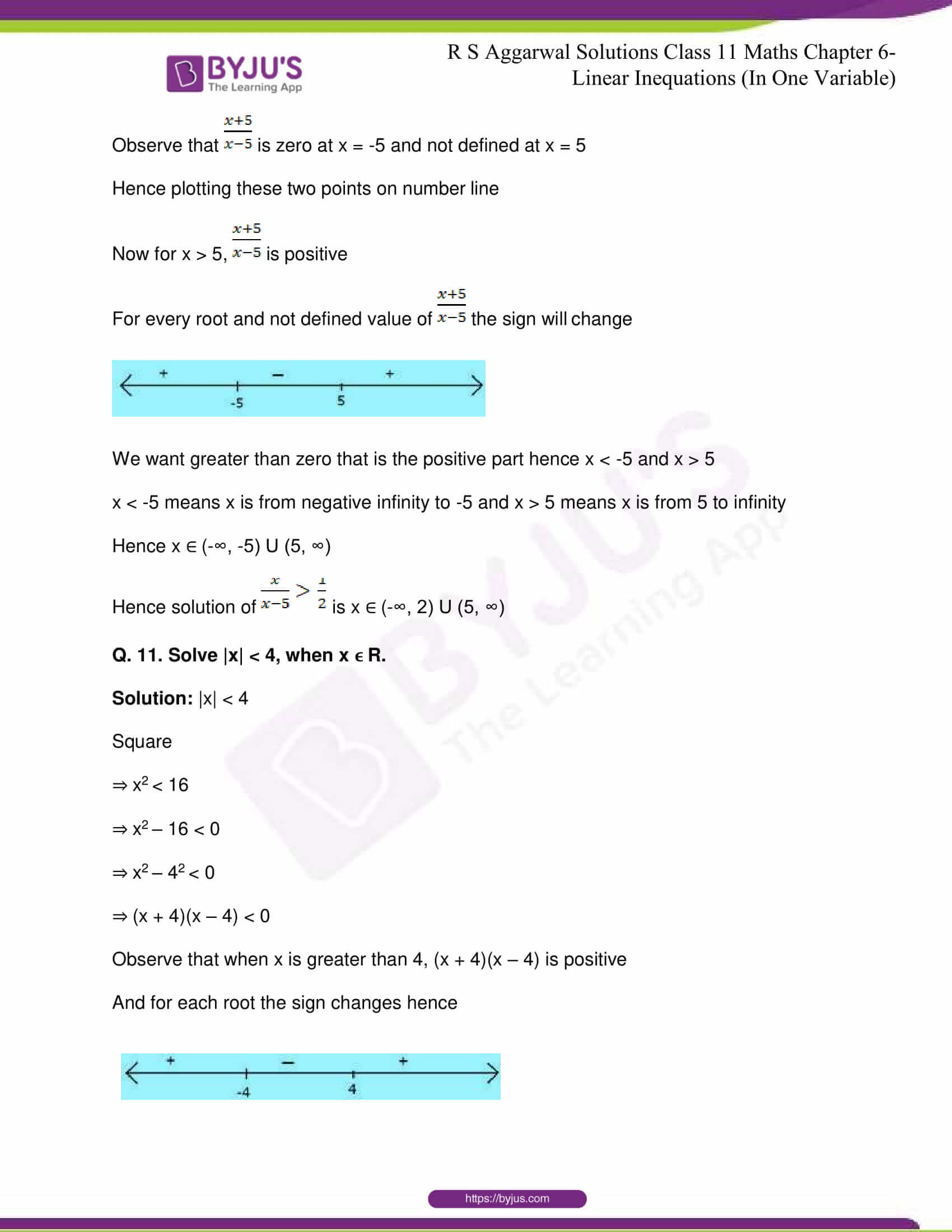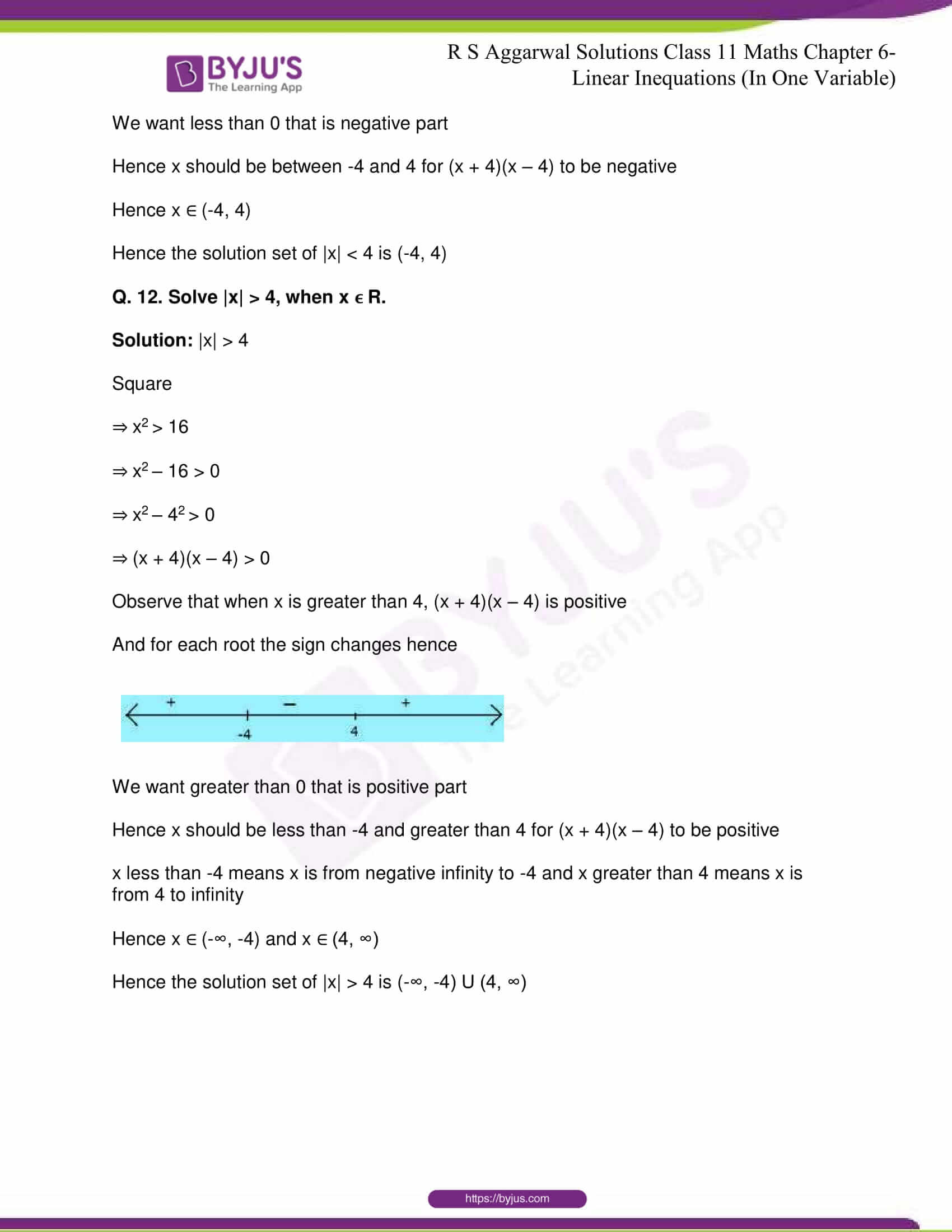## R S Aggarwal Solutions for Class 11 Maths Chapter 6- Linear Inequations (In One Variable)

Chapter 6, Linear Inequations (In One Variable), is composed of 2 exercises and the solutions given here contain answers to all the questions present in these exercises. The chapter deals with numerical problems and word problems related to linear inequations in one variable.

## R S Aggarwal Solutions for Class 11 Maths Chapter 6- Linear Inequations (In One Variable)

Mathematical expressions help us convert problem statements into entities and thus, help solve them. If the expression equates two expressions or values, then it is called an equation. An inequality which involves a linear function is a linear inequality. It looks like a linear equation, except that the ‘=’ sign is replaced by an inequality sign, called linear inequations. Read and learn the 6th chapter of R S Aggarwal textbook to get up to date with the concepts related to Complementary Angles that are taught in the class. Learn the R S Aggarwal Solutions for Class 11 effectively to score high in the examination.